JP4729812B2 - Image processing apparatus and method, recording medium, and program - Google Patents

Image processing apparatus and method, recording medium, and program Download PDF

Info

Publication number
JP4729812B2
JP4729812B2 JP2001194415A JP2001194415A JP4729812B2 JP 4729812 B2 JP4729812 B2 JP 4729812B2 JP 2001194415 A JP2001194415 A JP 2001194415A JP 2001194415 A JP2001194415 A JP 2001194415A JP 4729812 B2 JP4729812 B2 JP 4729812B2
Authority
JP
Japan
Prior art keywords
foreground
image
background
component
pixel
Prior art date
Legal status (The legal status is an assumption and is not a legal conclusion. Google has not performed a legal analysis and makes no representation as to the accuracy of the status listed.)
Expired - Fee Related
Application number
JP2001194415A
Other languages
Japanese (ja)
Other versions
JP2003009181A (en
Inventor

Original Assignee
ソニー株式会社
Priority date (The priority date is an assumption and is not a legal conclusion. Google has not performed a legal analysis and makes no representation as to the accuracy of the date listed.)
Filing date
Publication date
Application filed by ソニー株式会社 filed Critical ソニー株式会社
Priority to JP2001194415A priority Critical patent/JP4729812B2/en
Publication of JP2003009181A publication Critical patent/JP2003009181A/en
Application granted granted Critical
Publication of JP4729812B2 publication Critical patent/JP4729812B2/en
Application status is Expired - Fee Related legal-status Critical
Anticipated expiration legal-status Critical

Images

•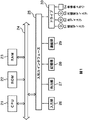•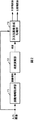••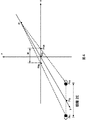•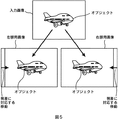•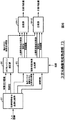•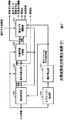•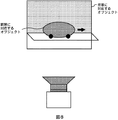•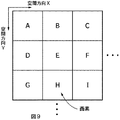•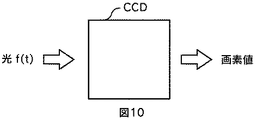•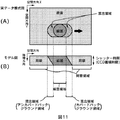••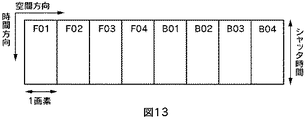•••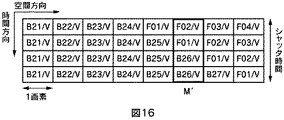••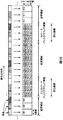•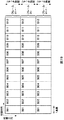•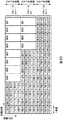•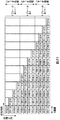•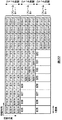•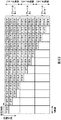•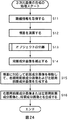•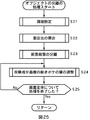••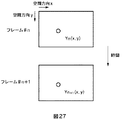•••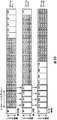•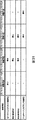•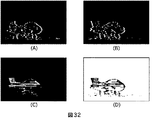•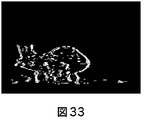•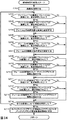•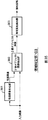••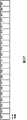••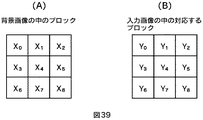••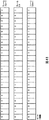•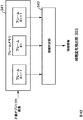•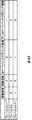•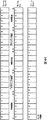•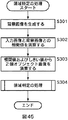•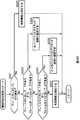•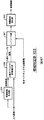•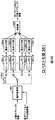•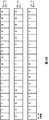•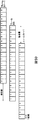•••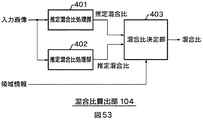•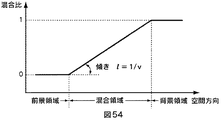•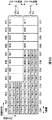•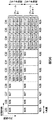•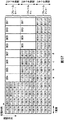••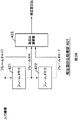•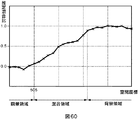•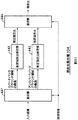•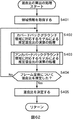••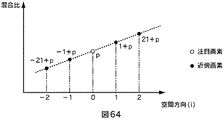•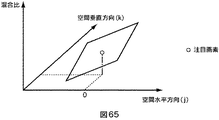••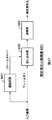•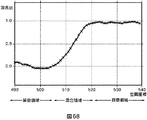•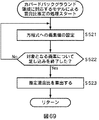•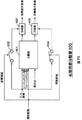••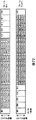•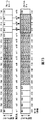•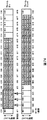•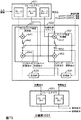•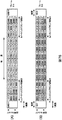•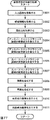•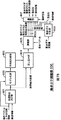•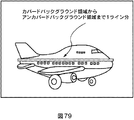•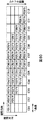•••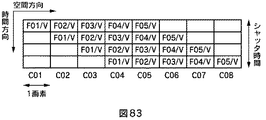••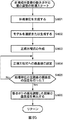••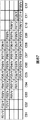•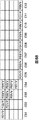•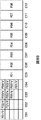•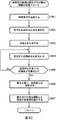•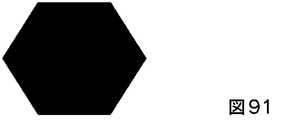••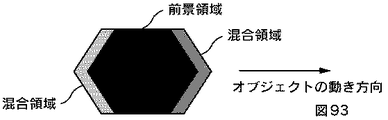•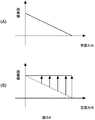•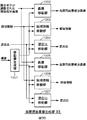•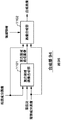Classifications

• HELECTRICITY
• H04ELECTRIC COMMUNICATION TECHNIQUE
• H04NPICTORIAL COMMUNICATION, e.g. TELEVISION
• H04N13/00Stereoscopic video systems; Multi-view video systems; Details thereof
• H04N13/20Image signal generators
• H04N13/261Image signal generators with monoscopic-to-stereoscopic image conversion
• HELECTRICITY
• H04ELECTRIC COMMUNICATION TECHNIQUE
• H04NPICTORIAL COMMUNICATION, e.g. TELEVISION
• H04N13/00Stereoscopic video systems; Multi-view video systems; Details thereof
• H04N13/10Processing, recording or transmission of stereoscopic or multi-view image signals
• H04N13/106Processing image signals
• H04N13/122Improving the 3D impression of stereoscopic images by modifying image signal contents, e.g. by filtering or adding monoscopic depth cues
• HELECTRICITY
• H04ELECTRIC COMMUNICATION TECHNIQUE
• H04NPICTORIAL COMMUNICATION, e.g. TELEVISION
• H04N13/00Stereoscopic video systems; Multi-view video systems; Details thereof
• H04N13/10Processing, recording or transmission of stereoscopic or multi-view image signals
• H04N13/106Processing image signals
• H04N13/128Adjusting depth or disparity
• HELECTRICITY
• H04ELECTRIC COMMUNICATION TECHNIQUE
• H04NPICTORIAL COMMUNICATION, e.g. TELEVISION
• H04N13/00Stereoscopic video systems; Multi-view video systems; Details thereof
• H04N13/20Image signal generators
• H04N13/275Image signal generators from 3D object models, e.g. computer-generated stereoscopic image signals
• HELECTRICITY
• H04ELECTRIC COMMUNICATION TECHNIQUE
• H04NPICTORIAL COMMUNICATION, e.g. TELEVISION
• H04N13/00Stereoscopic video systems; Multi-view video systems; Details thereof
• H04N13/10Processing, recording or transmission of stereoscopic or multi-view image signals
• H04N13/189Recording image signals; Reproducing recorded image signals
• HELECTRICITY
• H04ELECTRIC COMMUNICATION TECHNIQUE
• H04NPICTORIAL COMMUNICATION, e.g. TELEVISION
• H04N13/00Stereoscopic video systems; Multi-view video systems; Details thereof
• H04N13/10Processing, recording or transmission of stereoscopic or multi-view image signals
• H04N13/194Transmission of image signals
• HELECTRICITY
• H04ELECTRIC COMMUNICATION TECHNIQUE
• H04NPICTORIAL COMMUNICATION, e.g. TELEVISION
• H04N13/00Stereoscopic video systems; Multi-view video systems; Details thereof
• H04N13/20Image signal generators
• H04N13/204Image signal generators using stereoscopic image cameras
• H04N13/207Image signal generators using stereoscopic image cameras using a single 2D image sensor
• H04N13/221Image signal generators using stereoscopic image cameras using a single 2D image sensor using the relative movement between cameras and objects
• HELECTRICITY
• H04ELECTRIC COMMUNICATION TECHNIQUE
• H04NPICTORIAL COMMUNICATION, e.g. TELEVISION
• H04N13/00Stereoscopic video systems; Multi-view video systems; Details thereof
• H04N2013/0074Stereoscopic image analysis
• H04N2013/0081Depth or disparity estimation from stereoscopic image signals
• HELECTRICITY
• H04ELECTRIC COMMUNICATION TECHNIQUE
• H04NPICTORIAL COMMUNICATION, e.g. TELEVISION
• H04N13/00Stereoscopic video systems; Multi-view video systems; Details thereof
• H04N2013/0074Stereoscopic image analysis
• H04N2013/0092Image segmentation from stereoscopic image signals

Description


BACKGROUND OF THE INVENTION
The present invention relates to an image processing apparatus and method, a recording medium, and a program, and more particularly, to an image processing apparatus and method, a recording medium, and a program that take into consideration a difference between a signal detected by a sensor and the real world.

[Prior art]
A technique for detecting an event in the real world with a sensor and processing sampling data output from an image sensor is widely used.

For example, in an image obtained by capturing an object moving in front of a predetermined background with a video camera, motion blur occurs when the moving speed of the object is relatively fast.

There is also a technique for converting a two-dimensional image into a three-dimensional image. For example, one field screen of a two-dimensional image is divided into a plurality of regions, a horizontal parallax amount is calculated based on feature information extracted in each region, that is, depth information, and generated according to the horizontal parallax amount Displaying a three-dimensional image by displaying a first image and a second image is described in JP-A-10-51812.

[Problems to be solved by the invention]
When an object moves in front of a stationary background, not only motion blur due to mixing of the moving object image itself, but also mixing of the background image and the moving object image occurs. Conventionally, a process corresponding to the state of mixing between the background image and the moving object image has not been considered.

SUMMARY An advantage of some aspects of the invention is that a three-dimensional image can be generated from a two-dimensional image in accordance with the state of image mixing.

[Means for Solving the Problems]
The image processing apparatus of the present inventionIn an image processing apparatus for processing image data composed of a predetermined number of pixel data acquired by an imaging device having a predetermined number of pixels having a time integration effect,Image data consisting of a foreground area consisting only of the foreground object component constituting the foreground object, a background area consisting only of the background object component constituting the background object, and a mixed area obtained by mixing the foreground object component and the background object component A foreground / background component image generating means for generating a foreground component image consisting of only the foreground object component and a background component image consisting of only the background object component, and adding a right-eye parallax to the foreground component image A foreground parallax image generating means for generating a left-eye foreground parallax image by adding a left-eye parallax image to the foreground component image and generating a left-eye foreground parallax image; Combines the background image with the right-eye parallax image and combines the left-eye foreground parallax image with the background image for the left eye A parallax image producing means for producing a difference imageThe foreground / background component image generating means includes an area specifying means for specifying the foreground area, the background area, and the mixed area, and a foreground area, background area, and mixed area specifying result of the image data. , A mixture ratio detecting means for detecting a mixture ratio indicating a mixture ratio of the foreground object component and the background object component in the mixed area, and pixels belonging to the mixed area based on the detected mixed ratio, the foreground object component and the background Foreground / background separation means for generating a foreground component image and a background component image based on the separated foreground object component and background object component.It is characterized by that.

The image processing apparatus may further include a motion blur amount adjusting unit that adjusts a motion blur amount of the foreground component image generated by the foreground / background component image generating unit.

The image processing method of the present invention includes:In an image processing method for processing image data composed of a predetermined number of pixel data acquired by an imaging device having a predetermined number of pixels having a time integration effect,Image data consisting of a foreground area consisting only of the foreground object component constituting the foreground object, a background area consisting only of the background object component constituting the background object, and a mixed area obtained by mixing the foreground object component and the background object component A foreground / background component image generating step for generating a foreground / component image including only the foreground object component and a background / component image including only the background object component, and adding a right-eye parallax to the foreground component image Generating a foreground parallax image for the eye, adding a parallax for the left eye to the foreground component image to generate a foreground parallax image for the left eye, and a foreground parallax image for the right eye and an arbitrary Combines the background image with the right-eye parallax image and combines the left-eye foreground parallax image with the background image. A parallax image generation step of generating a parallax image for the left eyeAnd the foreground / background component image generation step identifies the foreground region, the background region, and the mixed region of the image data, and based on the specific results of the foreground region, the background region, and the mixed region, A mixture ratio indicating a mixture ratio of the foreground object component and the background object component is detected, and based on the detected mixture ratio, the pixels belonging to the mixed area are separated into the foreground object component and the background object component and separated. Generating foreground and background component images based on the foreground and background object componentsIt is characterized by.

The program of the recording medium of the present invention isIn a computer that processes image data consisting of a predetermined number of pixel data acquired by an imaging device having a predetermined number of pixels having a time integration effect,Image data consisting of a foreground area consisting only of the foreground object component constituting the foreground object, a background area consisting only of the background object component constituting the background object, and a mixed area obtained by mixing the foreground object component and the background object component A foreground / background component image generating step for generating a foreground / component image including only the foreground object component and a background / component image including only the background object component, and adding a right-eye parallax to the foreground component image Generating a foreground parallax image for the eye, adding a parallax for the left eye to the foreground component image to generate a foreground parallax image for the left eye, and a foreground parallax image for the right eye and an arbitrary Combines the background image with the right-eye parallax image and combines the left-eye foreground parallax image with the background image. A parallax image generation step of generating a parallax image for the left eyeAnd the foreground / background component image generation step identifies the foreground region, the background region, and the mixed region of the image data, and based on the specific results of the foreground region, the background region, and the mixed region, A mixture ratio indicating a mixture ratio of the foreground object component and the background object component is detected, and based on the detected mixture ratio, the pixels belonging to the mixed area are separated into the foreground object component and the background object component and separated. A computer-readable recording medium storing a program for executing a process for generating a foreground component image and a background component image based on the foreground object component and the background object component..

The program of the present inventionProcess image data consisting of a predetermined number of pixel data acquired by an imaging device having a predetermined number of pixels having a time integration effectThe computer includes a foreground area consisting only of the foreground object component constituting the foreground object, a background area consisting only of the background object component constituting the background object, and a mixed area where the foreground object component and the background object component are mixed. A foreground / background component image generating step for generating a foreground / component image consisting only of the foreground object component and a background / component image consisting only of the background object component, and adding a right-eye parallax to the foreground component image A foreground parallax image generating step for generating a left-eye foreground parallax image by adding a left-eye parallax image to the foreground component image and generating a left-eye foreground parallax image. Is combined with an arbitrary background image to generate a right-eye parallax image and a left-eye foreground parallax image A parallax image generation step of generating a parallax image for the left eye synthesizes the imageAnd the foreground / background component image generation step identifies the foreground region, the background region, and the mixed region of the image data, and based on the specific results of the foreground region, the background region, and the mixed region, A mixture ratio indicating a mixture ratio of the foreground object component and the background object component is detected, and based on the detected mixture ratio, the pixels belonging to the mixed area are separated into the foreground object component and the background object component and separated. Processing to generate foreground and background component images based on the foreground and background object componentsIs executed.

In the image processing apparatus and method, the recording medium, and the program according to the present invention, the foreground area composed only of the foreground object component constituting the foreground object, the background area composed solely of the background object component constituting the background object, and the foreground object component And a foreground component image including only the foreground object component and a background component image including only the background object component are generated from the image data including the mixed region obtained by mixing the background object component and the foreground component image. The right-eye foreground parallax image is generated by adding the right-eye parallax image, and the left-eye foreground parallax image is generated by adding the left-eye parallax to the foreground component image. A parallax image for the right eye is generated by combining the parallax image and an arbitrary background image, and a foreground parallax image for the left eye The parallax image for the left eye is generated by combining the background image and.In the generation of the foreground component image and the background component image, the foreground region, the background region, and the mixed region of the image data are specified. Based on the specific results of the foreground region, the background region, and the mixed region, A mixing ratio indicating a mixing ratio of the foreground object component and the background object component is detected, and pixels belonging to the mixed area are separated into the foreground object component and the background object component based on the detected mixing ratio. Based on the foreground object component and the background object component, a foreground component image and a background component image are generated.

DETAILED DESCRIPTION OF THE INVENTION
FIG. 1 is a diagram showing an embodiment of an image processing apparatus according to the present invention. A CPU (Central Processing Unit) 21 executes various processes according to a program stored in a ROM (Read Only Memory) 22 or a storage unit 28. A RAM (Random Access Memory) 23 appropriately stores programs executed by the CPU 21 and data. The CPU 21, ROM 22, and RAM 23 are connected to each other by a bus 24.

An input / output interface 25 is also connected to the CPU 21 via the bus 24. The input / output interface 25 is connected to an input unit 26 including a keyboard, a mouse, and a microphone, and an output unit 27 including a display and a speaker. The CPU 21 executes various processes in response to commands input from the input unit 26. Then, the CPU 21 outputs an image, sound, or the like obtained as a result of the processing to the output unit 27.

The storage unit 28 connected to the input / output interface 25 is configured by, for example, a hard disk and stores programs executed by the CPU 21 and various data. The communication unit 29 communicates with an external device via the Internet or other networks. In this example, the communication unit 29 functions as an acquisition unit that captures the output of the sensor.

A program may be acquired via the communication unit 29 and stored in the storage unit 28.

The drive 30 connected to the input / output interface 25, when a magnetic disk 51, an optical disk 52, a magneto-optical disk 53, or a semiconductor memory 54 is mounted, drives them, and programs and data recorded there. Get etc. The acquired program and data are transferred to and stored in the storage unit 28 as necessary.

FIG. 2 is a block diagram illustrating a configuration of the image processing apparatus.

It does not matter whether each function of the image processing apparatus is realized by hardware or software. That is, each block diagram in this specification may be considered as a hardware block diagram or a software functional block diagram.

In this specification, an image corresponding to an object in the real world to be imaged is referred to as an image object.

The input image input to the image processing apparatus is supplied to the distance information acquisition unit 71 and the three-dimensional image composition processing unit 73.

The distance information acquisition unit 71 acquires the distance from the sensor to the object corresponding to the foreground based on the input image.

In a general image, the object corresponding to the foreground is positioned in front of the background object, and the focus of the sensor is matched to the object corresponding to the foreground. Therefore, the image of the object corresponding to the foreground includes a lot of high frequency components compared to the image of the background object. Similarly, the brightness and saturation of the object image corresponding to the foreground are higher than those of the background object image.

The distance from the sensor to the object can be obtained by multiplying the amount of high-frequency components contained in the object image, or the luminance or saturation of the object image by a predetermined coefficient.

The distance information acquisition unit 71 generates distance information indicating the distance to the object based on, for example, the amount of high-frequency components included in the object image and the brightness and saturation of the object image.

The distance information acquisition unit 71 generates distance information indicating the distance from the sensor to the object corresponding to the foreground, and supplies the generated distance information to the parallax calculation unit 72.

The parallax calculation unit 72 calculates the parallax corresponding to the foreground object based on the distance information supplied from the distance information acquisition unit 71 and supplies the calculated parallax to the three-dimensional image composition processing unit 73.

The three-dimensional image composition processing unit 73 converts the input image into a foreground component image consisting of only image components corresponding to the foreground object (hereinafter also referred to as foreground components) and image components corresponding to the background objects (hereinafter referred to as “foreground components”). , Which are also referred to as background components). Based on the foreground component image, the background component image, and the parallax supplied from the parallax calculation unit 72, the three-dimensional image composition processing unit 73 performs a right-eye image (also referred to as a right-eye parallax image) and a left-eye image ( Left parallax image), and the generated right eye image and left eye image are output.

Next, a three-dimensional image and parallax will be described.

As shown in FIG. 3, when the object is viewed in the real world, the position of the object seen by the right eye and the position of the object seen by the left eye differ according to the distance to the object. The difference between the position of the object visible to the right eye and the position of the object visible to the left eye is referred to as parallax.

In the three-dimensional image, the image of the object for the right eye displayed at the position corresponding to the parallax is shown to the right eye, and the image of the object for the left eye displayed at the position corresponding to the parallax is By showing it to the eyes, the user can recognize the distance to the object.

The parallax will be described in more detail with reference to FIG.

In FIG. 4, x indicates the horizontal coordinate of the display surface, y indicates the depth coordinate perpendicular to the display surface, and z indicates the vertical coordinate of the display surface.

P0 indicates the center position of the eyes of the observer (user).

E indicates the length between the eyes. PER indicates the position of the right eye, and PEL indicates the position of the left eye. The coordinates of PER are represented by (X0, Y0, Z0), and the coordinates of PEL are represented by (X1, Y1, Z1).

PD indicates the intersection of the display surface and the visual axis. The coordinates of PD are represented by (Xd, Yd, Zd).

PDR indicates the image presentation position for the left eye, and PDL indicates the image presentation position for the right eye. The coordinates of the PDR are represented by (X2, Y2, Z2), and the coordinates of the PDL are represented by (X3, Y3, Z3).

P indicates the position of the displayed object. The coordinates of P are represented by (Xp, Yp, Zp).

W represents the parallax on the display surface of the object.

The coordinates P (Xp, Yp, Zp) of the object perceived by the observer are the line segment Lr connecting the right-eye coordinates PER and the right-eye image presentation position PDL presented on the display surface, and the left-eye It is obtained as the intersection of the coordinate PEL and the line segment Ll connecting the left-eye image presentation position PDR presented on the display surface.

The line segment Lr is expressed by equation (1), and the line segment Ll is expressed by equation (2).
(x-X0) / (X2-X0) = (y-Y0) / (Y2-Y0) = (z-Z0) / (Z2-Z0) (1)
(x-X1) / (X3-X1) = (y-Y1) / (Y3-Y1) = (z-Z1) / (Z3-Z1) (2)

Accordingly, the coordinates of P, which is the intersection of the line segment Lr and the line segment Ll, are expressed by equations (3) to (5).
Xp = Tfix- (X2-X0) + X0 (3)
Yp = Tfix- (Y2-Y0) + Y0 (4)
Zp = Tfix- (Z2-Z0) + Z0 (5)
Tfix in Expressions (3) to (5) is expressed by Expression (6).

Next, consider an image viewed from a position separated by a distance K in the y direction from the center of the display surface. If the coordinates of the intersection of the display surface and the visual axis are the origin of the X, Y, and Z coordinates, the coordinates of P0, PD, PER, PEL, PDR, and PDL are as follows: Can be expressed as

That is, the coordinates of P0 can be expressed as (0, K, 0). The coordinates (Xd, Yd, Zd) of the PD can be expressed as (0, 0, 0). The coordinates (X0, Y0, Z0) of PER can be expressed as (E, K, 0). The coordinates (X1, Y1, Z1) of PEL can be expressed as (-E, K, 0).

Similarly, the coordinates (X2, Y2, Z2) of the PDR can be expressed as (W / 2, 0, 0). The PDL coordinates (X3, Y3, Z3) can be expressed as (-W / 2,0,0).

By substituting these relationships into equations (3) to (5), equations (7) to (9) can be obtained.
Xp = 0 (7)
Yp = KW / (W-2E) (8)
Zp = 0 (9)

As shown in FIG. 5, the parallax is calculated corresponding to the distance of the object, and the image of the object for the right eye that is moved corresponding to the parallax is shown to the right eye of the observer, and the parallax is By showing the image of the moved object for the left eye to the left eye of the observer, the user can recognize the distance to the object.

Returning to FIG. 2, the distance information acquisition unit 71 acquires the distance to the object, and supplies distance information indicating the distance to the object to the parallax calculation unit 72.

The parallax calculation unit 72 calculates the parallax from the distance to the object based on the calculations shown in the equations (7) to (9), and supplies the calculated parallax to the three-dimensional image composition processing unit 73.

The three-dimensional image composition processing unit 73 generates a right eye image and a left eye image based on the parallax supplied from the parallax calculation unit 72.

FIG. 6 is a block diagram illustrating a configuration of the three-dimensional image composition processing unit 73.

The foreground / background component image generation unit 91 calculates a mixing ratio (hereinafter, referred to as a mixing ratio α) corresponding to the pixels included in the mixed area based on the input image. The foreground / background component image generation unit 91 supplies the calculated mixture ratio α to the occlusion correction unit 92.

The mixing ratio α is a value indicating the ratio of the background component corresponding to the background object in the pixel value, as shown in Expression (12) described later.

The foreground / background component image generation unit 91 separates the input image into a foreground component image consisting only of the foreground component corresponding to the foreground object and a background component image consisting only of the background component corresponding to the background object, The background component image is supplied to the occlusion correction unit 92.

The foreground / background component image generation unit 91 adjusts the amount of motion blur included in the separated foreground component image, and supplies the foreground component image with the adjusted amount of motion blur to the foreground parallax image generation unit 93.

The foreground / background component image generation unit 91 corresponds to the foreground component image in which the amount of motion blur is adjusted, and indicates whether each pixel belongs to the foreground region, the background region, or the mixed region (hereinafter, region information) Is supplied to the foreground parallax image generation unit 93. Details of the foreground area, the background area, and the mixed area will be described later.

The foreground / background component image generation unit 91 calculates a mixture ratio α corresponding to the foreground component image in which the amount of motion blur is adjusted, and supplies the calculated mixture ratio α to the foreground parallax image generation unit 93.

The occlusion correction unit 92 is based on the mixture ratio α supplied from the foreground / background component image generation unit 91 and, among the background component images, the image that has been mixed with the foreground object and the foreground object image. And the corrected background component image is supplied to the synthesis units 94-1 and 94-2.

The foreground parallax image generation unit 93 is based on the parallax supplied from the parallax calculation unit 72, the region information supplied from the foreground / background component image generation unit 91, and the mixture ratio α (for the right eye). (Also referred to as a foreground parallax image) and region information and a mixture ratio α corresponding to the foreground component image for the right eye. The foreground parallax image generation unit 93 supplies the right-eye foreground component image and the region information and the mixture ratio α corresponding to the right-eye foreground component image to the synthesis unit 94-1.

The foreground parallax image generation unit 93 is based on the parallax supplied from the parallax calculation unit 72 and the region information and the mixture ratio α supplied from the foreground / background component image generation unit 91. (Also referred to as a foreground parallax image) and region information and a mixture ratio α corresponding to the foreground component image for the left eye. The foreground parallax image generation unit 93 supplies the left-eye foreground component image and the region information and the mixture ratio α corresponding to the left-eye foreground component image to the synthesis unit 94-2.

The synthesizing unit 94-1 performs the background component image and foreground parallax supplied from the occlusion correcting unit 92 based on the region information and the mixing ratio α corresponding to the foreground component image for the right eye supplied from the foreground parallax image generating unit 93. The right-eye foreground component image supplied from the image generation unit 93 is synthesized to generate a right-eye image, and the generated right-eye image is output.

The synthesizing unit 94-2 uses the background component image and the foreground parallax supplied from the occlusion correcting unit 92 based on the region information and the mixing ratio α corresponding to the foreground component image for the left eye supplied from the foreground parallax image generating unit 93. The left-eye foreground component image supplied from the image generation unit 93 is synthesized to generate a left-eye image, and the generated left-eye image is output.

Hereinafter, when it is not necessary to distinguish between the synthesis unit 94-1 and the synthesis unit 94-2, they are simply referred to as the synthesis unit 94.

FIG. 7 is a block diagram illustrating a configuration of the foreground / background component image generation unit 91.

The input image supplied to the foreground / background component image generation unit 91 is supplied to the object extraction unit 101, the region specifying unit 103, the mixture ratio calculation unit 104, and the foreground / background separation unit 105.

The object extraction unit 101 roughly extracts an image object corresponding to a foreground object included in the input image, and supplies the extracted image object to the motion detection unit 102. For example, the object extraction unit 101 detects the outline of the image object corresponding to the foreground object included in the input image, thereby roughly extracting the image object corresponding to the foreground object.

The object extraction unit 101 roughly extracts an image object corresponding to a background object included in the input image, and supplies the extracted image object to the motion detection unit 102. For example, the object extraction unit 101 roughly extracts an image object corresponding to the background object from the difference between the input image and the image object corresponding to the extracted foreground object.

Further, for example, the object extraction unit 101 corresponds to the image object corresponding to the foreground object and the background object from the difference between the background image stored in the background memory provided therein and the input image. You may make it extract the image object to perform roughly.

The motion detection unit 102 calculates the motion vector of the image object corresponding to the coarsely extracted foreground object by a method such as a block matching method, a gradient method, a phase correlation method, and a per-recursive method. The motion vector and the position information of the motion vector (information specifying the position of the pixel corresponding to the motion vector) are supplied to the region specifying unit 103 and the motion blur adjusting unit 106.

The motion vector output from the motion detection unit 102 includes information corresponding to the motion amount v.

Further, for example, the motion detection unit 102 may output a motion vector for each image object to the motion blur adjustment unit 106 together with pixel position information for specifying a pixel in the image object.

The motion amount v is a value that represents a change in the position of the image corresponding to the moving object in units of pixel intervals. For example, when the image of the object corresponding to the foreground is moved so as to be displayed at a position separated by four pixels in the next frame with reference to a certain frame, the motion amount v of the image of the object corresponding to the foreground is 4.

Note that the object extraction unit 101 and the motion detection unit 102 are necessary when adjusting the amount of motion blur corresponding to a moving object.

The area specifying unit 103 specifies each pixel of the input image as one of the foreground area, the background area, or the mixed area, and whether each pixel belongs to one of the foreground area, the background area, or the mixed area Is supplied to the mixture ratio calculation unit 104, the foreground / background separation unit 105, and the motion blur adjustment unit 106.

Based on the input image and the region information supplied from the region specifying unit 103, the mixture ratio calculation unit 104 calculates the mixture ratio α corresponding to the pixels included in the mixed region and outputs the calculated mixture ratio α. At the same time, the mixture ratio α is supplied to the foreground / background separator 105. The mixture ratio α output from the mixture ratio calculation unit 104 corresponds to the background component image output from the foreground / background component image generation unit 91.

The foreground / background separation unit 105 is based on the region information supplied from the region specifying unit 103 and the mixture ratio α supplied from the mixture ratio calculation unit 104, and only includes the foreground component image corresponding to the foreground object. Then, the input image is separated into a background component image made up of only background components, and the foreground component image is supplied to the motion blur adjustment unit 106.

The motion blur adjustment unit 106 determines a processing unit indicating one or more pixels included in the foreground component image based on the motion amount v and the region information that can be known from the motion vector. The processing unit is data that designates a group of pixels to be subjected to a process for adjusting the amount of motion blur.

The motion blur adjustment unit 106 includes a motion blur adjustment amount input to the foreground / background component image generation unit 91, a foreground component image supplied from the foreground / background separation unit 105, a motion vector supplied from the motion detection unit 102, and position information thereof. Based on the processing unit, the amount of motion blur included in the foreground component image is adjusted, such as removing motion blur included in the foreground component image, decreasing the amount of motion blur, or increasing the amount of motion blur. The foreground component image with the amount of motion blur adjusted is output. The motion vector and its position information may not be used.

Here, the motion blur refers to a distortion included in an image corresponding to a moving object, which is caused by the movement of an object in the real world to be imaged and the imaging characteristics of the sensor.

The motion blur adjustment unit 106 generates region information and a mixture ratio α corresponding to the foreground component image in which the amount of motion blur is adjusted, and outputs the generated region information and the mixture ratio α.

Next, the input image supplied to the foreground / background component image generation unit 91 will be described with reference to FIGS.

FIG. 8 is a diagram for explaining imaging by a sensor. The sensor is composed of, for example, a CCD video camera equipped with a CCD (Charge-Coupled Device) area sensor which is a solid-state image sensor. The object corresponding to the foreground in the real world moves horizontally between the object corresponding to the background and the sensor in the real world, for example, from the left side to the right side in the drawing.

The sensor images an object corresponding to the foreground together with an object corresponding to the background. The sensor outputs the captured image in units of one frame. For example, the sensor outputs an image composed of 30 frames per second. The exposure time of the sensor can be 1/30 second. The exposure time is a period from the start of the conversion of the input light into the electric charge until the end of the conversion of the input light into the electric charge. Hereinafter, the exposure time is also referred to as shutter time.

FIG. 9 is a diagram illustrating the arrangement of pixels. In FIG. 9, A to I indicate individual pixels. The pixels are arranged on a plane corresponding to the image. One detection element corresponding to one pixel is arranged on the sensor. When the sensor captures an image, one detection element outputs a pixel value corresponding to one pixel constituting the image. For example, the position of the detection element in the X direction corresponds to the horizontal position on the image, and the position of the detection element in the Y direction corresponds to the vertical position on the image.

As illustrated in FIG. 10, for example, a detection element that is a CCD converts input light into electric charges and accumulates the converted electric charges for a period corresponding to the shutter time. The amount of charge is approximately proportional to the intensity of input light and the time during which light is input. The detection element adds the electric charge converted from the input light to the already accumulated electric charge in a period corresponding to the shutter time. That is, the detection element integrates the input light for a period corresponding to the shutter time, and accumulates an amount of charge corresponding to the integrated light. It can be said that the detection element has an integration effect with respect to time.

The electric charge accumulated in the detection element is converted into a voltage value by a circuit (not shown), and the voltage value is further converted into a pixel value such as digital data and output. Therefore, each pixel value output from the sensor is a value projected onto a one-dimensional space, which is the result of integrating a part of the object corresponding to the foreground or background having a spatial extent with respect to the shutter time. Have.

The foreground / background component image generation unit 91 extracts significant information buried in the output signal, for example, the mixture ratio α, by the accumulation operation of the sensor. The foreground / background component image generation unit 91 adjusts the amount of distortion caused by the mixing of the foreground image objects themselves, for example, the amount of motion blur. In addition, the foreground / background component image generation unit 91 adjusts the amount of distortion generated when the foreground image object and the background image object are mixed.

FIG. 11 is a diagram illustrating an image obtained by imaging an object corresponding to a moving foreground and an object corresponding to a stationary background. FIG. 11A shows an image obtained by imaging an object corresponding to the foreground with motion and an object corresponding to the stationary background. In the example shown in FIG. 11A, the object corresponding to the foreground is moving horizontally from the left to the right with respect to the screen.

FIG. 11B is a model diagram in which pixel values corresponding to one line of the image shown in FIG. The horizontal direction in FIG. 11B corresponds to the spatial direction X in FIG.

The pixel value of the background region pixel is composed of only the background component, that is, the image component corresponding to the background object. The pixel value of the foreground region pixel is composed of only the foreground component, that is, the image component corresponding to the foreground object.

The pixel value of the pixel in the mixed area is composed of a background component and a foreground component. Since the pixel value is composed of the background component and the foreground component, the mixed region can be said to be a distortion region. The mixed area is further classified into a covered background area and an uncovered background area.

The covered background area is a mixed area at a position corresponding to the front end of the foreground object in the advancing direction with respect to the foreground area, and is an area where the background component is covered with the foreground as time passes.

On the other hand, the uncovered background area is a mixed area at a position corresponding to the rear end portion of the foreground object in the advancing direction with respect to the foreground area, and an area where a background component appears as time passes. Say.

In this way, an image including a foreground area, a background area, or a covered background area or an uncovered background area is input as an input image to the area specifying unit 103, the mixture ratio calculation unit 104, and the foreground / background separation unit 105. .

FIG. 12 is a diagram illustrating the background area, the foreground area, the mixed area, the covered background area, and the uncovered background area as described above. In the case of corresponding to the image shown in FIG. 11, the background area is a static part, the foreground area is a moving part, and the covered background area of the mixed area is a part that changes from the background to the foreground. The uncovered background area is a portion that changes from the foreground to the background.

FIG. 13 is a model diagram in which pixel values of pixels arranged in a row adjacent to each other in an image obtained by capturing an object corresponding to a stationary foreground and an object corresponding to a stationary background are expanded in the time direction. It is. For example, pixels arranged on one line of the screen can be selected as the pixels arranged adjacent to each other in one column.

The pixel values F01 to F04 shown in FIG. 13 are pixel values corresponding to the still foreground object. The pixel values B01 to B04 shown in FIG. 13 are pixel values corresponding to a stationary background object.

The vertical direction in FIG. 13 corresponds to time, and time elapses from the top to the bottom in the figure. The position of the upper side of the rectangle in FIG. 13 corresponds to the time when the sensor starts to convert the input light into electric charge, and the position of the lower side of the rectangle in FIG. 13 indicates the electric charge of the light input by the sensor. Corresponds to the time to finish conversion of. That is, the distance from the upper side to the lower side of the rectangle in FIG. 13 corresponds to the shutter time.

Hereinafter, a case where the shutter time and the frame interval are the same will be described as an example.

The horizontal direction in FIG. 13 corresponds to the spatial direction X described in FIG. More specifically, in the example shown in FIG. 13, the distance from the left side of the rectangle described as “F01” in FIG. 13 to the right side of the rectangle described as “B04” is eight times the pixel pitch, That is, it corresponds to the interval between eight consecutive pixels.

When the foreground object and the background object are stationary, the light input to the sensor does not change during the period corresponding to the shutter time.

Here, the period corresponding to the shutter time is divided into two or more periods having the same length. For example, if the number of virtual divisions is 4, the model diagram shown in FIG. 13 can be represented as the model shown in FIG. The virtual division number is set corresponding to the amount of movement v of the object corresponding to the foreground within the shutter time. For example, the number of virtual divisions is 4 corresponding to the motion amount v being 4, and the period corresponding to the shutter time is divided into 4.

The top row in the figure corresponds to the first divided period after the shutter opens. The second row from the top in the figure corresponds to the second divided period from when the shutter has opened. The third line from the top in the figure corresponds to the third divided period from when the shutter has opened. The fourth row from the top in the figure corresponds to the fourth divided period from when the shutter has opened.

Hereinafter, the shutter time divided in accordance with the motion amount v is also referred to as shutter time / v.

Since the light input to the sensor does not change when the object corresponding to the foreground is stationary, the foreground component F01 / v is equal to a value obtained by dividing the pixel value F01 by the virtual division number. Similarly, when the object corresponding to the foreground is stationary, the foreground component F02 / v is equal to the value obtained by dividing the pixel value F02 by the virtual division number, and the foreground component F03 / v is the virtual value of the pixel value F03. The foreground component F04 / v is equal to the value obtained by dividing the pixel value F04 by the virtual division number.

Since the light input to the sensor does not change when the object corresponding to the background is stationary, the background component B01 / v is equal to the value obtained by dividing the pixel value B01 by the virtual division number. Similarly, when the object corresponding to the background is stationary, the background component B02 / v is equal to the value obtained by dividing the pixel value B02 by the virtual division number, and B03 / v is obtained by dividing the pixel value B03 by the virtual division number. B04 / v is equal to a value obtained by dividing the pixel value B04 by the number of virtual divisions.

That is, when the object corresponding to the foreground is stationary, the light corresponding to the foreground object input to the sensor does not change during the period corresponding to the shutter time. The foreground component F01 / v corresponding to, the foreground component F01 / v corresponding to the second shutter time / v after the shutter opens, and the third foreground corresponding to the shutter time / v corresponding to the shutter time / v. And the foreground component F01 / v corresponding to the fourth shutter time / v after the shutter is opened have the same value. F02 / v to F04 / v have the same relationship as F01 / v.

When the object corresponding to the background is stationary, the light corresponding to the background object input to the sensor does not change during the period corresponding to the shutter time, so it corresponds to the first shutter time / v after the shutter opens. Background component B01 / v, the second background component B01 / v corresponding to the shutter time / v after the shutter opens, and the third background component corresponding to the shutter time / v corresponding to the shutter time / v B01 / v and the fourth background component B01 / v corresponding to the shutter time / v after the shutter is opened have the same value. B02 / v to B04 / v have the same relationship.

Next, a case where the object corresponding to the foreground moves and the object corresponding to the background is stationary will be described.

FIG. 15 is a model diagram in which pixel values of pixels on one line including the covered background area are expanded in the time direction when the object corresponding to the foreground moves toward the right side in the drawing. In FIG. 15, the foreground motion amount v is 4. Since one frame is a short time, it can be assumed that the object corresponding to the foreground is a rigid body and is moving at a constant speed. In FIG. 15, the image of the object corresponding to the foreground moves so as to be displayed on the right side by four pixels in the next frame with reference to a certain frame.

In FIG. 15, the leftmost pixel through the fourth pixel from the left belong to the foreground area. In FIG. 15, the fifth through seventh pixels from the left belong to the mixed area, which is a covered background area. In FIG. 15, the rightmost pixel belongs to the background area.

Since the object corresponding to the foreground is moving so as to cover the object corresponding to the background with the passage of time, the component included in the pixel value of the pixel belonging to the covered background area has a period corresponding to the shutter time. At this point, the background component is replaced by the foreground component.

For example, the pixel value M with a thick frame in FIG. 15 is expressed by Expression (10).

M = B02 / v + B02 / v + F07 / v + F06 / v (10)

For example, since the fifth pixel from the left includes a background component corresponding to one shutter time / v and includes a foreground component corresponding to three shutter times / v, the mixture ratio of the fifth pixel from the left α is 1/4. The sixth pixel from the left includes a background component corresponding to two shutter times / v and includes a foreground component corresponding to two shutter times / v. Therefore, the mixture ratio α of the sixth pixel from the left is 1/2. The seventh pixel from the left includes a background component corresponding to three shutter times / v, and includes a foreground component corresponding to one shutter time / v. Therefore, the mixture ratio α of the seventh pixel from the left is 3/4.

Since it can be assumed that the object corresponding to the foreground is a rigid body and moves at a constant speed so that the foreground image is displayed on the right side of four pixels in the next frame, for example, the fourth pixel from the left in FIG. The foreground component F07 / v of the first shutter time / v after the shutter is opened is the foreground component corresponding to the second shutter time / v of the fifth pixel from the left in FIG. be equivalent to. Similarly, the foreground component F07 / v corresponds to the foreground component of the sixth pixel from the left in FIG. 15 corresponding to the third shutter time / v from when the shutter has opened and the seventh pixel from the left in FIG. And the foreground component corresponding to the fourth shutter time / v after the shutter is opened.

Since it can be assumed that the object corresponding to the foreground is a rigid body and moves at a constant speed so that the foreground image is displayed on the right side of four pixels in the next frame, for example, the third pixel from the left in FIG. The foreground component F06 / v of the first shutter time / v after the shutter is opened is the foreground component of the fourth pixel from the left in FIG. 15 corresponding to the second shutter time / v after the shutter is opened. equal. Similarly, the foreground component F06 / v is the sixth pixel from the left in FIG. 15 and the foreground component of the fifth pixel from the left in FIG. 15 corresponding to the third shutter time / v from when the shutter has opened. And the foreground component corresponding to the fourth shutter time / v after the shutter is opened.

Since it can be assumed that the object corresponding to the foreground is a rigid body and moves at a constant speed so that the foreground image is displayed on the right side of four pixels in the next frame, for example, the second pixel from the left in FIG. The foreground component F05 / v of the first shutter time / v after the shutter is opened is the foreground component corresponding to the second shutter time / v of the third pixel from the left in FIG. be equivalent to. Similarly, the foreground component F05 / v is the fourth pixel from the left in FIG. 15 and the foreground component corresponding to the third shutter time / v after the shutter is opened, and the fifth pixel from the left in FIG. And the foreground component corresponding to the fourth shutter time / v after the shutter is opened.

Since it can be assumed that the object corresponding to the foreground is a rigid body and moves at a constant speed so that the foreground image is displayed on the right side of four pixels in the next frame, for example, the shutter of the leftmost pixel in FIG. The foreground component F04 / v of the first shutter time / v after opening is equal to the foreground component of the second pixel from the left in FIG. 15 corresponding to the second shutter time / v after the shutter is opened. Similarly, the foreground component F04 / v corresponds to the foreground component of the third pixel from the left in FIG. 15 corresponding to the third shutter time / v from when the shutter has opened, and the fourth pixel from the left in FIG. And the foreground component corresponding to the fourth shutter time / v after the shutter is opened.

Since the foreground area corresponding to the moving object includes motion blur as described above, it can be said to be a distortion area.

FIG. 16 is a model diagram in which pixel values of pixels on one line including the uncovered background area are expanded in the time direction when the foreground moves toward the right side in the drawing. In FIG. 16, the foreground motion amount v is 4. Since one frame is a short time, it can be assumed that the object corresponding to the foreground is a rigid body and is moving at a constant speed. In FIG. 16, the image of the object corresponding to the foreground moves to the right by four pixels in the next frame with reference to a certain frame.

In FIG. 16, the leftmost pixel through the fourth pixel from the left belong to the background area. In FIG. 16, the fifth pixel from the left to the seventh pixel from the left belong to the mixed area which is an uncovered background. In FIG. 16, the rightmost pixel belongs to the foreground area.

Since the object corresponding to the foreground that covered the object corresponding to the background is moved so as to be removed from the front of the object corresponding to the background over time, it is included in the pixel value of the pixel belonging to the uncovered background area The component to be changed from the foreground component to the background component at a certain point in time corresponding to the shutter time.

For example, the pixel value M ′ with a thick frame in FIG. 16 is expressed by Expression (11).

M '= F02 / v + F01 / v + B26 / v + B26 / v (11)

For example, the fifth pixel from the left includes a background component corresponding to three shutter times / v, and includes a foreground component corresponding to one shutter time / v, so the mixing ratio of the fifth pixel from the left α is 3/4. The sixth pixel from the left includes a background component corresponding to two shutter times / v and includes a foreground component corresponding to two shutter times / v. Therefore, the mixture ratio α of the sixth pixel from the left is 1/2. Since the seventh pixel from the left includes a background component corresponding to one shutter time / v and includes a foreground component corresponding to three shutter times / v, the mixture ratio α of the seventh pixel from the left is 1/4.

By further generalizing Expression (10) and Expression (11), the pixel value M is expressed by Expression (12).

[Expression 1]
Here, α is a mixing ratio. B is a background pixel value, and Fi / v is a foreground component.

Since the object corresponding to the foreground is a rigid body and can be assumed to move at a constant speed and the amount of movement v is 4, for example, the first pixel from the left in FIG. The foreground component F01 / v of the shutter time / v is equal to the foreground component of the sixth pixel from the left in FIG. 16 corresponding to the second shutter time / v after the shutter is opened. Similarly, F01 / v represents the foreground component of the seventh pixel from the left in FIG. 16 corresponding to the third shutter time / v from when the shutter has opened, and the eighth pixel from the left in FIG. , And the foreground component corresponding to the fourth shutter time / v after the shutter is opened.

Since it can be assumed that the object corresponding to the foreground is a rigid body and moves at a constant speed, and the number of virtual divisions is 4, for example, the first pixel from the left in FIG. The foreground component F02 / v of the shutter time / v is equal to the foreground component of the seventh pixel from the left in FIG. 16 corresponding to the second shutter time / v after the shutter is opened. Similarly, the foreground component F02 / v is equal to the foreground component of the eighth pixel from the left in FIG. 16 corresponding to the third shutter time / v from when the shutter has opened.

Since the object corresponding to the foreground is a rigid body and can be assumed to move at a constant speed, and the amount of movement v is 4, for example, the seventh pixel from the left in FIG. The foreground component F03 / v of the shutter time / v is equal to the foreground component of the eighth pixel from the left in FIG. 16 corresponding to the second shutter time / v after the shutter is opened.

In the description of FIG. 14 to FIG. 16, it is described that the virtual division number is 4, but the virtual division number corresponds to the motion amount v. The amount of movement v generally corresponds to the moving speed of the object corresponding to the foreground. For example, when the object corresponding to the foreground is moving so as to be displayed to the right by four pixels in the next frame with reference to a certain frame, the amount of movement v is 4. Corresponding to the motion amount v, the number of virtual divisions is 4. Similarly, for example, when the object corresponding to the foreground is moving so that it is displayed on the left by 6 pixels in the next frame with reference to a certain frame, the motion amount v is set to 6, and the number of virtual divisions is , 6.

17 and 18, the above-described mixed area composed of the foreground area, the background area, the covered background area, or the uncovered background area, and the foreground components and the background components corresponding to the divided shutter times, The relationship is shown.

FIG. 17 shows an example in which pixels in the foreground area, background area, and mixed area are extracted from an image including a foreground corresponding to an object moving in front of a stationary background. In the example shown in FIG. 17, the object corresponding to the foreground is moving horizontally with respect to the screen.

Frame # n + 1 is the next frame after frame #n, and frame # n + 2 is the next frame after frame # n + 1.

Extract the pixels in the foreground area, background area, and mixed area extracted from any of frame #n to frame # n + 2, set the amount of motion v to 4, and set the pixel values of the extracted pixels in the time direction The developed model is shown in FIG.

Since the object corresponding to the foreground moves, the pixel value in the foreground area is composed of four different foreground components corresponding to the shutter time / v period. For example, the leftmost pixel among the pixels in the foreground area shown in FIG. 18 is composed of F01 / v, F02 / v, F03 / v, and F04 / v. That is, the pixels in the foreground area include motion blur.

Since the object corresponding to the background is stationary, the light corresponding to the background input to the sensor does not change during the period corresponding to the shutter time. In this case, the pixel value in the background area does not include motion blur.

The pixel value of the pixel belonging to the mixed area composed of the covered background area or the uncovered background area is composed of a foreground component and a background component.

Next, when the image corresponding to the object is moving, the pixel values of the pixels at the same position on the frame that are adjacent to each other in a plurality of frames are developed in the time direction. The model will be described. For example, when the image corresponding to the object moves horizontally with respect to the screen, the pixels arranged on one line of the screen can be selected as the pixels arranged in a row adjacent to each other.

FIG. 19 shows pixels arranged in a row adjacent to three frames of an image obtained by capturing an object corresponding to a stationary background, and the pixel values of the pixels at the same position on the frame are represented by time. It is the model figure developed in the direction. Frame #n is the next frame after frame # n-1, and frame # n + 1 is the next frame after frame #n. Other frames are also referred to in the same manner.

The pixel values B01 to B12 shown in FIG. 19 are pixel values corresponding to a stationary background object. Since the object corresponding to the background is stationary, the pixel value of the corresponding pixel does not change in frame # n−1 to frame n + 1. For example, the pixel in frame #n and the pixel in frame # n + 1 corresponding to the position of the pixel having a pixel value of B05 in frame # n−1 each have a pixel value of B05.

FIG. 20 shows pixels arranged in a row adjacent to each other in three frames of an image obtained by imaging an object corresponding to a foreground moving to the right side in the drawing together with an object corresponding to a stationary background, FIG. 5 is a model diagram in which pixel values of pixels at the same position on a frame are developed in the time direction. The model shown in FIG. 20 includes a covered background area.

In FIG. 20, it can be assumed that the object corresponding to the foreground is a rigid body and moves at a constant speed, and the foreground image moves to the right of four pixels in the next frame. 4 and the number of virtual divisions is 4.

For example, the foreground component of the leftmost pixel of frame # n-1 in FIG. 20 for the first shutter time / v after the shutter opens is F12 / v, and the second pixel from the left in FIG. The foreground component of the second shutter time / v after the shutter is opened is also F12 / v. The foreground component of the third pixel from the left in FIG. 20 corresponding to the third shutter time / v from when the shutter has opened, and the fourth shutter time from the shutter opening of the fourth pixel from the left in FIG. The foreground component of / v is F12 / v.

The foreground component of the leftmost pixel of frame # n-1 in FIG. 20 for the second shutter time / v after the shutter opens is F11 / v, and the second pixel from the left in FIG. The foreground component of the third shutter time / v after the shutter is opened is also F11 / v. The foreground component of the third pixel from the left in FIG. 20 corresponding to the fourth portion of the shutter time / v from when the shutter has opened is F11 / v.

The foreground component of the leftmost pixel of frame # n-1 in FIG. 20 corresponding to the third shutter time / v from when the shutter has opened is F10 / v, and the second pixel from the left in FIG. The foreground component of the fourth shutter time / v after the shutter is opened is also F10 / v. The foreground component of the leftmost pixel in frame # n−1 in FIG. 20 corresponding to the fourth shutter time / v from when the shutter has opened is F09 / v.

Since the object corresponding to the background is stationary, the background component of the second pixel from the left of frame # n-1 in FIG. 20 corresponding to the first shutter time / v after the shutter is opened is B01 / v Become. The background component of the third pixel from the left of frame # n−1 in FIG. 20 corresponding to the first and second shutter time / v from when the shutter has opened is B02 / v. The background component of the fourth pixel from the left of frame # n−1 in FIG. 20 corresponding to the first through third shutter time / v from when the shutter has opened is B03 / v.

In frame # n−1 in FIG. 20, the leftmost pixel belongs to the foreground area, and the second to fourth pixels from the left belong to the mixed area, which is a covered background area.

The fifth through twelfth pixels from the left of frame # n−1 in FIG. 20 belong to the background area, and the pixel values thereof are B04 through B11, respectively.

The first through fifth pixels from the left of frame #n in FIG. 20 belong to the foreground area. The foreground component of the shutter time / v in the foreground area of frame #n is any one of F05 / v to F12 / v.

It can be assumed that the object corresponding to the foreground is a rigid body and moves at a constant speed, and the foreground image moves so as to be displayed on the right side of four pixels in the next frame, so from the left of frame #n in FIG. The foreground component of the fifth pixel of the first shutter time / v after the shutter opens is F12 / v, and the sixth pixel from the left in FIG. The foreground component is also F12 / v. The foreground component of the seventh pixel from the left in FIG. 20 corresponding to the third shutter time / v from when the shutter has opened, and the fourth shutter time from the shutter opening of the eighth pixel from the left in FIG. The foreground component of / v is F12 / v.

The foreground component of the fifth pixel from the left in frame #n in FIG. 20 corresponding to the second shutter time / v from when the shutter has opened is F11 / v, and the sixth pixel from the left in FIG. The foreground component of the third shutter time / v after the shutter is opened is also F11 / v. The foreground component of the seventh pixel from the left in FIG. 20 corresponding to the fourth portion of the shutter time / v from when the shutter has opened is F11 / v.

The foreground component of the fifth pixel from the left in frame #n in FIG. 20 corresponding to the third shutter time / v from when the shutter has opened is F10 / v, and the sixth pixel from the left in FIG. The foreground component of the fourth shutter time / v after the shutter is opened is also F10 / v. The foreground component of the fifth pixel from the left of frame #n in FIG. 20 corresponding to the fourth portion of the shutter time / v from when the shutter has opened is F09 / v.

Since the object corresponding to the background is stationary, the background component of the sixth pixel from the left of frame #n in FIG. 20 corresponding to the first shutter time / v after the shutter is opened is B05 / v. The background component of the seventh pixel from the left of frame #n in FIG. 20 corresponding to the first and second shutter time / v from when the shutter has opened is B06 / v. The background component of the eighth pixel from the left of frame #n in FIG. 20 corresponding to the first through third shutter time / v from when the shutter has opened is B07 / v.

In frame #n in FIG. 20, the sixth through eighth pixels from the left belong to the mixed area, which is a covered background area.

The ninth through twelfth pixels from the left in frame #n in FIG. 20 belong to the background area, and the pixel values thereof are B08 through B11, respectively.

The first through ninth pixels from the left of frame # n + 1 in FIG. 20 belong to the foreground area. The foreground component of the shutter time / v in the foreground area of frame # n + 1 is any one of F01 / v to F12 / v.

It can be assumed that the object corresponding to the foreground is a rigid body and moves at a constant speed, and moves so that the foreground image is displayed on the right side by four pixels in the next frame. The foreground component of the ninth pixel from the left when the shutter opens is the first shutter time / v is F12 / v, and the tenth pixel from the left in FIG. 20 is the second shutter time after the shutter is opened. The foreground component of / v is also F12 / v. The foreground component of the eleventh pixel from the left in FIG. 20 corresponding to the third shutter time / v from when the shutter has opened, and the fourth shutter time from the shutter opening of the twelfth pixel from the left in FIG. The foreground component of / v is F12 / v.

The foreground component of the ninth pixel from the left of frame # n + 1 in FIG. 20 corresponding to the second shutter time / v from when the shutter has opened is F11 / v, which is the tenth from the left in FIG. The foreground component of the third shutter time / v after the shutter opens is also F11 / v. The foreground component of the eleventh pixel from the left in FIG. 20 corresponding to the fourth shutter time / v from when the shutter has opened is F11 / v.

The foreground component of the ninth pixel from the left of frame # n + 1 in FIG. 20 corresponding to the third shutter time / v from when the shutter has opened is F10 / v, which is the tenth pixel from the left in FIG. The foreground component of the fourth shutter time / v after the shutter is opened is also F10 / v. The foreground component of the ninth pixel from the left of frame # n + 1 in FIG. 20 corresponding to the fourth portion of the shutter time / v from when the shutter has opened is F09 / v.

Since the object corresponding to the background is stationary, the background component of the tenth pixel from the left in frame # n + 1 in FIG. 20 corresponding to the first shutter time / v after the shutter is opened is B09 / v Become. The background component of the eleventh pixel from the left of frame # n + 1 in FIG. 20 corresponding to the first and second shutter time / v from when the shutter has opened is B10 / v. The background component of the twelfth pixel from the left of frame # n + 1 in FIG. 20 corresponding to the first through third shutter time / v from when the shutter has opened is B11 / v.

In frame # n + 1 in FIG. 20, the tenth through twelfth pixels from the left correspond to the mixed area, which is a covered background area.

FIG. 21 is a model diagram of an image obtained by extracting foreground components from the pixel values shown in FIG.

FIG. 22 shows pixels arranged in a row adjacent to each other in three frames of an image obtained by imaging a foreground corresponding to an object moving to the right side in the figure together with a stationary background. It is the model figure which expand | deployed the pixel value of the pixel of the position of the time direction. In FIG. 22, an uncovered background area is included.

In FIG. 22, it can be assumed that the object corresponding to the foreground is a rigid body and is moving at a constant speed. Since the object corresponding to the foreground is moved so as to be displayed on the right side by four pixels in the next frame, the motion amount v is 4.

For example, the foreground component of the leftmost pixel of frame # n-1 in FIG. 22 that is the first shutter time / v after the shutter is opened is F13 / v, and is the second pixel from the left in FIG. The foreground component of the second shutter time / v after the shutter is opened is also F13 / v. The foreground component of the third pixel from the left in FIG. 22 with the third shutter time / v from when the shutter opens, and the fourth shutter time from the shutter of the fourth pixel from the left in FIG. The foreground component of / v is F13 / v.

The foreground component of the second pixel from the left of frame # n-1 in FIG. 22 corresponding to the first shutter time / v from when the shutter has opened is F14 / v, and the third pixel from the left in FIG. The foreground component of the second shutter time / v after the shutter is opened is also F14 / v. The foreground component of the third pixel from the left in FIG. 22 corresponding to the first shutter time / v from when the shutter has opened is F15 / v.

Since the object corresponding to the background is stationary, the background component of the leftmost pixel of frame # n−1 in FIG. 22 corresponding to the second through fourth shutter time / v from when the shutter has opened is B25. / v. The background components of the second pixel from the left of frame # n−1 in FIG. 22 corresponding to the third and fourth shutter time / v from when the shutter has opened are B26 / v. The background component of the third pixel from the left of frame # n−1 in FIG. 22 corresponding to the fourth portion of the shutter time / v from when the shutter has opened is B27 / v.

In frame # n−1 in FIG. 22, the leftmost pixel through the third pixel belong to the mixed area, which is an uncovered background area.

The fourth through twelfth pixels from the left of frame # n−1 in FIG. 22 belong to the foreground area. The foreground component of the frame is any one of F13 / v to F24 / v.

The leftmost pixel through the fourth pixel from the left in frame #n in FIG. 22 belong to the background area, and the pixel values are B25 through B28, respectively.

It can be assumed that the object corresponding to the foreground is a rigid body and moves at a constant speed, and the foreground image moves so as to be displayed on the right side of four pixels in the next frame. The foreground component of the fifth pixel of the first shutter time / v after the shutter opens is F13 / v, and the sixth pixel from the left in FIG. The foreground component is also F13 / v. The foreground component of the seventh pixel from the left in FIG. 22 for the third shutter time / v after the shutter opens, and the fourth shutter time for the eighth pixel from the left in FIG. The foreground component of / v is F13 / v.

The foreground component of the sixth pixel from the left of frame #n in FIG. 22 corresponding to the first shutter time / v from when the shutter has opened is F14 / v, and the seventh pixel from the left in FIG. The foreground component of the second shutter time / v after opening is also F14 / v. The foreground component of the eighth pixel from the left in FIG. 22 corresponding to the first portion of the shutter time / v from when the shutter has opened is F15 / v.

Since the object corresponding to the background is stationary, the background components of the fifth pixel from the left of frame #n in FIG. 22 corresponding to the second through fourth shutter time / v from when the shutter has opened are B29 / v. The background component of the sixth pixel from the left of frame #n in FIG. 22 corresponding to the third and fourth shutter time / v from when the shutter has opened is B30 / v. The background component of the seventh pixel from the left of frame #n in FIG. 22 corresponding to the fourth portion of the shutter time / v from when the shutter has opened is B31 / v.

In frame #n in FIG. 22, the fifth through seventh pixels from the left belong to the mixed area, which is an uncovered background area.

The eighth through twelfth pixels from the left of frame #n in FIG. 22 belong to the foreground area. The value corresponding to the period of the shutter time / v in the foreground area of frame #n is any one of F13 / v to F20 / v.

The leftmost pixel through the eighth pixel from the left in frame # n + 1 in FIG. 22 belong to the background area, and the pixel values thereof are B25 through B32, respectively.

It can be assumed that the object corresponding to the foreground is a rigid body and moves at a constant speed, and the foreground image moves so as to be displayed on the right side by four pixels in the next frame. The foreground component of the ninth pixel from the left when the shutter opens is the first shutter time / v is F13 / v, and the tenth pixel from the left in FIG. The foreground component of / v is also F13 / v. The foreground component of the eleventh pixel from the left in FIG. 22 corresponding to the third shutter time / v from when the shutter has opened and the fourth shutter time from the shutter opening of the twelfth pixel from the left in FIG. The foreground component of / v is F13 / v.

The foreground component of the tenth pixel from the left of frame # n + 1 in FIG. 22 corresponding to the first shutter time / v from when the shutter has opened is F14 / v, and the eleventh pixel from the left in FIG. The foreground component of the second shutter time / v after the shutter is opened is also F14 / v. The foreground component of the twelfth pixel from the left in FIG. 22 corresponding to the first shutter time / v from when the shutter has opened is F15 / v.

Since the object corresponding to the background is stationary, the background components of the ninth pixel from the left of frame # n + 1 in FIG. , B33 / v. The background component of the tenth pixel from the left of frame # n + 1 in FIG. 22 corresponding to the third and fourth shutter time / v from when the shutter has opened is B34 / v. The background component of the eleventh pixel from the left of frame # n + 1 in FIG. 22 corresponding to the fourth portion of the shutter time / v from when the shutter has opened is B35 / v.

In frame # n + 1 in FIG. 22, the ninth through eleventh pixels from the left belong to the mixed area, which is an uncovered background area.

The twelfth pixel from the left of frame # n + 1 in FIG. 22 belongs to the foreground area. The foreground component of the shutter time / v in the foreground area of frame # n + 1 is any one of F13 / v to F16 / v.

FIG. 23 is a model diagram of an image obtained by extracting foreground components from the pixel values shown in FIG.

Returning to FIG. 7, the region specifying unit 103 associates a flag indicating that the pixel belongs to the foreground region, the background region, the covered background region, or the uncovered background region for each pixel using the pixel values of the plurality of frames. Thus, the region information is supplied to the mixture ratio calculation unit 104 and the motion blur adjustment unit 106.

The mixture ratio calculation unit 104 calculates the mixture ratio α for each pixel for the pixels included in the mixed region based on the pixel values of a plurality of frames and the region information, and supplies the calculated mixture ratio α to the foreground / background separation unit 105. Supply.

The foreground / background separation unit 105 extracts a foreground component image including only foreground components based on the pixel values of a plurality of frames, region information, and the mixture ratio α, and supplies the foreground component image to the motion blur adjustment unit 106.

The motion blur adjustment unit 106 converts the foreground component image based on the foreground component image supplied from the foreground / background separation unit 105, the motion vector supplied from the motion detection unit 102, and the region information supplied from the region specifying unit 103. The amount of motion blur included is adjusted, and a foreground component image in which the amount of motion blur is adjusted is output.

With reference to the flowchart of FIG. 24, a process of synthesizing a three-dimensional image by the image processing apparatus will be described.

In step S11, the distance information acquisition unit 71 acquires distance information indicating the distance to the foreground object included in the input image based on the input image that is a two-dimensional image. The distance information acquisition unit 71 supplies the distance information to the parallax calculation unit 72.

In step S <b> 12, the parallax calculation unit 72 calculates the parallax based on the distance information, and supplies the calculated parallax to the three-dimensional image composition processing unit 73.

In step S13, the three-dimensional image composition processing unit 73 extracts the foreground components constituting the foreground object and the background components constituting the background object from the input image, and extracts the foreground component image and the background. The input image is separated into component images.

In step S <b> 14, the occlusion correction unit 92 corrects the pixel values of the pixels in the background component image that are behind the foreground object and the pixels corresponding to the mixed region.

In step S15, the foreground parallax image generation unit 93 moves the foreground component image corresponding to the parallax, and generates a right-eye foreground component image and a left-eye foreground component image.

In step S16, the combining unit 94-1 combines the right-eye foreground component image and the background component image to generate a right-eye image, and the combining unit 94-2 performs the left-eye foreground component image and the background component. The left eye image is generated by synthesizing with the image, and the process ends.

With reference to the flowchart of FIG. 25, the object separation processing by the foreground / background component image generation unit 91 corresponding to step S13 will be described. In step S21, the area specifying unit 103 obtains area information indicating whether each pixel of the input image belongs to the foreground area, the background area, the covered background area, or the uncovered background area based on the input image. A process for specifying the area to be generated is executed. Details of the area specifying process will be described later. The region specifying unit 103 supplies the generated region information to the mixture ratio calculation unit 104, the foreground / background separation unit 105, and the motion blur adjustment unit 106.

In step S21, the area specifying unit 103, based on the input image, foreground area, background area, or mixed area for each pixel of the input image (does not distinguish between a covered background area or an uncovered background area). It may be possible to generate region information indicating which one of the items belongs to. In this case, the foreground / background separation unit 105 and the motion blur adjustment unit 106 determine whether the mixed region is a covered background region or an uncovered background region based on the direction of the motion vector. For example, when the foreground region, the mixed region, and the background region are arranged in order corresponding to the direction of the motion vector, the mixed region is determined as the covered background region, and the background is determined corresponding to the direction of the motion vector. When the region, the mixed region, and the foreground region are arranged in this order, the mixed region is determined as an uncovered background region.

In step S22, the mixture ratio calculation unit 104 calculates the mixture ratio α for each pixel included in the mixed area based on the input image and the area information. Details of the mixing ratio calculation process will be described later. The mixture ratio calculation unit 104 supplies the calculated mixture ratio α to the occlusion correction unit 92 and also supplies the mixture ratio α to the foreground / background separation unit 105.

In step S23, the foreground / background separator 105 extracts a foreground component from the input image based on the region information and the mixture ratio α, and supplies the foreground component image to the motion blur adjustment unit 106 as a foreground component image. The foreground / background separation unit 105 supplies the separated background component image to the occlusion correction unit 92.

In step S24, the motion blur adjusting unit 106 is a continuous pixel lined up in the motion direction based on the motion vector and the region information, and belongs to any one of the uncovered background region, the foreground region, and the covered background region. A processing unit indicating the position of the object on the image is generated, and the amount of motion blur included in the foreground component corresponding to the processing unit is adjusted. Details of the process of adjusting the amount of motion blur will be described later.

The motion blur adjustment unit 106 generates region information and a mixture ratio α corresponding to the foreground component image in which the motion blur is adjusted.

In step S25, the foreground / background component image generation unit 91 determines whether or not the process has been completed for the entire screen. If it is determined that the process has not been completed for the entire screen, the process proceeds to step S24, where the processing unit is set. The process of adjusting the amount of motion blur for the corresponding foreground components is repeated.

If it is determined in step S25 that the processing has been completed for the entire screen, the foreground component image with motion blur adjusted is supplied to the foreground parallax image generation unit 93, and the generated region information and the mixture ratio α are used as the foreground parallax image. The data is output to the generation unit 93. The process ends.

In this way, the foreground / background component image generation unit 91 can adjust the amount of motion blur included in the foreground by separating the foreground and the background. That is, the foreground / background component image generation unit 91 can adjust the amount of motion blur included in the sample data that is the pixel value of the foreground pixel.

Hereinafter, the configurations of the area specifying unit 103, the mixture ratio calculating unit 104, the foreground / background separating unit 105, and the motion blur adjusting unit 106 will be described.

FIG. 26 is a block diagram illustrating an example of the configuration of the area specifying unit 103. The area specifying unit 103 shown in FIG. 26 does not use a motion vector. The frame memory 201 stores the input image in units of frames. When the processing target is the frame #n, the frame memory 201 is a frame # n-2 that is a frame immediately before the frame #n, a frame # n-1 that is a frame immediately before the frame #n, A frame #n, a frame # n + 1 that is a frame subsequent to the frame #n, and a frame # n + 2 that is a frame subsequent to the frame #n are stored.

The static motion determination unit 202-1 determines the pixel value of the pixel of frame # n + 2 at the same position on the image of the pixel that is the target of region specification of frame #n, and the region specification of frame #n. The pixel value of the pixel of frame # n + 1 at the same position as the position of the target pixel on the image is read from the frame memory 201, and the absolute value of the difference between the read pixel values is calculated. The static motion determination unit 202-1 determines whether or not the absolute value of the difference between the pixel value of frame # n + 2 and the pixel value of frame # n + 1 is greater than a preset threshold Th, When it is determined that the absolute value of the difference is greater than the threshold value Th, a static motion determination indicating motion is supplied to the region determination unit 203-1. When it is determined that the absolute value of the difference between the pixel value of the pixel of frame # n + 2 and the pixel value of the pixel of frame # n + 1 is equal to or less than the threshold value Th, the static motion determination unit 202-1 The static motion determination shown is supplied to the region determination unit 203-1.

The static motion determination unit 202-2 is the target of the pixel value of the frame # n + 1 at the same position on the image of the pixel that is the target of region identification of the frame #n, and the target of the frame #n. The pixel value of the pixel is read from the frame memory 201, and the absolute value of the difference between the pixel values is calculated. The static motion determination unit 202-2 determines whether or not the absolute value of the difference between the pixel value of the frame # n + 1 and the pixel value of the frame #n is greater than a preset threshold value Th. When it is determined that the absolute value of the difference between the two is greater than the threshold value Th, a static motion determination indicating motion is supplied to the region determination unit 203-1 and the region determination unit 203-2. When it is determined that the absolute value of the difference between the pixel value of the pixel of frame # n + 1 and the pixel value of the pixel of frame #n is equal to or less than the threshold value Th, the static motion determination unit 202-2 indicates stillness. The static motion determination is supplied to the region determination unit 203-1 and the region determination unit 203-2.

The static motion determination unit 202-3 determines the frame #n at the same position as the pixel value of the pixel that is the region specification target of the frame #n and the position of the pixel that is the region specification target of the frame #n. The pixel value of −1 pixel is read from the frame memory 201, and the absolute value of the difference between the pixel values is calculated. The static motion determination unit 202-3 determines whether or not the absolute value of the difference between the pixel value of the frame #n and the pixel value of the frame # n-1 is larger than a preset threshold value Th. When it is determined that the absolute value of the difference between the two is greater than the threshold value Th, a static motion determination indicating motion is supplied to the region determination unit 203-2 and the region determination unit 203-3. When it is determined that the absolute value of the difference between the pixel value of the pixel of frame #n and the pixel value of the pixel of frame # n-1 is equal to or less than the threshold value Th, the static motion determination unit 202-3 indicates the still state The static motion determination is supplied to the region determination unit 203-2 and the region determination unit 203-3.

The static motion determination unit 202-4 determines the pixel value of the pixel of frame # n-1 at the same position on the image of the pixel that is the target of region specification of frame #n, and the region specification of frame #n. The pixel value of the pixel of frame # n-2 located at the same position on the image of the target pixel is read from the frame memory 201, and the absolute value of the difference between the pixel values is calculated. The static motion determination unit 202-4 determines whether or not the absolute value of the difference between the pixel value of the frame # n-1 and the pixel value of the frame # n-2 is greater than a preset threshold Th, When it is determined that the absolute value of the difference between the pixel values is greater than the threshold value Th, a static motion determination indicating motion is supplied to the region determination unit 203-3. When it is determined that the absolute value of the difference between the pixel value of the pixel of frame # n-1 and the pixel value of the pixel of frame # n-2 is equal to or less than the threshold value Th, the static motion determination unit 202-4 Is supplied to the region determination unit 203-3.

The region determination unit 203-1 is configured such that the static motion determination supplied from the static motion determination unit 202-1 indicates stillness and the static motion determination supplied from the static motion determination unit 202-2 indicates movement. The pixel that is the target of region identification in frame #n is determined to belong to the uncovered background region, and the uncovered background region determination flag corresponding to the pixel that is determined to belong to the region belongs to the uncovered background region. “1” indicating “” is set.

The area determination unit 203-1 indicates that the static motion determination supplied from the static motion determination unit 202-1 indicates movement, or the static motion determination supplied from the static motion determination unit 202-2 indicates stillness. When determining that the pixel that is the target of region identification in frame #n does not belong to the uncovered background region, the uncovered background region determination flag corresponding to the pixel to be determined for the region is set to the uncovered background region. “0” is set to indicate that it does not belong.

The area determination unit 203-1 supplies the uncovered background area determination flag in which “1” or “0” is set as described above to the determination flag storage frame memory 204.

The region determination unit 203-2 is configured such that the static motion determination supplied from the static motion determination unit 202-2 indicates static and the static motion determination supplied from the static motion determination unit 202-3 indicates static. Then, it is determined that the pixel that is the target of region identification in frame #n belongs to the still region, and “1” indicating that it belongs to the still region is set in the still region determination flag corresponding to the pixel to be determined for the region.

In the area determination unit 203-2, the static motion determination supplied from the static motion determination unit 202-2 indicates a motion, or the static motion determination supplied from the static motion determination unit 202-3 indicates a motion. At this time, it is determined that the pixel that is the region identification target in frame #n does not belong to the still region, and “0” indicating that it does not belong to the still region is set in the still region determination flag corresponding to the pixel to be determined for the region. Set.

The region determination unit 203-2 supplies the still region determination flag in which “1” or “0” is set as described above to the determination flag storage frame memory 204.

The region determination unit 203-2 is configured such that the static motion determination supplied from the static motion determination unit 202-2 indicates movement and the static motion determination supplied from the static motion determination unit 202-3 indicates movement. Then, it is determined that the pixel that is the target of region identification in frame #n belongs to the motion region, and “1” indicating that it belongs to the motion region is set in the motion region determination flag corresponding to the pixel determined for the region.

The area determination unit 203-2 indicates that the static motion determination supplied from the static motion determination unit 202-2 indicates static or the static motion determination supplied from the static motion determination unit 202-3 indicates static. At this time, it is determined that the pixel that is the region identification target in frame #n does not belong to the motion region, and “0” indicating that it does not belong to the motion region is set in the motion region determination flag corresponding to the pixel that is determined to be the region Set.

The region determination unit 203-2 supplies the motion region determination flag set to “1” or “0” to the determination flag storage frame memory 204 in this way.

The region determination unit 203-3 is configured such that the static motion determination supplied from the static motion determination unit 202-3 indicates movement and the static motion determination supplied from the static motion determination unit 202-4 indicates stillness. , It is determined that the pixel that is the target of region identification in frame #n belongs to the covered background region, and the covered background region determination flag corresponding to the pixel to be determined of the region indicates that it belongs to the covered background region. 1 ”is set.

In the area determination unit 203-3, the static motion determination supplied from the static motion determination unit 202-3 indicates stillness, or the static motion determination supplied from the static motion determination unit 202-4 indicates movement. When determining that the pixel that is the target of region identification in frame #n does not belong to the covered background region, the covered background region determination flag corresponding to the pixel to be determined for the region does not belong to the covered background region. “0” is set to indicate.

The area determination unit 203-3 supplies the covered background area determination flag set to “1” or “0” to the determination flag storage frame memory 204 in this way.

The determination flag storage frame memory 204 is supplied from the uncovered background region determination flag supplied from the region determination unit 203-1, the still region determination flag supplied from the region determination unit 203-2, and the region determination unit 203-2. The movement area determination flag and the covered background area determination flag supplied from the area determination unit 203-3 are stored.

The determination flag storage frame memory 204 supplies the stored uncovered background area determination flag, still area determination flag, motion area determination flag, and covered background area determination flag to the synthesis unit 205. Based on the uncovered background area determination flag, the still area determination flag, the motion area determination flag, and the covered background area determination flag supplied from the determination flag storage frame memory 204, the combining unit 205 Area information indicating that it belongs to any one of the covered background area, the stationary area, the motion area, and the covered background area is generated and supplied to the determination flag storage frame memory 206.

The determination flag storage frame memory 206 stores the area information supplied from the synthesis unit 205 and outputs the stored area information.

Next, an example of processing of the area specifying unit 103 will be described with reference to FIGS.

When the object corresponding to the foreground is moving, the position on the screen of the image corresponding to the object changes for each frame. As shown in FIG. 27, in the frame #n, the image corresponding to the object located at the position indicated by Yn (x, y) is Yn + 1 (x, y in the frame # n + 1 which is the next frame. Located in y).

FIG. 22 shows a model diagram in which pixel values of pixels arranged in a row adjacent to the moving direction of the image corresponding to the foreground object are developed in the time direction. For example, when the motion direction of the image corresponding to the foreground object is horizontal to the screen, the model diagram in FIG. 28 shows a model in which pixel values of adjacent pixels on one line are expanded in the time direction.

In FIG. 28, the line in frame #n is the same as the line in frame # n + 1.

Foreground components corresponding to the objects included in the second through thirteenth pixels from the left in frame #n are included in the sixth through seventeenth pixels from the left in frame # n + 1.

In frame #n, the pixels belonging to the covered background area are the 11th to 13th pixels from the left, and the pixels belonging to the uncovered background area are the 2nd to 4th pixels from the left. In frame # n + 1, the pixels belonging to the covered background area are the 15th to 17th pixels from the left, and the pixels belonging to the uncovered background area are the 6th to 8th pixels from the left.

In the example shown in FIG. 28, the foreground component included in frame #n has moved four pixels in frame # n + 1, so the amount of motion v is four. The virtual division number corresponds to the motion amount v and is 4.

Next, changes in pixel values of pixels belonging to the mixed region before and after the frame of interest will be described.

In frame #n shown in FIG. 29 where the background is stationary and the foreground motion amount v is 4, the pixels belonging to the covered background area are the fifteenth through seventeenth pixels from the left. Since the motion amount v is 4, in the previous frame # n−1, the fifteenth through seventeenth pixels from the left include only background components and belong to the background area. In frame # n-2, the fifteenth through seventeenth pixels from the left include only background components and belong to the background area.

Here, since the object corresponding to the background is stationary, the pixel value of the fifteenth pixel from the left in frame # n-1 does not change from the pixel value of the fifteenth pixel from the left in frame # n-2. . Similarly, the pixel value of the 16th pixel from the left of frame # n-1 does not change from the pixel value of the 16th pixel from the left of frame # n-2, and the 17th pixel from the left of frame # n-1 The pixel value of this pixel does not change from the pixel value of the 17th pixel from the left in frame # n-2.

That is, the pixels of frame # n-1 and frame # n-2 corresponding to the pixels belonging to the covered background area in frame #n are composed of only background components, and the pixel value does not change. The value is almost zero. Therefore, the static motion determination for the pixels in frame # n-1 and frame # n-2 corresponding to the pixels belonging to the mixed region in frame #n is determined as static by the static motion determination unit 202-4.

Since the pixels belonging to the covered background area in frame #n include the foreground components, the pixel values are different from the case of only the background components in frame # n-1. Therefore, the static motion determination for the pixels belonging to the mixed region in frame #n and the corresponding pixels in frame # n-1 is determined as motion by the static motion determination unit 202-3.

As described above, the region determination unit 203-3 is supplied with the result of the static motion determination indicating the motion from the static motion determination unit 202-3, and is supplied with the result of the static motion determination indicating the static motion from the static motion determination unit 202-4. When it is done, it is determined that the corresponding pixel belongs to the covered background area.

In frame #n shown in FIG. 30 where the background is stationary and the foreground motion amount v is 4, the pixels included in the uncovered background area are the second through fourth pixels from the left. Since the motion amount v is 4, in the next frame # n + 1, the second through fourth pixels from the left include only background components and belong to the background area. Further, in the next frame # n + 2, the second through fourth pixels from the left include only background components and belong to the background area.

Here, since the object corresponding to the background is stationary, the pixel value of the second pixel from the left of frame # n + 2 does not change from the pixel value of the second pixel from the left of frame # n + 1. . Similarly, the pixel value of the third pixel from the left of frame # n + 2 does not change from the pixel value of the third pixel from the left of frame # n + 1, and is the fourth from the left of frame # n + 2. The pixel value of this pixel does not change from the pixel value of the fourth pixel from the left in frame # n + 1.

That is, the pixels of frame # n + 1 and frame # n + 2, which correspond to the pixels belonging to the uncovered background area in frame #n, consist only of background components, and the pixel value does not change. The absolute value is almost zero. Therefore, the static motion determination for the pixels in frame # n + 1 and frame # n + 2 corresponding to the pixels belonging to the mixed region in frame #n is determined as static by the static motion determination unit 202-1.

Since the pixels belonging to the uncovered background area in frame #n include the foreground components, the pixel values are different from the case of only the background components in frame # n + 1. Therefore, the static motion determination for the pixels belonging to the mixed region in frame #n and the corresponding pixels in frame # n + 1 is determined as motion by the static motion determination unit 202-2.

As described above, the region determination unit 203-1 is supplied with the result of the static motion determination indicating the motion from the static motion determination unit 202-2, and is supplied with the result of the static motion determination indicating the static motion from the static motion determination unit 202-1. Is determined to belong to the uncovered background area.

FIG. 31 is a diagram showing the determination conditions of the area specifying unit 103 in frame #n. The pixel in frame # n-2 at the same position on the image of the pixel to be judged in frame #n and the same position on the image of the pixel to be judged in frame #n A pixel in frame # n-1 is determined to be stationary, and a pixel in frame # n-1 and a pixel in frame #n at the same position on the image of the pixel to be determined in frame #n Are determined to be movements, the area specifying unit 103 determines that the pixel to be determined for frame #n belongs to the covered background area.

The pixel in frame # n-1 and the pixel in frame #n at the same position on the image of the pixel to be determined in frame #n are determined to be stationary, and the pixel in frame #n When it is determined that the pixel of frame # n + 1 at the same position on the image of the pixel to be determined as #n is still, the area specifying unit 103 determines that the determination target of frame #n is Is determined to belong to the still region.

The pixel in frame # n-1 and the pixel in frame #n at the same position on the image of the pixel to be determined in frame #n are determined to move, and the pixel in frame #n When it is determined that a pixel in frame # n + 1 located at the same position on the image of a pixel to be determined as #n is a motion, the region specifying unit 103 determines that the determination is performed in frame #n. Is determined to belong to the motion region.

The pixel in frame #n and the pixel in frame # n + 1 at the same position on the image of the pixel to be determined in frame #n are determined as motion, and the determination target in frame #n The pixel of frame # n + 1 at the same position as the position of the pixel on the image and the pixel of frame # n + 2 at the same position as the position of the pixel to be determined at frame #n on the image Are determined to be stationary, the area specifying unit 103 determines that the pixel to be determined for frame #n belongs to the uncovered background area.

FIG. 32 is a diagram illustrating an example of the result of specifying the area by the area specifying unit 103. In FIG. 32A, pixels determined to belong to the covered background area are displayed in white. In FIG. 32B, pixels determined to belong to the uncovered background area are displayed in white.

In FIG. 32C, pixels determined to belong to the motion region are displayed in white. In FIG. 32D, the pixels determined to belong to the still area are displayed in white.

FIG. 33 is a diagram showing, as an image, the region information indicating the mixed region in the region information output from the determination flag storage frame memory 206. In FIG. 33, a pixel determined to belong to the covered background area or the uncovered background area, that is, a pixel determined to belong to the mixed area is displayed in white. The area information indicating the mixed area output from the determination flag storage frame memory 206 indicates a mixed area and a portion having a texture surrounded by a portion having no texture in the foreground area.

Next, the area specifying process of the area specifying unit 103 will be described with reference to the flowchart of FIG. In step S201, the frame memory 201 acquires images of frames # n-2 to # n + 2 including the frame #n to be determined.

In step S202, the static motion determination unit 202-3 determines whether or not the pixel in frame # n-1 and the pixel at the same position in frame #n are stationary. Then, the static motion determination unit 202-2 determines whether or not the frame #n and the pixel at the same position in the frame # n + 1 are still.

In step S203, if it is determined that the pixel in frame #n and the pixel in the same position in frame # n + 1 are determined to be stationary, the process proceeds to step S204, and the region determination unit 203-2 determines that the region is determined. A corresponding still area determination flag is set to “1” indicating that it belongs to a still area. The region determination unit 203-2 supplies the still region determination flag to the determination flag storage frame memory 204, and the procedure proceeds to step S205.

When it is determined in step S202 that the pixel in frame # n-1 and the pixel at the same position in frame #n are in motion, or in step S203, the pixel in frame #n and the same position in frame # n + 1 If the pixel is determined to be moving, the pixel in frame #n does not belong to the still region, so the process of step S204 is skipped, and the procedure proceeds to step S205.

In step S205, the static motion determination unit 202-3 determines whether or not the pixel in frame # n-1 and the pixel at the same position in frame #n are in motion, and if it is determined as motion, the process proceeds to step S206. Then, the static motion determination unit 202-2 determines whether or not there is motion between the pixel of frame #n and the pixel at the same position of frame # n + 1.

If it is determined in step S206 that the pixel in frame #n and the pixel in the same position in frame # n + 1 are in motion, the process proceeds to step S207, and the region determination unit 203-2 determines that the region is determined. “1” indicating that it belongs to a motion region is set in the corresponding motion region determination flag. The region determination unit 203-2 supplies the motion region determination flag to the determination flag storage frame memory 204, and the procedure proceeds to step S208.

If it is determined in step S205 that the pixel in frame # n-1 and the pixel in the same position in frame #n are still, or in step S206, the pixel in frame #n and the same position in frame # n + 1 If the current pixel is determined to be still, the pixel of frame #n does not belong to the motion region, so the process of step S207 is skipped, and the procedure proceeds to step S208.

In step S208, the static motion determination unit 202-4 determines whether or not the pixel in frame # n-2 and the pixel in the same position in frame # n-1 are stationary. In step S209, the static motion determination unit 202-3 determines whether or not there is motion between the pixel in frame # n-1 and the pixel at the same position in frame #n.

If it is determined in step S209 that the motion of the pixel in frame # n-1 and the pixel at the same position in frame #n is determined as moving, the process proceeds to step S210, and the region determination unit 203-3 determines that the region is to be determined. The corresponding covered background area determination flag is set to “1” indicating that it belongs to the covered background area. The area determination unit 203-3 supplies the covered background area determination flag to the determination flag storage frame memory 204, and the procedure proceeds to step S211.

If it is determined in step S208 that the pixel in frame # n-2 and the pixel in the same position in frame # n-1 are in motion, or in step S209, the pixel in frame # n-1 and the pixel in frame #n If it is determined that the pixel at the same position is still, the pixel of frame #n does not belong to the covered background area, so the process of step S210 is skipped, and the procedure proceeds to step S211.

In step S211, the static motion determination unit 202-2 determines whether or not the pixel in the frame #n and the pixel in the same position in the frame # n + 1 are in motion, and if it is determined to be in motion, the process proceeds to step S212. Then, the static motion determination unit 202-1 determines whether or not the pixel of frame # n + 1 and the pixel at the same position of frame # n + 2 are still.

If it is determined in step S212 that the pixel in frame # n + 1 and the pixel in the same position in frame # n + 2 are stationary, the process proceeds to step S213, and the region determination unit 203-1 determines the region. In the uncovered background area determination flag corresponding to the pixel, “1” indicating that the pixel belongs to the uncovered background area is set. The area determination unit 203-1 supplies the uncovered background area determination flag to the determination flag storage frame memory 204, and the procedure proceeds to step S214.

If it is determined in step S211 that the pixel in frame #n and the pixel in the same position in frame # n + 1 are stationary, or in step S212, the pixel in frame # n + 1 and the frame # n + 2 If it is determined that the motion is the same pixel, the pixel of frame #n does not belong to the uncovered background area, so the process of step S213 is skipped, and the procedure proceeds to step S214.

In step S214, the area specifying unit 103 determines whether or not an area has been specified for all the pixels of frame #n. If it is determined that no area has been specified for all the pixels of frame #n, Returns to step S202 and repeats the area specifying process for other pixels.

If it is determined in step S214 that the area has been specified for all the pixels of frame #n, the process proceeds to step S215, where the synthesis unit 205 stores the uncovered background area determination flag stored in the determination flag storage frame memory 204. And a covered background area determination flag, area information indicating a mixed area is generated, and each pixel belongs to one of an uncovered background area, a stationary area, a motion area, and a covered background area. The region information indicating this is generated, the generated region information is set in the determination flag storage frame memory 206, and the process ends.

As described above, the region specifying unit 103 may generate region information indicating that each pixel included in the frame belongs to the motion region, the still region, the uncovered background region, or the covered background region. it can.

The area specifying unit 103 generates area information corresponding to the mixed area by applying a logical sum to the area information corresponding to the uncovered background area and the covered background area, and is included in the frame. For each pixel, region information including a flag indicating that the pixel belongs to a motion region, a still region, or a mixed region may be generated.

When the object corresponding to the foreground has a texture, the area specifying unit 103 can specify the movement area more accurately.

The area specifying unit 103 can output area information indicating a motion area as area information indicating a foreground area, and area information indicating a still area as area information indicating a background area.

In addition, although the object corresponding to the background has been described as stationary, the above-described processing for specifying the region can be applied even if the image corresponding to the background region includes a motion. For example, when the image corresponding to the background area is moving uniformly, the area specifying unit 103 shifts the entire image corresponding to this movement, and performs the same processing as when the object corresponding to the background is stationary. To do. In addition, when the image corresponding to the background region includes a different motion for each local area, the region specifying unit 103 selects a pixel corresponding to the motion and executes the above-described processing.

FIG. 35 is a block diagram illustrating another example of the configuration of the area specifying unit 103. The area specifying unit 103 illustrated in FIG. 35 does not use a motion vector. The background image generation unit 301 generates a background image corresponding to the input image, and supplies the generated background image to the binary object image extraction unit 302. For example, the background image generation unit 301 extracts an image object corresponding to a background object included in the input image, and generates a background image.

FIG. 36 shows an example of a model diagram in which pixel values of pixels arranged in a line adjacent to the moving direction of the image corresponding to the foreground object are developed in the time direction. For example, when the moving direction of the image corresponding to the foreground object is horizontal with respect to the screen, the model diagram in FIG. 36 shows a model in which pixel values of adjacent pixels on one line are expanded in the time direction.

In FIG. 36, the line in frame #n is the same as the line in frame # n−1 and frame # n + 1.

In frame #n, the foreground components corresponding to the objects included in the sixth through seventeenth pixels from the left are included in the second through thirteenth pixels from the left in frame # n-1. In frame # n + 1, they are included in the 10th to 21st pixels from the left.

In frame # n−1, the pixels belonging to the covered background area are the 11th to 13th pixels from the left, and the pixels belonging to the uncovered background area are the 2nd to 4th pixels from the left. In frame #n, the pixels belonging to the covered background area are the 15th to 17th pixels from the left, and the pixels belonging to the uncovered background area are the 6th to 8th pixels from the left. In frame # n + 1, the pixels belonging to the covered background area are the 19th to 21st pixels from the left, and the pixels belonging to the uncovered background area are the 10th to 12th pixels from the left.

In frame # n−1, the pixels belonging to the background area are the first pixel from the left and the fourteenth through twenty-first pixels from the left. In frame #n, the pixels belonging to the background area are the first through fifth pixels from the left, and the eighteenth through twenty-first pixels from the left. In frame # n + 1, the pixels belonging to the background area are the first through ninth pixels from the left.

FIG. 37 shows an example of a background image generated by the background image generation unit 301 and corresponding to the example of FIG. The background image is composed of pixels corresponding to the background object, and does not include image components corresponding to the foreground object.

The binary object image extraction unit 302 generates a binary object image based on the correlation between the background image and the input image, and supplies the generated binary object image to the time change detection unit 303.

FIG. 38 is a block diagram illustrating a configuration of the binary object image extraction unit 302. The correlation value calculation unit 321 calculates the correlation between the background image and the input image supplied from the background image generation unit 301, generates a correlation value, and supplies the generated correlation value to the threshold processing unit 322.

For example, as shown in FIG.FourAnd a block corresponding to a block in the background image as shown in FIG. 39B.FourApplying equation (13) to a block in a 3 × 3 input image centered on YFourThe correlation value corresponding to is calculated.

[Expression 2]
[Equation 3]
[Expression 4]

The correlation value calculation unit 321 supplies the correlation value calculated for each pixel in this way to the threshold processing unit 322.

In addition, the correlation value calculation unit 321 performs, for example, as shown in FIG.FourAnd a block corresponding to the block in the background image as shown in FIG. 40 (B).FourApplying equation (16) to the block in the 3 × 3 input image centered at YFourThe absolute difference value corresponding to may be calculated.

[Equation 5]

The correlation value calculation unit 321 supplies the difference absolute value calculated in this way to the threshold processing unit 322 as a correlation value.

The threshold value processing unit 322 compares the pixel value of the correlation image with the threshold value th0. When the correlation value is equal to or less than the threshold value th0, the threshold value processing unit 322 sets the pixel value of the binary object image to 1 and sets the correlation value. Is greater than the threshold th0, the pixel value of the binary object image is set to 0, and a binary object image with 0 or 1 set to the pixel value is output. The threshold processing unit 322 may store the threshold th0 in advance, or may use the threshold th0 input from the outside.

FIG. 41 is a diagram showing an example of a binary object image corresponding to the model of the input image shown in FIG. In the binary object image, the pixel value is set to 0 for a pixel having a high correlation with the background image.

FIG. 42 is a block diagram illustrating a configuration of the time change detection unit 303. The frame memory 341 determines the area for the pixel of frame #n, and the binary object image of frame # n−1, frame #n, and frame # n + 1 supplied from the binary object image extraction unit 302 Remember.

The area determination unit 342 determines an area for each pixel of the frame #n based on the binary object images of the frame # n−1, the frame #n, and the frame # n + 1 stored in the frame memory 341. Region information is generated, and the generated region information is output.

FIG. 43 is a diagram for explaining the determination by the region determination unit 342. When the pixel of interest of the binary object image of frame #n is 0, the region determination unit 342 determines that the pixel of interest of frame #n belongs to the background region.

The pixel of interest of the binary object image of frame #n is 1, the corresponding pixel of the binary object image of frame # n-1 is 1, and the correspondence of the binary object image of frame # n + 1 When the pixel to be processed is 1, the region determination unit 342 determines that the pixel of interest in frame #n belongs to the foreground region.

When the pixel of interest of the binary object image of frame #n is 1 and the corresponding pixel of the binary object image of frame # n-1 is 0, the region determination unit 342 It is determined that the pixel in question belongs to the covered background area.

When the pixel of interest of the binary object image of frame #n is 1 and the corresponding pixel of the binary object image of frame # n + 1 is 0, the region determination unit 342 It is determined that the current pixel belongs to the uncovered background area.

FIG. 44 is a diagram illustrating an example in which the time change detection unit 303 determines the binary object image corresponding to the input image model illustrated in FIG. Since the corresponding pixel of frame #n of the binary object image is 0, the time change detection unit 303 determines that the first to fifth pixels from the left of the frame #n belong to the background area.

The temporal change detection unit 303 has the uncovered background region as the sixth to ninth pixels from the left because the pixel of frame #n of the binary object image is 1 and the corresponding pixel of frame # n + 1 is 0. It is determined that it belongs to.

The temporal change detection unit 303 has a pixel of frame #n of 1 in the binary object image, a corresponding pixel of frame # n−1 is 1, and a corresponding pixel of frame # n + 1 is 1. The tenth through thirteenth pixels are determined to belong to the foreground area.

Since the pixel of frame #n of the binary object image is 1 and the corresponding pixel of frame # n−1 is 0, the time change detection unit 303 sets the 14th to 17th pixels from the left as the covered background area. Judge as belonging.

The time change detection unit 303 determines that the 18th to 21st pixels from the left belong to the background area because the corresponding pixel of frame #n of the binary object image is 0.

Next, the area specifying process of the area determining unit 103 will be described with reference to the flowchart of FIG. In step S301, the background image generation unit 301 of the region determination unit 103 generates a background image by extracting, for example, an image object corresponding to a background object included in the input image based on the input image, and generates the generated background. The image is supplied to the binary object image extraction unit 302.

In step S302, the binary object image extraction unit 302 calculates a correlation value between the input image and the background image supplied from the background image generation unit 301, for example, by the calculation described with reference to FIG. In step S303, the binary object image extraction unit 302 calculates a binary object image from the correlation value and the threshold value th0, for example, by comparing the correlation value with the threshold value th0.

In step S304, the time change detection unit 303 executes region determination processing, and the processing ends.

Details of the area determination process corresponding to step S304 will be described with reference to the flowchart of FIG. In step S321, the region determination unit 342 of the time change detection unit 303 determines whether or not the pixel of interest is 0 in the frame #n stored in the frame memory 341, and pays attention in the frame #n. If it is determined that the pixel is 0, the process proceeds to step S322, the pixel of interest in frame #n is set as belonging to the background area, and the process ends.

If it is determined in step S321 that the pixel of interest is 1 in frame #n, the process proceeds to step S323, where the area determination unit 342 of the time change detection unit 303 stores the frame #n stored in the frame memory 341. In frame # n-1, it is determined whether or not the corresponding pixel is 0, and in frame #n, the target pixel is 1 and frame #n If it is determined at n−1 that the corresponding pixel is 0, the process proceeds to step S324, the pixel of interest in frame #n is set as belonging to the covered background area, and the process ends.

If it is determined in step S323 that the pixel of interest is 0 in frame #n or the corresponding pixel is 1 in frame # n-1, the process proceeds to step S325, and the time change detection unit 303 The area determination unit 342 determines whether the pixel of interest is 1 in frame #n stored in the frame memory 341 and whether the corresponding pixel is 0 in frame # n + 1. If it is determined that the pixel of interest is 1 in frame #n and the corresponding pixel is 0 in frame # n + 1, the process proceeds to step S326, and the pixel of interest of frame #n is undefined. The process ends with setting to belong to the covered background area.

If it is determined in step S325 that the pixel of interest is 0 in frame #n or the corresponding pixel is 1 in frame # n + 1, the process proceeds to step S327, and the time change detection unit 303 The area determination unit 342 sets the pixel of interest in frame #n as the foreground area, and the process ends.

As described above, the area specifying unit 103 determines whether the pixels of the input image are the foreground area, the background area, the covered background area, and the uncovered background area based on the correlation value between the input image and the corresponding background image. It is possible to specify which one belongs, and generate region information corresponding to the specified result.

FIG. 47 is a block diagram showing another configuration of the area specifying unit 103. 47 uses the motion vector supplied from the motion detector 102 and its position information. The same parts as those shown in FIG. 35 are denoted by the same reference numerals, and the description thereof is omitted.

The robust unit 361 generates a robust binary object image based on the binary object images of N frames supplied from the binary object image extraction unit 302, and sends it to the time change detection unit 303. Output.

FIG. 48 is a block diagram illustrating the configuration of the robust unit 361. The motion compensation unit 381 compensates for the motion of the binary object image of N frames based on the motion vector supplied from the motion detection unit 102 and its position information, and obtains a binary object image with motion compensated. Output to the switch 382.

The motion compensation of the motion compensation unit 381 will be described with reference to the examples of FIGS. 49 and 50. For example, when the region of frame #n is determined, when binary object images of frame # n-1, frame #n, and frame # n + 1 shown in FIG. 49 are input, the motion compensation unit 381 Based on the motion vector supplied from the motion detector 102, motion compensation is performed on the binary object image of frame # n-1 and the binary object image of frame # n + 1 as shown in FIG. Then, the binary object image subjected to motion compensation is supplied to the switch 382.

The switch 382 outputs the motion-compensated binary object image of the first frame to the frame memory 383-1, and outputs the motion-compensated binary object image of the second frame to the frame memory 383-2. Similarly, the switch 382 outputs each of the motion compensated binary object images of the third to N−1th frames to any of the frame memory 383-3 to the frame memory 383- (N−1), The motion-compensated binary object image of the Nth frame is output to the frame memory 383-N.

The frame memory 383-1 stores the binary object image for which motion compensation has been performed for the first frame, and outputs the stored binary object image to the weighting unit 384-1. The frame memory 383-2 stores the binary object image with motion compensation of the second frame, and outputs the stored binary object image to the weighting unit 384-2.

Similarly, each of the frame memories 383-3 to 383- (N-1) stores and stores any of the motion compensated binary object images of the third frame to the (N-1) th frame. The binary object image thus output is output to any one of the weighting unit 384-3 to the weighting unit 384- (N-1). The frame memory 383-N stores the binary object image with motion compensation of the Nth frame, and outputs the stored binary object image to the weighting unit 384-N.

The weighting unit 384-1 multiplies the pixel value of the motion-compensated binary object image of the first frame supplied from the frame memory 383-1 by a predetermined weight w1 and supplies the result to the integrating unit 385. The weighting unit 384-2 multiplies the pixel value of the motion compensated binary object image of the second frame supplied from the frame memory 383-2 by a predetermined weight w2, and supplies the result to the integrating unit 385.

Similarly, each of the weighting units 384-3 to 384- (N-1) is the third to N-1 supplied from any one of the frame memories 383-3 to 383- (N-1). The pixel value of the motion-compensated binary object image of any one of the frames is multiplied by one of the predetermined weights w3 to w (N−1) and supplied to the accumulating unit 385. The weighting unit 384-N multiplies the pixel value of the motion-compensated binary object image of the Nth frame supplied from the frame memory 383-N by a predetermined weight wN and supplies the result to the integrating unit 385.

The accumulating unit 385 accumulates the corresponding pixel values of the binary object image that have been subjected to motion compensation of the 1st to Nth frames and multiplied by one of the weights w1 to wN, respectively, and the accumulated pixel values are obtained in advance. A binary object image is generated by comparing with a predetermined threshold value th0.

In this way, the robust unit 361 generates a robust binary object image from the N binary object images and supplies the generated binary object image to the time change detection unit 303. Therefore, the region specifying unit shown in FIG. Even if the input image includes noise, the area 103 can specify the area more accurately than in the case shown in FIG.

Next, the area specifying process of the area specifying unit 103 having the configuration shown in FIG. 47 will be described with reference to the flowchart of FIG. The processing in steps S341 to S343 is the same as that in steps S301 to S303 described with reference to the flowchart of FIG.

In step S344, the robust unit 361 executes a robust process.

In step S345, the time change detection unit 303 executes region determination processing, and the processing ends. Details of the processing in step S345 are the same as the processing described with reference to the flowchart of FIG.

Next, details of the robust processing corresponding to the processing in step S344 in FIG. 51 will be described with reference to the flowchart in FIG. In step S361, the motion compensation unit 381 performs motion compensation processing on the input binary object image based on the motion vector supplied from the motion detection unit 102 and its position information. In step S362, any of the frame memories 383-1 to 383-N stores the motion compensated binary object image supplied via the switch 382.

In step S363, the robust unit 361 determines whether or not N binary object images are stored. If it is determined that N binary object images are not stored, the process returns to step S361. The motion compensation processing of the binary object image and the storage processing of the binary object image are repeated.

If it is determined in step S363 that N binary object images have been stored, the process proceeds to step S364, and each of the weighting units 384-1 to 384-N adds w1 to w in each of the N binary object images. Multiply by one of the weights of wN.

In step S365, the integration unit 385 integrates the weighted N binary object images.

In step S366, the integrating unit 385 generates a binary object image from the integrated image, for example, by comparison with a predetermined threshold value th1, and the process ends.

As described above, the region specifying unit 103 having the configuration shown in FIG. 47 can generate region information based on the robust binary object image.

As described above, the area specifying unit 103 generates area information indicating that each of the pixels included in the frame belongs to the motion area, the still area, the uncovered background area, or the covered background area. Can do.

FIG. 53 is a block diagram illustrating an example of the configuration of the mixture ratio calculation unit 104. Based on the input image, the estimated mixture ratio processing unit 401 calculates an estimated mixture ratio for each pixel by an operation corresponding to the model of the covered background area, and supplies the calculated estimated mixture ratio to the mixture ratio determining unit 403. To do.

Based on the input image, the estimated mixture ratio processing unit 402 calculates an estimated mixture ratio for each pixel by an operation corresponding to the model of the uncovered background region, and the calculated estimated mixture ratio is sent to the mixture ratio determining unit 403. Supply.

Since it can be assumed that the object corresponding to the foreground is moving at a constant speed within the shutter time, the mixture ratio α of the pixels belonging to the mixed area has the following properties. That is, the mixture ratio α changes linearly in response to changes in the pixel position. If the change in the pixel position is one-dimensional, the change in the mixture ratio α can be expressed by a straight line. If the change in the pixel position is two-dimensional, the change in the mixture ratio α is expressed by a plane. be able to.

Since the period of one frame is short, it is assumed that the object corresponding to the foreground is a rigid body and moves at a constant speed.

In this case, the gradient of the mixture ratio α is the inverse ratio of the motion amount v within the foreground shutter time.

An example of an ideal mixing ratio α is shown in FIG. The gradient l in the mixing region of the ideal mixing ratio α can be expressed as the reciprocal of the motion amount v.

As shown in FIG. 54, the ideal mixture ratio α has a value of 1 in the background region, a value of 0 in the foreground region, and a value greater than 0 and less than 1 in the mixed region. .

In the example of FIG. 55, the pixel value C06 of the seventh pixel from the left of frame #n can be expressed by Expression (17) using the pixel value P06 of the seventh pixel from the left of frame # n-1. it can.

[Formula 6]

In Expression (17), the pixel value C06 is expressed as the pixel value M of the pixel in the mixed region, and the pixel value P06 is expressed as the pixel value B of the pixel in the background region. That is, the pixel value M of the pixel in the mixed region and the pixel value B of the pixel in the background region can be expressed as Equation (18) and Equation (19), respectively.

M = C06 (18)
B = P06 (19)

2 / v in the equation (17) corresponds to the mixing ratio α. Since the motion amount v is 4, the mixture ratio α of the seventh pixel from the left of the frame #n is 0.5.

As described above, the pixel value C of the focused frame #n is regarded as the pixel value of the mixed region, and the pixel value P of the frame # n-1 before the frame #n is regarded as the pixel value of the background region. The equation (12) indicating the mixing ratio α can be rewritten as the equation (20).

C = α ・ P + f (20)
F in Expression (20) is the sum of the foreground components included in the pixel of interest ΣiFi / v. There are two variables included in equation (20): the mixture ratio α and the sum f of the foreground components.

Similarly, FIG. 56 shows a model in which pixel values are expanded in the time direction in which the amount of motion v is 4 and the number of virtual divisions in the time direction is 4 in the uncovered background area.

In the uncovered background area, similarly to the above-described representation in the covered background area, the pixel value C of the frame #n of interest is regarded as the pixel value of the mixed area, and the frame # n + 1 after the frame #n (12) representing the mixing ratio α can be expressed as the following equation (21).

C = α ・ N + f (21)

Although it has been described that the background object is stationary, even when the background object is moving, by using the pixel value of the pixel at the position corresponding to the background motion amount v, the expression (17 ) To (21) can be applied. For example, in FIG. 55, when the amount of motion v of the object corresponding to the background is 2 and the number of virtual divisions is 2, and the object corresponding to the background is moving to the right side in the figure, The pixel value B of the pixel in the background area is set to a pixel value P04.

Since Expression (20) and Expression (21) each include two variables, the mixture ratio α cannot be obtained as it is. Here, since an image generally has a strong spatial correlation, adjacent pixels have almost the same pixel value.

Therefore, since the foreground components have a strong spatial correlation, the formula is modified so that the sum f of the foreground components can be derived from the previous or subsequent frame to obtain the mixture ratio α.

The pixel value Mc of the seventh pixel from the left in frame #n in FIG. 57 can be expressed by Expression (22).

[Expression 7]
2 / v in the first term on the right side of Equation (22) corresponds to the mixing ratio α. The second term on the right side of Expression (22) is expressed as Expression (23) using the pixel value of the subsequent frame # n + 1.

[Equation 8]

Here, it is assumed that Expression (24) is established by using the spatial correlation of the foreground components.

F = F05 = F06 = F07 = F08 = F09 = F10 = F11 = F12 (24)
Expression (23) can be replaced with Expression (25) using Expression (24).

[Equation 9]

As a result, β can be expressed by equation (26).

β = 2/4 (26)

In general, assuming that the foreground components related to the mixed area are equal as shown in Expression (24), Expression (27) is established from the relationship of the internal ratio for all the pixels in the mixed area.

β = 1-α (27)

If Expression (27) is established, Expression (20) can be expanded as shown in Expression (28).

[Expression 10]

Similarly, if equation (27) holds, equation (21) can be expanded as shown in equation (29).

[Expression 11]

In Expression (28) and Expression (29), C, N, and P are known pixel values, and therefore, the variable included in Expression (28) and Expression (29) is only the mixture ratio α. FIG. 58 shows the relationship among C, N, and P in the equations (28) and (29). C is the pixel value of the pixel of interest in frame #n for calculating the mixture ratio α. N is a pixel value of a pixel in frame # n + 1 corresponding to a pixel of interest corresponding to a position in the spatial direction. P is a pixel value of a pixel in frame # n−1 in which the pixel of interest corresponds to the position in the spatial direction.

Accordingly, since one variable is included in each of Expression (28) and Expression (29), the mixture ratio α can be calculated using the pixel values of the pixels of the three frames. The condition for calculating the correct mixture ratio α by solving Equation (28) and Equation (29) is that the foreground components related to the mixed region are equal, that is, when the foreground object is stationary. In the foreground image object thus obtained, the pixel values of the pixels located at the boundary of the image object corresponding to the direction of the motion of the foreground object, which are twice as many as the movement amount v, are continuous. It is constant.

As described above, the mixing ratio α of the pixels belonging to the covered background area is calculated by Expression (30), and the mixing ratio α of the pixels belonging to the uncovered background area is calculated by Expression (31).

α = (C-N) / (P-N) (30)
α = (C-P) / (N-P) (31)

FIG. 59 is a block diagram illustrating a configuration of the estimated mixture ratio processing unit 401. The frame memory 421 stores the input image in units of frames, and supplies the frame immediately after the frame input as the input image to the frame memory 422 and the mixture ratio calculation unit 423.

The frame memory 422 stores the input image in units of frames, and supplies the frame immediately after the frame supplied from the frame memory 421 to the mixture ratio calculation unit 423.

Therefore, when the frame # n + 1 is input to the mixing ratio calculation unit 423 as an input image, the frame memory 421 supplies the frame #n to the mixing ratio calculation unit 423, and the frame memory 422 stores the frame # n− 1 is supplied to the mixture ratio calculation unit 423.

The mixture ratio calculation unit 423 calculates the pixel value C of the pixel of interest in frame #n, the pixel of frame # n + 1 corresponding to the spatial position of the pixel of interest by the calculation shown in Expression (30). And the estimated mixture ratio of the pixel of interest was calculated based on the pixel value N of the pixel and the pixel value P of the pixel of frame # n-1 whose spatial position corresponds to the pixel of interest. Output the estimated mixture ratio. For example, when the background is stationary, the mixture ratio calculation unit 423 determines that the pixel value C of the pixel of interest in frame #n is the same as the pixel of interest in the frame # n + 1. Calculate the estimated mixture ratio of the pixel of interest based on the pixel value N of the pixel and the pixel value P of the pixel of frame # n-1, which has the same position in the frame as the pixel of interest. The estimated mixture ratio is output.

As described above, the estimated mixture ratio processing unit 401 can calculate the estimated mixture ratio based on the input image and supply the estimated mixture ratio to the mixture ratio determining unit 403.

The estimated mixture ratio processing unit 402 calculates the estimated mixture ratio of the pixel of interest by the calculation shown in the equation (30) by the estimated mixture ratio processing unit 401, whereas the calculation shown in the equation (31). Thus, the estimated mixture ratio processing unit 401 is the same as the estimated mixture ratio processing unit 401 except that a part for calculating the estimated mixture ratio of the pixel of interest is different.

FIG. 60 is a diagram illustrating an example of the estimated mixture ratio calculated by the estimated mixture ratio processing unit 401. The estimated mixture ratio shown in FIG. 60 shows the result when the foreground motion amount v corresponding to an object moving at a constant speed is 11, for one line.

It can be seen that the estimated mixture ratio changes almost linearly in the mixed region as shown in FIG.

Returning to FIG. 53, the mixture ratio determining unit 403 determines whether the pixel for which the mixture ratio α supplied from the region specifying unit 103 is to be calculated is the foreground area, the background area, the covered background area, or the uncovered background area. The mixing ratio α is set on the basis of the area information indicating which of the two. The mixture ratio determining unit 403 sets 0 as the mixture ratio α when the target pixel belongs to the foreground area, and sets 1 as the mixture ratio α when the target pixel belongs to the background area. When the pixel belongs to the covered background area, the estimated mixture ratio supplied from the estimated mixture ratio processing unit 401 is set to the mixture ratio α, and when the target pixel belongs to the uncovered background area, the estimated mixture ratio processing unit The estimated mixing ratio supplied from 402 is set to the mixing ratio α. The mixture ratio determination unit 403 outputs a mixture ratio α set based on the region information.

FIG. 61 is a block diagram showing another configuration of the mixture ratio calculation unit 104. Based on the region information supplied from the region specifying unit 103, the selection unit 441 supplies the pixels belonging to the covered background region and the corresponding pixels of the previous and subsequent frames to the estimated mixture ratio processing unit 442. Based on the region information supplied from the region specifying unit 103, the selection unit 441 supplies the pixels belonging to the uncovered background region and the corresponding pixels in the previous and subsequent frames to the estimated mixture ratio processing unit 443. .

Based on the pixel value input from the selection unit 441, the estimated mixture ratio processing unit 442 calculates the estimated mixture ratio of the pixel of interest belonging to the covered background region by the calculation shown in Expression (30). The calculated estimated mixture ratio is supplied to the selection unit 444.

Based on the pixel value input from the selection unit 441, the estimated mixture ratio processing unit 443 calculates an estimated mixture ratio of the pixel of interest belonging to the uncovered background region by the calculation shown in Expression (31). Then, the calculated estimated mixture ratio is supplied to the selection unit 444.

When the target pixel belongs to the foreground area based on the area information supplied from the area specifying unit 103, the selection unit 444 selects an estimated mixture ratio that is 0, sets the mixture ratio α, If the pixel belongs to the background region, an estimated mixture ratio of 1 is selected and set to the mixture ratio α. When the target pixel belongs to the covered background area, the selection unit 444 selects the estimated mixture ratio supplied from the estimated mixture ratio processing unit 442 and sets it to the mixture ratio α, and the target pixel is uncovered back. When belonging to the ground region, the estimated mixture ratio supplied from the estimated mixture ratio processing unit 443 is selected and set to the mixture ratio α. The selection unit 444 outputs the mixture ratio α selected and set based on the region information.

In this way, the mixture ratio calculation unit 104 having another configuration shown in FIG. 61 can calculate the mixture ratio α for each pixel included in the image and output the calculated mixture ratio α.

With reference to the flowchart of FIG. 62, the process of calculating the mixture ratio α of the mixture ratio calculator 104 shown in FIG. 53 will be described. In step S <b> 401, the mixture ratio calculation unit 104 acquires the region information supplied from the region specifying unit 103. In step S <b> 402, the estimated mixture ratio processing unit 401 performs an estimated mixture ratio calculation process using a model corresponding to the covered background region, and supplies the calculated estimated mixture ratio to the mixture ratio determining unit 403. Details of the processing of the mixture ratio estimation will be described later with reference to the flowchart of FIG.

In step S <b> 403, the estimated mixture ratio processing unit 402 performs an estimated mixture ratio calculation process using a model corresponding to the uncovered background region, and supplies the calculated estimated mixture ratio to the mixture ratio determining unit 403.

In step S404, the mixture ratio calculation unit 104 determines whether or not the mixture ratio α is estimated for the entire frame. If it is determined that the mixture ratio α is not estimated for the entire frame, the process returns to step S402. Then, the process of estimating the mixture ratio α for the next pixel is executed.

If it is determined in step S404 that the mixture ratio α has been estimated for the entire frame, the process proceeds to step S405, where the mixture ratio determination unit 403 determines that the pixel is a foreground area, background area, covered background area, or uncovered back. The mixture ratio α is set based on the area information supplied from the area specifying unit 103 indicating which of the ground areas belongs. The mixture ratio determining unit 403 sets 0 as the mixture ratio α when the target pixel belongs to the foreground area, and sets 1 as the mixture ratio α when the target pixel belongs to the background area. When the pixel belongs to the covered background area, the estimated mixture ratio supplied from the estimated mixture ratio processing unit 401 is set to the mixture ratio α, and when the target pixel belongs to the uncovered background area, the estimated mixture ratio processing unit The estimated mixture ratio supplied from 402 is set to the mixture ratio α, and the process ends.

As described above, the mixture ratio calculation unit 104 can calculate the mixture ratio α, which is a feature amount corresponding to each pixel, based on the region information supplied from the region specifying unit 103 and the input image.

The processing for calculating the mixing ratio α by the mixing ratio calculation unit 104 having the configuration shown in FIG. 61 is the same as the processing described with reference to the flowchart of FIG.

Next, the mixing ratio estimation process using the model corresponding to the covered background area corresponding to step S402 in FIG. 62 will be described with reference to the flowchart in FIG.

In step S421, the mixture ratio calculation unit 423 acquires the pixel value C of the target pixel of frame #n from the frame memory 421.

In step S422, the mixture ratio calculation unit 423 acquires the pixel value P of the pixel of frame # n−1 corresponding to the target pixel from the frame memory 422.

In step S423, the mixture ratio calculation unit 423 acquires the pixel value N of the pixel of frame # n + 1 corresponding to the target pixel included in the input image.

In step S424, the mixture ratio calculation unit 423, based on the pixel value C of the pixel of interest in frame #n, the pixel value P of the pixel of frame # n-1, and the pixel value N of the pixel of frame # n + 1, Calculate the estimated mixture ratio.

In step S425, the mixture ratio calculation unit 423 determines whether or not the process of calculating the estimated mixture ratio has been completed for the entire frame, and determines that the process of calculating the estimated mixture ratio has not been completed for the entire frame. If so, the process returns to step S421, and the process of calculating the estimated mixture ratio for the next pixel is repeated.

If it is determined in step S425 that the process of calculating the estimated mixture ratio has been completed for the entire frame, the process ends.

Thus, the estimated mixture ratio processing unit 401 can calculate the estimated mixture ratio based on the input image.

The process of estimating the mixture ratio by the model corresponding to the uncovered background area in step S403 of FIG. 62 is the same as the process shown in the flowchart of FIG. 63 using the expression corresponding to the model of the uncovered background area. Description is omitted.

Note that the estimated mixture ratio processing unit 442 and the estimated mixture ratio processing unit 443 illustrated in FIG. 61 perform the same processing as the flowchart illustrated in FIG. 63 to calculate the estimated mixture ratio, and thus description thereof is omitted.

In addition, although it has been described that the object corresponding to the background is stationary, the above-described processing for obtaining the mixture ratio α can be applied even if the image corresponding to the background region includes a motion. For example, when the image corresponding to the background region is moving uniformly, the estimated mixture ratio processing unit 401 shifts the entire image corresponding to the movement of the background, and the object corresponding to the background is stationary. Process in the same way. In addition, when the image corresponding to the background region includes a different background motion for each local area, the estimated mixture ratio processing unit 401 selects a pixel corresponding to the background motion as a pixel corresponding to the pixel belonging to the mixed region. Then, the above-described processing is executed.

In addition, the mixture ratio calculation unit 104 executes only the mixture ratio estimation process using the model corresponding to the covered background region for all pixels, and outputs the calculated estimated mixture ratio as the mixture ratio α. Also good. In this case, the mixing ratio α indicates the ratio of the background components for the pixels belonging to the covered background area, and indicates the ratio of the foreground components for the pixels belonging to the uncovered background area. Foreground / background component image generation by calculating the absolute value of the difference between the thus calculated mixing ratio α and 1 for the pixels belonging to the uncovered background area and setting the calculated absolute value to the mixing ratio α. The unit 91 can obtain the mixing ratio α indicating the ratio of the background components for the pixels belonging to the uncovered background area.

Similarly, the mixture ratio calculation unit 104 executes only the mixture ratio estimation process using the model corresponding to the uncovered background area for all pixels, and outputs the calculated estimated mixture ratio as the mixture ratio α. You may make it do.

Next, the mixing ratio calculation unit 104 that calculates the mixing ratio α using the property that the mixing ratio α changes linearly will be described.

As described above, since the equations (20) and (21) each include two variables, the mixture ratio α cannot be obtained as it is.

Therefore, using the property that the mixing ratio α changes linearly in response to changes in the pixel position due to the object corresponding to the foreground moving at a constant speed within the shutter time, mixing is performed in the spatial direction. An expression that approximates the ratio α and the sum f of the foreground components is established. By using a plurality of sets of pixel values of pixels belonging to the mixed area and pixel values belonging to the background area, an equation that approximates the mixing ratio α and the sum f of the foreground components is solved.

When the change in the mixing ratio α is approximated as a straight line, the mixing ratio α is expressed by Expression (32).

α = il + p (32)
In Expression (32), i is an index in the spatial direction where the position of the pixel of interest is 0. l is the slope of the straight line of the mixing ratio α. p is a straight line intercept of the mixing ratio α and is the mixing ratio α of the pixel of interest. In equation (32), the index i is known, but the slope l and the intercept p are unknown.

The relationship between the index i, the slope l, and the intercept p is shown in FIG.

By approximating the mixture ratio α as shown in Expression (32), a plurality of different mixture ratios α for a plurality of pixels can be expressed by two variables. In the example shown in FIG. 64, the five mixing ratios for the five pixels are expressed by two variables, the gradient l and the intercept p.

When the mixture ratio α is approximated in the plane shown in FIG. 65, when considering the motion v corresponding to the two directions of the image in the horizontal direction and the vertical direction, the formula (32) is expanded to a plane, and the mixture ratio α is It is represented by Formula (33).

α = jm + kq + p (33)
In Expression (33), j is a horizontal index with the pixel position of interest as 0, and k is a vertical index. m is the horizontal inclination of the surface of the mixing ratio α, and q is the vertical inclination of the surface of the mixing ratio α. p is an intercept of the surface of the mixing ratio α.

For example, in frame #n shown in FIG. 55, equations (34) to (36) are established for C05 to C07, respectively.

C05 = α05 ・ B05 / v + f05 (34)
C06 = α06 ・ B06 / v + f06 (35)
C07 = α07 ・ B07 / v + f07 (36)

When the foreground components match in the vicinity, that is, F01 to F03 are equal, and F01 to F03 are replaced with Fc, Expression (37) is established.

f (x) = (1-α (x)) · Fc (37)
In Expression (37), x represents a position in the spatial direction.

When α (x) is replaced with Expression (33), Expression (37) can be expressed as Expression (38).


In the equation (38), (−m · Fc), (−q · Fc), and (1-p) · Fc are replaced as shown in the equations (39) to (41).

s = -m · Fc (39)
t = -q · Fc (40)
u = (1-p) ・ Fc (41)

In Expression (38), j is a horizontal index with the position of the pixel of interest being 0, and k is a vertical index.

In this way, since it is assumed that the object corresponding to the foreground moves at a constant speed within the shutter time and the component corresponding to the foreground is constant in the vicinity, the sum of the foreground components is expressed by Equation (38). Approximated.

When the mixture ratio α is approximated by a straight line, the sum of the foreground components can be expressed by Expression (42).

f (x) = is + u (42)

When the sum of the mixture ratio α and the foreground component in Expression (22) is replaced using Expression (33) and Expression (38), the pixel value M is expressed by Expression (43).


In equation (43), the unknown variables are the horizontal slope m of the surface of the mixing ratio α, the vertical inclination q of the surface of the mixing ratio α, the intercepts p, s, t, and u of the surface of the mixing ratio α. These are six.

A plurality of normal equations in which the pixel value M or the pixel value B is set in the normal equation shown in the equation (43) corresponding to the pixel in the vicinity of the pixel of interest, and the pixel value M or the pixel value B is set. Is calculated by the method of least squares to calculate the mixture ratio α.

For example, the horizontal index j of the pixel of interest is set to 0, the index k of the vertical direction is set to 0, and a 3 × 3 pixel in the vicinity of the pixel of interest is expressed by the normal equation shown in Expression (43). When the pixel value M or the pixel value B is set, Expressions (44) to (52) are obtained.

Since the index j in the horizontal direction of the pixel of interest is 0 and the index k in the vertical direction is 0, the mixture ratio α of the pixel of interest is expressed by j = 0 and k = It is equal to the value at 0, that is, the intercept p.

Accordingly, based on the nine equations (44) to (52), the values of the horizontal gradient m, the vertical gradient q, the intercepts p, s, t, and u are calculated by the method of least squares. The intercept p may be output as the mixing ratio α.

Next, a more specific procedure for calculating the mixture ratio α by applying the least square method will be described.

When the index i and the index k are expressed by one index x, the relationship between the index i, the index k, and the index x is expressed by Expression (53).

x = (j + 1) ・ 3+ (k + 1) (53)

Express horizontal slope m, vertical slope q, intercepts p, s, t, and u as variables w0, w1, w2, w3, w4, and W5, respectively, jB, kB, B, j, k, And 1 are expressed as a0, a1, a2, a3, a4, and a5, respectively. In consideration of the error ex, the equations (44) to (52) can be rewritten into the equation (54).

[Expression 12]
In the formula (54), x is any value in the range of 0 to 8.

From equation (54), equation (55) can be derived.

[Formula 13]

Here, in order to apply the method of least squares, an error square sum E is defined as shown in Expression (56).

[Expression 14]

In order to minimize the error, it is only necessary that the partial differentiation of the variable Wv with respect to the square sum E of the error becomes zero. Here, v is one of integers from 0 to 5. Therefore, wy is obtained so as to satisfy the equation (57).

[Expression 15]

Substituting equation (55) into equation (57) yields equation (58).

[Expression 16]

For example, a sweep method (Gauss-Jordan elimination method) is applied to six formulas obtained by substituting any one of integers 0 to 5 for v in formula (58) to calculate wy. . As described above, w0 is the horizontal gradient m, w1 is the vertical gradient q, w2 is the intercept p, w3 is s, w4 is t, and w5 is u.

As described above, horizontal slope m, vertical slope q, intercepts p, s, t, and u are obtained by applying the method of least squares to the equation in which pixel value M and pixel value B are set. be able to.

In the description corresponding to the equations (44) to (52), the pixel value of the pixel included in the mixed region has been described as M, and the pixel value of the pixel included in the background region has been described as B. Therefore, it is necessary to establish a normal equation for each of the cases where they are included in the covered background region or the uncovered background region.

For example, when obtaining the mixture ratio α of pixels included in the covered background area of frame #n shown in FIG. 55, C04 to C08 of pixels of frame #n and pixel values P04 to P04 of pixels of frame # n−1 P08 is set as a normal equation.

When obtaining the mixture ratio α of the pixels included in the uncovered background area of frame #n shown in FIG. 56, pixel values N28 to N32 of pixels C28 to C32 of frame #n and pixels of frame # n + 1 Is set to a normal equation.

Further, for example, when calculating the mixture ratio α of the pixels included in the covered background region shown in FIG. 66, the following equations (59) to (67) are established. The pixel value of the pixel for calculating the mixture ratio α is Mc5.
Mc1 = (-1) ・ Bc1 ・ m + (-1) ・ Bc1 ・ q + Bc1 ・ p + (-1) ・ s + (-1) ・ t + u (59)
Mc2 = (0) ・ Bc2 ・ m + (-1) ・ Bc2 ・ q + Bc2 ・ p + (0) ・ s + (-1) ・ t + u (60)
Mc3 = (+ 1) ・ Bc3 ・ m + (-1) ・ Bc3 ・ q + Bc3 ・ p + (+ 1) ・ s + (-1) ・ t + u (61)
Mc4 = (-1) ・ Bc4 ・ m + (0) ・ Bc4 ・ q + Bc4 ・ p + (-1) ・ s + (0) ・ t + u (62)
Mc5 = (0) ・ Bc5 ・ m + (0) ・ Bc5 ・ q + Bc5 ・ p + (0) ・ s + (0) ・ t + u (63)
Mc6 = (+ 1) ・ Bc6 ・ m + (0) ・ Bc6 ・ q + Bc6 ・ p + (+ 1) ・ s + (0) ・ t + u (64)
Mc7 = (-1) ・ Bc7 ・ m + (+ 1) ・ Bc7 ・ q + Bc7 ・ p + (-1) ・ s + (+ 1) ・ t + u (65)
Mc8 = (0) ・ Bc8 ・ m + (+ 1) ・ Bc8 ・ q + Bc8 ・ p + (0) ・ s + (+ 1) ・ t + u (66)
Mc9 = (+ 1) ・ Bc9 ・ m + (+ 1) ・ Bc9 ・ q + Bc9 ・ p + (+ 1) ・ s + (+ 1) ・ t + u (67)

When calculating the mixture ratio α of the pixels included in the covered background area of frame #n, the background of the pixel of frame # n−1 corresponding to the pixel of frame #n in Equations (59) to (67) Pixel values Bc1 to Bc9 of the pixels in the area are used.

When calculating the mixture ratio α of the pixels included in the uncovered background area shown in FIG. 66, the following equations (68) to (76) are established. The pixel value of the pixel for calculating the mixture ratio α is Mu5.
Mu1 = (-1) ・ Bu1 ・ m + (-1) ・ Bu1 ・ q + Bu1 ・ p + (-1) ・ s + (-1) ・ t + u (68)
Mu2 = (0) ・ Bu2 ・ m + (-1) ・ Bu2 ・ q + Bu2 ・ p + (0) ・ s + (-1) ・ t + u (69)
Mu3 = (+ 1) ・ Bu3 ・ m + (-1) ・ Bu3 ・ q + Bu3 ・ p + (+ 1) ・ s + (-1) ・ t + u (70)
Mu4 = (-1) ・ Bu4 ・ m + (0) ・ Bu4 ・ q + Bu4 ・ p + (-1) ・ s + (0) ・ t + u (71)
Mu5 = (0) ・ Bu5 ・ m + (0) ・ Bu5 ・ q + Bu5 ・ p + (0) ・ s + (0) ・ t + u (72)
Mu6 = (+ 1) ・ Bu6 ・ m + (0) ・ Bu6 ・ q + Bu6 ・ p + (+ 1) ・ s + (0) ・ t + u (73)
Mu7 = (-1) ・ Bu7 ・ m + (+ 1) ・ Bu7 ・ q + Bu7 ・ p + (-1) ・ s + (+ 1) ・ t + u (74)
Mu8 = (0) ・ Bu8 ・ m + (+ 1) ・ Bu8 ・ q + Bu8 ・ p + (0) ・ s + (+ 1) ・ t + u (75)
Mu9 = (+ 1) ・ Bu9 ・ m + (+ 1) ・ Bu9 ・ q + Bu9 ・ p + (+ 1) ・ s + (+ 1) ・ t + u (76)

When calculating the mixture ratio α of the pixels included in the uncovered background area of frame #n, in equations (68) to (76), the pixel # 5 + 1 corresponding to the pixel of frame #n is calculated. The pixel values Bu1 to Bu9 of the pixels in the background area are used.

FIG. 67 is a block diagram illustrating a configuration of the estimated mixture ratio processing unit 401. The image input to the estimated mixture ratio processing unit 401 is supplied to the delay unit 501 and the adding unit 502.

The delay circuit 221 delays the input image by one frame and supplies it to the adding unit 502. When the frame #n is input as an input image to the adding unit 502, the delay circuit 221 supplies the frame # n-1 to the adding unit 502.

The adding unit 502 sets the pixel value of the pixel near the pixel for calculating the mixture ratio α and the pixel value of the frame # n−1 in a normal equation. For example, the adding unit 502 sets the pixel values Mc1 to Mc9 and the pixel values Bc1 to Bc9 in the normal equation based on the equations (59) to (67). The adding unit 502 supplies the normal equation in which the pixel value is set to the calculation unit 503.

The computing unit 503 solves the normal equation supplied from the adding unit 502 by a sweeping method or the like to obtain an estimated mixture ratio, and outputs the obtained estimated mixture ratio.

As described above, the estimated mixture ratio processing unit 401 can calculate the estimated mixture ratio based on the input image and supply the estimated mixture ratio to the mixture ratio determining unit 403.

Note that the estimated mixture ratio processing unit 402 has the same configuration as the estimated mixture ratio processing unit 401, and thus description thereof is omitted.

FIG. 68 is a diagram illustrating an example of the estimated mixture ratio calculated by the estimated mixture ratio processing unit 401. In the estimated mixture ratio shown in FIG. 68, the foreground motion v corresponding to an object moving at a constant speed is 11, and the result calculated by generating an equation with a block of 7 × 7 pixels as one unit is 1 line. Is shown.

It can be seen that the estimated mixture ratio changes almost linearly in the mixed region as shown in FIG.

Next, the mixing ratio estimation processing by the model corresponding to the covered background area by the estimated mixing ratio processing unit 401 having the configuration shown in FIG. 67 will be described with reference to the flowchart of FIG.

In step S521, the adding unit 502 sets the pixel value included in the input image and the pixel value included in the image supplied from the delay circuit 221 to a normal equation corresponding to the model of the covered background area. .

In step S522, the estimated mixture ratio processing unit 401 determines whether or not the setting for the target pixel has been completed. If it is determined that the setting for the target pixel has not been completed, the process proceeds to step S521. Returning, the process of setting the pixel value to the normal equation is repeated.

If it is determined in step S522 that the pixel value setting for the target pixel has been completed, the process proceeds to step S523, and the calculation unit 173 calculates the estimated mixture ratio based on the normal equation in which the pixel value is set. Then, the obtained estimated mixture ratio is output.

As described above, the estimated mixture ratio processing unit 401 having the configuration shown in FIG. 67 can calculate the estimated mixture ratio based on the input image.

The mixture ratio estimation process by the model corresponding to the uncovered background area is the same as the process shown in the flowchart of FIG. 69 using the normal equation corresponding to the uncovered background area model, and the description thereof will be omitted.

Note that although the object corresponding to the background has been described as stationary, the above-described processing for obtaining the mixture ratio can be applied even if the image corresponding to the background area includes movement. For example, when the image corresponding to the background region is moving uniformly, the estimated mixture ratio processing unit 401 shifts the entire image corresponding to this movement, and is the same as when the object corresponding to the background is stationary. To process. Further, when the image corresponding to the background region includes a different motion for each local area, the estimated mixture ratio processing unit 401 selects a pixel corresponding to the motion as a pixel corresponding to the pixel belonging to the mixed region, and Execute the process.

As described above, the mixture ratio calculation unit 102 can calculate the mixture ratio α, which is a feature amount corresponding to each pixel, based on the region information supplied from the region specifying unit 101 and the input image.

By using the mixing ratio α, it becomes possible to separate the foreground component and the background component included in the pixel value while leaving the motion blur information included in the image corresponding to the moving object. .

Also, by compositing images based on the mixture ratio α, it is possible to create an image including correct motion blur that matches the speed of a moving object as if the real world was actually recaptured.

Next, the foreground / background separation unit 105 will be described. FIG. 70 is a block diagram illustrating an example of the configuration of the foreground / background separator 105. The input image supplied to the foreground / background separator 105 is supplied to the separator 601, the switch 602, and the switch 604. The information indicating the covered background area and the area information supplied from the area specifying unit 103 indicating the uncovered background area are supplied to the separation unit 601. Area information indicating the foreground area is supplied to the switch 602. Area information indicating the background area is supplied to the switch 604.

The mixing ratio α supplied from the mixing ratio calculation unit 104 is supplied to the separation unit 601.

The separation unit 601 separates the foreground components from the input image based on the region information indicating the covered background region, the region information indicating the uncovered background region, and the mixing ratio α, and synthesizes the separated foreground components. The background component is separated from the input image, and the separated background component is supplied to the synthesis unit 605.

The switch 602 is closed when a pixel corresponding to the foreground is input based on the region information indicating the foreground region, and supplies only the pixel corresponding to the foreground included in the input image to the combining unit 603.

The switch 604 is closed when a pixel corresponding to the background is input based on the region information indicating the background region, and supplies only the pixel corresponding to the background included in the input image to the combining unit 605.

The combining unit 603 combines the foreground component image based on the component corresponding to the foreground supplied from the separation unit 601 and the pixel corresponding to the foreground supplied from the switch 602, and outputs the combined foreground component image. Since the foreground area and the mixed area do not overlap, the synthesis unit 603 synthesizes the foreground component image by applying a logical sum operation to the component corresponding to the foreground and the pixel corresponding to the foreground, for example.

In the initialization process executed at the beginning of the foreground component image synthesis process, the synthesis unit 603 stores an image in which all pixel values are 0 in the built-in frame memory, and performs synthesis of the foreground component image. In the process, the foreground component image is stored (overwritten). Accordingly, 0 is stored as the pixel value in the pixel corresponding to the background area in the foreground component image output by the synthesis unit 603.

The combining unit 605 combines the background component images based on the components corresponding to the background supplied from the separation unit 601 and the pixels corresponding to the background supplied from the switch 604, and outputs the combined background component image. Since the background area and the mixed area do not overlap, the synthesis unit 605 synthesizes the background component image by applying a logical sum operation to the component corresponding to the background and the pixel corresponding to the background, for example.

In the initialization process executed at the beginning of the background component image synthesis process, the synthesis unit 605 stores an image in which all pixel values are 0 in the built-in frame memory, and performs synthesis of the background component image. In the processing, the background component image is stored (overwritten). Accordingly, 0 is stored as the pixel value in the pixel corresponding to the foreground area in the background component image output from the synthesis unit 605.

FIG. 71 is a diagram illustrating an input image input to the foreground / background separator 105 and a foreground component image and a background component image output from the foreground / background separator 105.

71A is a schematic diagram of a displayed image, and FIG. 71B is a diagram of pixels belonging to the foreground area, pixels belonging to the background area, and pixels belonging to the mixed area corresponding to FIG. 71A. 1 is a model diagram in which one line of pixels including is expanded in the time direction.

As shown in FIGS. 71A and 71B, the background component image output from the foreground / background separator 105 is composed of pixels belonging to the background area and background components included in the pixels in the mixed area. The

As shown in FIGS. 71A and 71B, the foreground component image output from the foreground / background separator 105 is composed of pixels belonging to the foreground area and foreground components included in the pixels of the mixed area. The

The pixel values of the pixels in the mixed region are separated into a background component and a foreground component by the foreground / background separation unit 105. The separated background components together with the pixels belonging to the background area constitute a background component image. The separated foreground components together with the pixels belonging to the foreground area constitute a foreground component image.

Thus, in the foreground component image, the pixel value of the pixel corresponding to the background area is set to 0, and a meaningful pixel value is set to the pixel corresponding to the foreground area and the pixel corresponding to the mixed area. Similarly, in the background component image, the pixel value of the pixel corresponding to the foreground area is set to 0, and a meaningful pixel value is set to the pixel corresponding to the background area and the pixel corresponding to the mixed area.

Next, a process performed by the separation unit 601 to separate the foreground components and the background components from the pixels belonging to the mixed area will be described.

FIG. 72 is an image model showing foreground components and background components of two frames including a foreground corresponding to an object moving from left to right in the drawing. In the image model shown in FIG. 72, the foreground motion amount v is 4, and the number of virtual divisions is 4.

In frame #n, the leftmost pixel and the fourteenth through eighteenth pixels from the left consist only of background components and belong to the background area. In frame #n, the second through fourth pixels from the left include a background component and a foreground component, and belong to the uncovered background area. In frame #n, the eleventh through thirteenth pixels from the left include a background component and a foreground component, and belong to the covered background area. In frame #n, the fifth through tenth pixels from the left consist of only the foreground components and belong to the foreground area.

In frame # n + 1, the first through fifth pixels from the left and the eighteenth pixel from the left consist of only the background components, and belong to the background area. In frame # n + 1, the sixth through eighth pixels from the left include a background component and a foreground component, and belong to the uncovered background area. In frame # n + 1, the fifteenth through seventeenth pixels from the left include a background component and a foreground component, and belong to the covered background area. In frame # n + 1, the ninth through fourteenth pixels from the left consist of only the foreground components, and belong to the foreground area.

FIG. 73 is a diagram illustrating processing for separating foreground components from pixels belonging to the covered background area. In FIG. 73, α1 to α18 are mixing ratios corresponding to the respective pixels in frame #n. In FIG. 73, the fifteenth through seventeenth pixels from the left belong to the covered background area.

The pixel value C15 of the fifteenth pixel from the left in frame #n is expressed by Expression (77).

This is the pixel value of the fifteenth pixel from the left in frame # n-1.

Based on Expression (77), the sum f15 of the foreground components of the fifteenth pixel from the left of frame #n is expressed by Expression (78).


Similarly, the foreground component sum f16 of the 16th pixel from the left in frame #n is expressed by Equation (79), and the foreground component sum f17 of the 17th pixel from the left in frame #n is expressed by Equation (79). (80)

f16 = C16-α16 ・ P16 (79)
f17 = C17-α17 ・ P17 (80)

As described above, the foreground component fc included in the pixel value C of the pixel belonging to the covered background area is calculated by Expression (81).

fc = C-α ・ P (81)
P is the pixel value of the corresponding pixel in the previous frame.

FIG. 74 is a diagram for explaining processing for separating foreground components from pixels belonging to the uncovered background area. In FIG. 74, α1 to α18 are mixing ratios corresponding to the pixels in the frame #n. In FIG. 74, the second through fourth pixels from the left belong to the uncovered background area.

The pixel value C02 of the second pixel from the left of frame #n is expressed by Expression (82).

Here, α2 is the mixture ratio of the second pixel from the left in frame #n. N02 is the pixel value of the second pixel from the left in frame # n + 1.

Based on Expression (82), the sum f02 of the foreground components of the second pixel from the left in frame #n is expressed by Expression (83).

Similarly, the sum f03 of the foreground components of the third pixel from the left in frame #n is expressed by Expression (84), and the sum f04 of the foreground components of the fourth pixel from the left of frame #n is expressed by Expression (84). (85).

f03 = C03-α3 ・ N03 (84)
f04 = C04-α4 ・ N04 (85)

In this way, the foreground component fu included in the pixel value C of the pixel belonging to the uncovered background area is calculated by Expression (86).

fu = C-α ・ N (86)
N is the pixel value of the corresponding pixel in the next frame.

As described above, the separation unit 601 determines from the pixels belonging to the mixed region based on the information indicating the covered background region, the information indicating the uncovered background region, and the mixing ratio α for each pixel included in the region information. Foreground and background components can be separated.

FIG. 75 is a block diagram illustrating an example of the configuration of the separation unit 601 that executes the processing described above. The image input to the separation unit 601 is supplied to the frame memory 621, and the region information indicating the covered background region and the uncovered background region supplied from the mixture ratio calculation unit 104, and the mixture ratio α are the separation processing block. It is input to 622.

The frame memory 621 stores the input image in units of frames. When the object of processing is frame #n, the frame memory 621 is a frame that is the frame immediately after frame # n-1, frame #n, and frame #n. Remember # n + 1.

The frame memory 621 supplies the pixels corresponding to the frame # n−1, the frame #n, and the frame # n + 1 to the separation processing block 622.

The separation processing block 622 includes the frame # n−1, the frame #n, and the frame #n supplied from the frame memory 621 based on the area information indicating the covered background area and the uncovered background area, and the mixing ratio α. The calculation described with reference to FIGS. 73 and 74 is applied to the pixel value of the corresponding pixel of +1 to separate the foreground component and the background component from the pixels belonging to the mixed region of frame #n, and This is supplied to the memory 623.

The separation processing block 622 includes an uncovered area processing unit 631, a covered area processing unit 632, a combining unit 633, and a combining unit 634.

The multiplier 641 of the uncovered area processing unit 631 multiplies the mixing ratio α by the pixel value of the pixel of frame # n + 1 supplied from the frame memory 621 and outputs the result to the switch 642. The switch 642 is closed when the pixel of frame #n (corresponding to the pixel of frame # n + 1) supplied from the frame memory 621 is an uncovered background area, and the mixture ratio supplied from the multiplier 641 The pixel value multiplied by α is supplied to the calculator 643 and the synthesis unit 634. The value obtained by multiplying the pixel value of the pixel of frame # n + 1 output from the switch 642 by the mixing ratio α is equal to the background component of the pixel value of the corresponding pixel of frame #n.

The computing unit 643 subtracts the background component supplied from the switch 642 from the pixel value of the pixel of frame #n supplied from the frame memory 621 to obtain the foreground component. The computing unit 643 supplies the foreground component of the pixel of frame #n belonging to the uncovered background area to the synthesis unit 633.

The multiplier 651 of the covered area processing unit 632 multiplies the mixture ratio α by the pixel value of the pixel of frame # n−1 supplied from the frame memory 621 and outputs the result to the switch 652. The switch 652 is closed when the pixel of the frame #n supplied from the frame memory 621 (corresponding to the pixel of the frame # n−1) is the covered background region, and the mixture ratio α supplied from the multiplier 651 is The pixel value multiplied by is supplied to the calculator 653 and the combining unit 634. A value obtained by multiplying the pixel value of the pixel of frame # n−1 output from the switch 652 by the mixing ratio α is equal to the background component of the pixel value of the corresponding pixel of frame #n.

The arithmetic unit 653 subtracts the background component supplied from the switch 652 from the pixel value of the pixel of frame #n supplied from the frame memory 621 to obtain the foreground component. The calculator 653 supplies the foreground components of the pixels of the frame #n belonging to the covered background area to the synthesis unit 633.

The synthesizer 633 outputs the foreground components of the pixel belonging to the uncovered background area supplied from the calculator 643 and the foreground of the pixel belonging to the covered background area supplied from the calculator 653 of the frame #n. The components are combined and supplied to the frame memory 623.

The combining unit 634 receives the background component of the pixel belonging to the uncovered background area supplied from the switch 642 and the background component of the pixel belonging to the covered background area supplied from the switch 652 of the frame #n. Combined and supplied to the frame memory 623.

The frame memory 623 stores the foreground components and the background components of the pixels in the mixed area of the frame #n supplied from the separation processing block 622, respectively.

The frame memory 623 outputs the stored foreground components of the pixels in the mixed area of frame #n and the stored background components of the pixels of the mixed area in frame #n.

By using the mixture ratio α, which is a feature amount, it is possible to completely separate the foreground component and the background component included in the pixel value.

The synthesizing unit 603 combines the foreground components of the pixels in the mixed area of frame #n output from the separating unit 601 with the pixels belonging to the foreground area to generate a foreground component image. The synthesizing unit 605 synthesizes the background component of the pixel in the mixed area of frame #n output from the separating unit 601 and the pixel belonging to the background area to generate a background component image.

FIG. 76 is a diagram illustrating an example of the foreground component image and an example of the background component image corresponding to frame #n in FIG.

FIG. 76A shows an example of the foreground component image corresponding to frame #n in FIG. Since the leftmost pixel and the fourteenth pixel from the left consist of only background components before the foreground and the background are separated, the pixel value is set to zero.

The second through fourth pixels from the left belong to the uncovered background area before the foreground and the background are separated, the background component is 0, and the foreground component is left as it is. The eleventh to thirteenth pixels from the left belong to the covered background area before the foreground and the background are separated, the background component is 0, and the foreground component is left as it is. The fifth through tenth pixels from the left are composed of only the foreground components and are left as they are.

FIG. 76B shows an example of a background component image corresponding to frame #n in FIG. The leftmost pixel and the fourteenth pixel from the left are left as they are because they consisted only of the background components before the foreground and the background were separated.

The second through fourth pixels from the left belong to the uncovered background area before the foreground and the background are separated, the foreground components are set to 0, and the background components are left as they are. The eleventh to thirteenth pixels from the left belong to the covered background area before the foreground and the background are separated, and the foreground components are set to 0 and the background components are left as they are. Since the fifth through tenth pixels from the left consist of only the foreground components before the foreground and the background are separated, the pixel value is set to zero.

Next, foreground / background separation processing by the foreground / background separation unit 105 will be described with reference to the flowchart shown in FIG. 77. In step S601, the frame memory 621 of the separation unit 601 obtains an input image, and determines the frame #n to be separated from the foreground and the background as the previous frame # n-1 and the subsequent frame # n + 1. Remember with.

In step S <b> 602, the separation processing block 622 of the separation unit 601 acquires the region information supplied from the mixture ratio calculation unit 104. In step S <b> 603, the separation processing block 622 of the separation unit 601 acquires the mixture ratio α supplied from the mixture ratio calculation unit 104.

In step S604, the uncovered area processing unit 631 extracts a background component from the pixel values of the pixels belonging to the uncovered background area supplied from the frame memory 621 based on the area information and the mixture ratio α.

In step S605, the uncovered area processing unit 631 extracts the foreground components from the pixel values of the pixels belonging to the uncovered background area supplied from the frame memory 621 based on the area information and the mixture ratio α.

In step S606, the covered area processing unit 632 extracts a background component from the pixel values of the pixels belonging to the covered background area supplied from the frame memory 621 based on the area information and the mixture ratio α.

In step S607, the covered area processing unit 632 extracts the foreground components from the pixel values of the pixels belonging to the covered background area supplied from the frame memory 621 based on the area information and the mixture ratio α.

In step S608, the synthesizer 633 extracts the foreground components of the pixels belonging to the uncovered background area extracted in step S605 and the foreground components of the pixels belonging to the covered background area extracted in step S607. And synthesize. The synthesized foreground components are supplied to the synthesis unit 603. Further, the synthesizing unit 603 combines the pixels belonging to the foreground area supplied via the switch 602 with the foreground components supplied from the separating unit 601 to generate a foreground component image.

In step S609, the synthesizer 634 extracts the background component of the pixel belonging to the uncovered background area extracted in step S604 and the background component of the pixel belonging to the covered background area extracted in step S606. And synthesize. The synthesized background component is supplied to the synthesis unit 605. Furthermore, the synthesis unit 605 synthesizes the pixels belonging to the background area supplied via the switch 604 and the background components supplied from the separation unit 601 to generate a background component image.

In step S610, the synthesis unit 603 outputs the foreground component image. In step S611, the synthesis unit 605 outputs a background component image, and the process ends.

As described above, the foreground / background separation unit 105 separates the foreground component and the background component from the input image based on the region information and the mixture ratio α, and the foreground component image including only the foreground component and the background A background component image consisting only of components can be output.

Next, adjustment of the amount of motion blur in the foreground component image will be described.

FIG. 78 is a block diagram illustrating an example of the configuration of the motion blur adjustment unit 106. The motion vector and its position information supplied from the motion detection unit 102 are supplied to the processing unit determination unit 801, the modeling unit 802, and the calculation unit 805. The area information supplied from the area specifying unit 103 is supplied to the processing unit determination unit 801. The foreground component image supplied from the foreground / background separation unit 105 is supplied to the adding unit 804.

The processing unit determination unit 801 generates a processing unit based on the motion vector, its position information, and region information, and supplies the generated processing unit to the modeling unit 802 and the adding unit 804.

The processing unit generated by the processing unit determination unit 801 starts from a pixel corresponding to the covered background area of the foreground component image and moves in the direction of movement to the pixel corresponding to the uncovered background area, as shown in FIG. A continuous pixel lined up in a moving direction starting from a pixel lined up or a pixel corresponding to an uncovered background area to a pixel corresponding to a covered background area is shown. The processing unit is composed of, for example, two pieces of data: an upper left point (a pixel specified by the processing unit and located at the leftmost or uppermost pixel on the image) and a lower right point.

The modeling unit 802 executes modeling based on the motion vector and the input processing unit. More specifically, for example, the modeling unit 802 previously stores a plurality of models corresponding to the number of pixels included in the processing unit, the number of virtual divisions of the pixel values in the time direction, and the number of foreground components for each pixel. A model that specifies the correspondence between pixel values and foreground components as shown in FIG. 80 is selected based on the processing unit and the number of virtual divisions of the pixel values in the time direction.

For example, when the number of pixels corresponding to the processing unit is 12 and the amount of motion v within the shutter time is 5, the modeling unit 802 sets the virtual division number to 5 and sets the leftmost pixel to 1 The foreground component, the second pixel from the left contains the two foreground components, the third pixel from the left contains the three foreground components, and the fourth pixel from the left contains the four foreground components The fifth pixel from the left contains five foreground components, the sixth pixel from the left contains five foreground components, the seventh pixel from the left contains five foreground components, and eight from the left. The tenth pixel includes five foreground components, the ninth pixel from the left includes four foreground components, the tenth pixel from the left includes three foreground components, and the eleventh pixel from the left is 2 The foreground component, and the twelfth pixel from the left contains one foreground component. As a whole, a model consisting of eight foreground components is selected.

Note that the modeling unit 802 generates a model based on the motion vector and the processing unit when the motion vector and the processing unit are supplied, instead of selecting from the models stored in advance. Also good.

The modeling unit 802 supplies the selected model to the equation generation unit 803.

The equation generation unit 803 generates an equation based on the model supplied from the modeling unit 802. Referring to the model of the foreground component image shown in FIG. 80, the number of foreground components is 8, the number of pixels corresponding to the processing unit is 12, the amount of motion v is 5, and the number of virtual divisions is 5 The equation generated by the equation generation unit 803 will be described.

When the foreground components corresponding to the shutter time / v included in the foreground component image are F01 / v to F08 / v, the relationship between F01 / v to F08 / v and the pixel values C01 to C12 is expressed by Equations (87) to (87). It is represented by Formula (98).

C01 = F01 / v (87)
C02 = F02 / v + F01 / v (88)
C03 = F03 / v + F02 / v + F01 / v (89)
C04 = F04 / v + F03 / v + F02 / v + F01 / v (90)
C05 = F05 / v + F04 / v + F03 / v + F02 / v + F01 / v (91)
C06 = F06 / v + F05 / v + F04 / v + F03 / v + F02 / v (92)
C07 = F07 / v + F06 / v + F05 / v + F04 / v + F03 / v (93)
C08 = F08 / v + F07 / v + F06 / v + F05 / v + F04 / v (94)
C09 = F08 / v + F07 / v + F06 / v + F05 / v (95)
C10 = F08 / v + F07 / v + F06 / v (96)
C11 = F08 / v + F07 / v (97)
C12 = F08 / v (98)

The equation generation unit 803 generates an equation by modifying the generated equation. The equations generated by the equation generation unit 803 are shown in equations (99) to (110).
C01 = 1 ・ F01 / v + 0 ・ F02 / v + 0 ・ F03 / v + 0 ・ F04 / v + 0 ・ F05 / v
+0 ・ F06 / v + 0 ・ F07 / v + 0 ・ F08 / v (99)
C02 = 1 ・ F01 / v + 1 ・ F02 / v + 0 ・ F03 / v + 0 ・ F04 / v + 0 ・ F05 / v
+0 ・ F06 / v + 0 ・ F07 / v + 0 ・ F08 / v (100)
C03 = 1 ・ F01 / v + 1 ・ F02 / v + 1 ・ F03 / v + 0 ・ F04 / v + 0 ・ F05 / v
+0 ・ F06 / v + 0 ・ F07 / v + 0 ・ F08 / v (101)
C04 = 1 ・ F01 / v + 1 ・ F02 / v + 1 ・ F03 / v + 1 ・ F04 / v + 0 ・ F05 / v
+0 ・ F06 / v + 0 ・ F07 / v + 0 ・ F08 / v （102）
C05 = 1 ・ F01 / v + 1 ・ F02 / v + 1 ・ F03 / v + 1 ・ F04 / v + 1 ・ F05 / v
+0 ・ F06 / v + 0 ・ F07 / v + 0 ・ F08 / v (103)
C06 = 0 ・ F01 / v + 1 ・ F02 / v + 1 ・ F03 / v + 1 ・ F04 / v + 1 ・ F05 / v
+1 ・ F06 / v + 0 ・ F07 / v + 0 ・ F08 / v （104）
C07 = 0 ・ F01 / v + 0 ・ F02 / v + 1 ・ F03 / v + 1 ・ F04 / v + 1 ・ F05 / v
+1 ・ F06 / v + 1 ・ F07 / v + 0 ・ F08 / v （105）
C08 = 0 ・ F01 / v + 0 ・ F02 / v + 0 ・ F03 / v + 1 ・ F04 / v + 1 ・ F05 / v
+1 ・ F06 / v + 1 ・ F07 / v + 1 ・ F08 / v （106）
C09 = 0 ・ F01 / v + 0 ・ F02 / v + 0 ・ F03 / v + 0 ・ F04 / v + 1 ・ F05 / v
+1 ・ F06 / v + 1 ・ F07 / v + 1 ・ F08 / v (107)
C10 = 0 ・ F01 / v + 0 ・ F02 / v + 0 ・ F03 / v + 0 ・ F04 / v + 0 ・ F05 / v
+1 ・ F06 / v + 1 ・ F07 / v + 1 ・ F08 / v （108）
C11 = 0 ・ F01 / v + 0 ・ F02 / v + 0 ・ F03 / v + 0 ・ F04 / v + 0 ・ F05 / v
+0 ・ F06 / v + 1 ・ F07 / v + 1 ・ F08 / v (109)
C12 = 0 ・ F01 / v + 0 ・ F02 / v + 0 ・ F03 / v + 0 ・ F04 / v + 0 ・ F05 / v
+0 ・ F06 / v + 0 ・ F07 / v + 1 ・ F08 / v (110)

Expressions (99) to (110) can also be expressed as Expression (111).

[Expression 17]
In Expression (111), j represents the position of the pixel. In this example, j has any one value of 1 to 12. I indicates the position of the foreground value. In this example, i has any one value of 1 to 8. aij has a value of 0 or 1 corresponding to the values of i and j.

When expressed in consideration of the error, Expression (111) can be expressed as Expression (112).

[Formula 18]
In Expression (112), ej is an error included in the target pixel Cj.

Expression (112) can be rewritten as Expression (113).

[Equation 19]

Here, in order to apply the method of least squares, an error square sum E is defined as shown in Expression (114).

[Expression 20]

In order to minimize the error, the partial differential value of the variable Fk with respect to the square sum E of the error may be zero. Fk is obtained so as to satisfy Expression (115).

[Expression 21]

In equation (115), since the motion amount v is a fixed value, equation (116) can be derived.

[Expression 22]

When equation (116) is expanded and transferred, equation (117) is obtained.

[Expression 23]

This is expanded into eight equations obtained by substituting any one of integers 1 to 8 for k in equation (117). The obtained eight expressions can be expressed by one expression by a matrix. This equation is called a normal equation.

An example of a normal equation generated by the equation generation unit 803 based on such a method of least squares is shown in Equation (118).

[Expression 24]

When Expression (118) is expressed as A · F = v · C, C, A, v are known, and F is unknown. A and v are known at the time of modeling, but C is known by inputting a pixel value in the adding operation.

By calculating the foreground component using a normal equation based on the method of least squares, the error included in the pixel C can be dispersed.

The equation generation unit 803 supplies the normal equation generated in this way to the addition unit 804.

The addition unit 804 sets the pixel value C included in the foreground component image to the matrix expression supplied from the equation generation unit 803 based on the processing unit supplied from the processing unit determination unit 801. The adding unit 804 supplies a matrix in which the pixel value C is set to the calculation unit 805.

The calculation unit 805 calculates a foreground component Fi / v from which motion blur has been removed by processing based on a solution method such as a sweep-out method (Gauss-Jordan elimination method), and uses the foreground pixel values from which motion blur has been removed. A foreground component from which motion blur is removed, which is made up of Fi, which is a pixel value from which motion blur has been removed, is calculated by calculating Fi corresponding to any one of the integers from 0 to 8, as shown in FIG. The image is output to the motion blur adding unit 806 and the selection unit 807.

The calculation unit 805 generates region information and a mixture ratio α corresponding to the foreground component image from which motion blur has been removed, and supplies the generated region information and the mixture ratio α to the selection unit 807. The mixing ratio α generated by the calculation unit 805 is 0 or 1 because the motion blur is removed. The area information generated by the calculation unit 805 indicates only the foreground area since motion blur is removed.

In addition, in the foreground component image from which the motion blur shown in FIG. 81 is removed, each of F01 to F08 is set to each of C03 to C10 in order not to change the position of the foreground component image with respect to the screen. It can correspond to an arbitrary position.

The motion blur adding unit 806 is a motion blur adjustment amount v ′ having a value different from the motion amount v, for example, a motion blur adjustment amount v ′ having a value half that of the motion amount v, or a motion blur having a value unrelated to the motion amount v. By giving the adjustment amount v ′, the amount of motion blur can be adjusted. For example, as shown in FIG. 82, the motion blur adding unit 806 calculates the foreground component Fi / v ′ by dividing the foreground pixel value Fi from which motion blur is removed by the motion blur adjustment amount v ′. Then, the sum of the foreground components Fi / v ′ is calculated to generate a pixel value in which the amount of motion blur is adjusted. For example, when the motion blur adjustment amount v ′ is 3, the pixel value C02 is (F01) / v ′, the pixel value C03 is (F01 + F02) / v ′, and the pixel value C04 is (F01 + F02 + F03) / v ′, and the pixel value C05 is (F02 + F03 + F04) / v ′.

The motion blur adding unit 806 supplies the foreground component image in which the amount of motion blur is adjusted to the selection unit 807. The motion blur adding unit 806 generates region information and a mixture ratio α corresponding to the foreground component image in which the amount of motion blur is adjusted, and supplies the generated region information and the mixture ratio α to the selection unit 807.

The mixture ratio α generated by the motion blur adding unit 806 has a value between 0 and 1 because motion blur is added to the foreground component image. For example, the mixture ratio α corresponding to the pixel having the pixel value C02 in FIG. 82 is 2/3, and the mixture ratio α corresponding to the pixel having the pixel value C03 in FIG. 82 is 1/3.

The area information generated by the calculation unit 805 indicates the foreground area and the mixed area because motion blur is added to the foreground component image. For example, the area information corresponding to the pixel having the pixel value C02 in FIG. 82 indicates the mixed area, and the area information corresponding to the pixel having the pixel value C03 in FIG. 82 indicates the mixed area.

The selection unit 807, for example, based on a selection signal corresponding to the user's selection, the foreground component image from which the motion blur supplied from the calculation unit 805 has been removed, the region information, the mixture ratio α, and the motion blur addition unit 806 One of the foreground component image, the region information, and the mixture ratio α adjusted in the amount of motion blur supplied from is selected, and the selected foreground component image, region information, and the mixture ratio α are output.

Thus, the motion blur adjusting unit 106 can adjust the amount of motion blur based on the selection signal and the motion blur adjustment amount v ′.

For example, as illustrated in FIG. 83, when the number of pixels corresponding to the processing unit is 8 and the motion amount v is 4, the motion blur adjustment unit 106 uses the matrix equation represented by Equation (119). Generate.

[Expression 25]

The motion blur adjustment unit 106 thus calculates the number of expressions corresponding to the length of the processing unit in this way, and calculates Fi that is a pixel value in which the amount of motion blur is adjusted. Similarly, for example, when the number of pixels included in the processing unit is 100, an expression corresponding to 100 pixels is generated and Fi is calculated.

FIG. 84 is a diagram illustrating another configuration of the motion blur adjusting unit 106. The same parts as those shown in FIG. 78 are denoted by the same reference numerals, and the description thereof is omitted.

The selection unit 821 supplies the input motion vector and its position signal as they are to the processing unit determination unit 801 and the modeling unit 802 based on the selection signal, or the magnitude of the motion vector is set as the motion blur adjustment amount v ′. The motion vector whose size is replaced with the motion blur adjustment amount v ′ and its position signal are supplied to the processing unit determination unit 801 and the modeling unit 802.

In this way, the processing unit determination unit 801 to the calculation unit 805 of the motion blur adjustment unit 106 in FIG. 84 adjust the motion blur amount in accordance with the values of the motion amount v and the motion blur adjustment amount v ′. Can be adjusted. For example, when the motion amount v is 5 and the motion blur adjustment amount v ′ is 3, the processing unit determination unit 801 to the calculation unit 805 of the motion blur adjustment unit 106 in FIG. The foreground component image of 5 is calculated according to the model shown in FIG. 82 corresponding to the motion blur adjustment amount v ′ of 3, and (motion amount v) / (motion blur adjustment amount v ′) = An image including motion blur corresponding to a motion amount v of 5/3, that is, approximately 1.7 is calculated. In this case, since the calculated image does not include motion blur corresponding to the motion amount v of 3, the result of the motion blur addition unit 806 is the relationship between the motion amount v and the motion blur adjustment amount v ′. It should be noted that the meaning of is different.

As described above, the motion blur adjustment unit 106 generates an equation corresponding to the motion amount v and the processing unit, sets the pixel value of the foreground component image in the generated equation, and adjusts the amount of motion blur. A foreground component image is calculated.

Next, a process for adjusting the amount of motion blur included in the foreground component image by the motion blur adjustment unit 106 will be described with reference to the flowchart of FIG.

In step S801, the processing unit determination unit 801 of the motion blur adjustment unit 106 generates a processing unit based on the motion vector and the region information, and supplies the generated processing unit to the modeling unit 802.

In step S802, the modeling unit 802 of the motion blur adjustment unit 106 selects and generates a model corresponding to the motion amount v and the processing unit. In step S803, the equation generation unit 803 creates a normal equation based on the selected model.

In step S804, the adding unit 804 sets the pixel value of the foreground component image in the created normal equation. In step S805, the adding unit 804 determines whether or not the pixel values of all the pixels corresponding to the processing unit have been set, and if the pixel values of all the pixels corresponding to the processing unit have not been set. If it is determined, the process returns to step S804, and the process of setting the pixel value in the normal equation is repeated.

If it is determined in step S805 that the pixel values of all the pixels in the processing unit have been set, the process advances to step S806, and the calculation unit 805 calculates a normal equation in which the pixel values supplied from the addition unit 804 are set. Based on this, the foreground pixel value adjusted for the amount of motion blur is calculated, and the process ends.

As described above, the motion blur adjusting unit 106 can adjust the amount of motion blur from the foreground image including motion blur based on the motion vector and the region information.

That is, it is possible to adjust the amount of motion blur included in the pixel value that is the sample data.

FIG. 86 is a block diagram illustrating another example of the configuration of the motion blur adjustment unit 106. The motion vector and its position information supplied from the motion detection unit 102 are supplied to the processing unit determination unit 901 and the correction unit 905, and the region information supplied from the region specifying unit 103 is supplied to the processing unit determination unit 901. . The foreground component image supplied from the foreground / background separation unit 105 is supplied to the calculation unit 904.

The processing unit determination unit 901 generates a processing unit based on the motion vector, its position information, and region information, and supplies the generated processing unit to the modeling unit 902 together with the motion vector.

The modeling unit 902 performs modeling based on the motion vector and the input processing unit. More specifically, for example, the modeling unit 902 previously stores a plurality of models corresponding to the number of pixels included in the processing unit, the number of virtual divisions of the pixel values in the time direction, and the number of foreground components for each pixel. A model that specifies the correspondence between the pixel value and the foreground component as shown in FIG. 87 is selected based on the processing unit and the virtual number of divisions in the time direction of the pixel value.

For example, when the number of pixels corresponding to the processing unit is 12 and the amount of motion v is 5, the modeling unit 902 sets the virtual division number to 5 and the leftmost pixel is one foreground component. The second pixel from the left contains two foreground components, the third pixel from the left contains three foreground components, the fourth pixel from the left contains four foreground components, and The fifth pixel contains five foreground components, the sixth pixel from the left contains five foreground components, the seventh pixel from the left contains five foreground components, and the eighth pixel from the left Contains 5 foreground components, the 9th pixel from the left contains 4 foreground components, the 10th pixel from the left contains 3 foreground components, and the 11th pixel from the left contains 2 foreground components And the twelfth pixel from the left contains one foreground component, Select a model consisting of two foreground components.

Note that the modeling unit 902 generates a model based on the motion vector and the processing unit when the motion vector and the processing unit are supplied, instead of selecting from the models stored in advance. Also good.

The equation generation unit 903 generates an equation based on the model supplied from the modeling unit 902.

With reference to the foreground component image model shown in FIGS. 87 to 89, when the number of foreground components is 8, the number of pixels corresponding to the processing unit is 12, and the amount of motion v is 5, An example of an equation generated by the equation generation unit 903 will be described.

When the foreground components corresponding to the shutter time / v included in the foreground component image are F01 / v to F08 / v, the relationship between F01 / v to F08 / v and the pixel values C01 to C12 is as described above. It represents with Formula (87) thru | or Formula (98).

Paying attention to the pixel values C12 and C11, the pixel value C12 includes only the foreground component F08 / v as shown in the equation (120), and the pixel value C11 includes the foreground component F08 / v and the foreground component F07 / v. Consists of product sums of v. Therefore, the foreground component F07 / v can be obtained by Expression (121).

F08 / v = C12 (120)
F07 / v = C11-C12 (121)

Similarly, in consideration of the foreground components included in the pixel values C10 to C01, the foreground components F06 / v to F01 / v can be obtained by Expressions (122) to (127).

F06 / v = C10-C11 (122)
F05 / v = C09-C10 (123)
F04 / v = C08-C09 (124)
F03 / v = C07-C08 + C12 (125)
F02 / v = C06-C07 + C11-C12 (126)
F01 / v = C05-C06 + C10-C11 (127)

The equation generation unit 903 generates an equation for calculating a foreground component based on a difference in pixel values, for example, shown in equations (120) to (127). The equation generation unit 903 supplies the generated equation to the calculation unit 904.

The calculation unit 904 sets the pixel value of the foreground component image in the equation supplied from the equation generation unit 903, and calculates the foreground component based on the equation in which the pixel value is set. For example, when the equations (120) to (127) are supplied from the equation generator 903, the arithmetic unit 904 sets the pixel values C05 to C12 in the equations (120) to (127).

The calculation unit 904 calculates the foreground components based on the formula in which the pixel values are set. For example, the calculation unit 904 calculates foreground components F01 / v to F08 / v as shown in FIG. 88 by calculations based on the equations (120) to (127) in which the pixel values C05 to C12 are set. . The calculation unit 904 supplies the foreground components F01 / v to F08 / v to the correction unit 905.

The correction unit 905 multiplies the foreground component supplied from the calculation unit 904 by the motion amount v included in the motion vector supplied from the processing unit determination unit 901 to calculate a foreground pixel value from which motion blur has been removed. . For example, when the foreground components F01 / v to F08 / v supplied from the calculation unit 904 are supplied, the correction unit 905 has a motion amount v that is 5 for each of the foreground components F01 / v to F08 / v. , The foreground pixel values F01 to F08 from which motion blur has been removed are calculated as shown in FIG.

The correction unit 905 supplies the foreground component image, which is calculated as described above and includes the foreground pixel values from which the motion blur is removed, to the motion blur addition unit 906 and the selection unit 907.

The correction unit 905 generates region information and a mixture ratio α corresponding to the foreground component image including the foreground pixel values from which motion blur is removed, and supplies the generated region information and the mixture ratio α to the selection unit 907.

The motion blur adding unit 906 is a motion blur adjustment amount v ′ having a value different from the motion amount v, for example, a motion blur adjustment amount v ′ having a value half the motion amount v, and a motion blur adjustment having a value unrelated to the motion amount v. With the amount v ′, the amount of motion blur can be adjusted. For example, as shown in FIG. 82, the motion blur adding unit 906 calculates the foreground component Fi / v ′ by dividing the foreground pixel value Fi from which motion blur is removed by the motion blur adjustment amount v ′. The sum of the foreground components Fi / v ′ is calculated to generate a pixel value in which the amount of motion blur is adjusted. For example, when the motion blur adjustment amount v ′ is 3, the pixel value C02 is (F01) / v ′, the pixel value C03 is (F01 + F02) / v ′, and the pixel value C04 is (F01 + F02 + F03) / v ′, and the pixel value C05 is (F02 + F03 + F04) / v ′.

The motion blur adding unit 906 supplies the foreground component image in which the amount of motion blur is adjusted to the selection unit 907.

The motion blur adding unit 906 generates region information and a mixture ratio α corresponding to the foreground component image in which the amount of motion blur is adjusted, and supplies the generated region information and the mixture ratio α to the selection unit 907.

For example, the selection unit 907 removes the motion blur supplied from the correction unit 905 based on a selection signal corresponding to the user's selection, the foreground component image, the region information, and the mixture ratio α, and the motion blur addition unit 906. One of the foreground component image, the region information, and the mixture ratio α adjusted in the amount of motion blur supplied from is selected, and the selected foreground component image, region information, and the mixture ratio α are output.

Thus, the motion blur adjusting unit 106 can adjust the amount of motion blur based on the selection signal and the motion blur adjustment amount v ′.

Next, the process of adjusting the amount of foreground motion blur by the motion blur adjustment unit 106 having the configuration shown in FIG. 86 will be described with reference to the flowchart of FIG.

In step S901, the processing unit determination unit 901 of the motion blur adjustment unit 106 generates a processing unit based on the motion vector and the region information, and supplies the generated processing unit to the modeling unit 902 and the correction unit 905.

In step S902, the modeling unit 902 of the motion blur adjusting unit 106 selects and generates a model corresponding to the motion amount v and the processing unit. In step S903, the equation generation unit 903 generates an equation for calculating a foreground component based on a difference in pixel values of the foreground component image based on the selected or generated model.

In step S904, the calculation unit 904 sets the pixel value of the foreground component image in the created equation, and extracts the foreground component from the difference between the pixel values based on the equation in which the pixel value is set. In step S905, the calculation unit 904 determines whether all foreground components corresponding to the processing unit have been extracted, and if it is determined that all foreground components corresponding to the processing unit have not been extracted, Returning to step S904, the process of extracting the foreground components is repeated.

If it is determined in step S905 that all foreground components corresponding to the processing unit have been extracted, the process advances to step S906, and the correction unit 905 supplies the foreground components supplied from the calculation unit 904 based on the motion amount v. Each of F01 / v through F08 / v is corrected to calculate foreground pixel values F01 through F08 from which motion blur has been removed.

In step S907, the motion blur adding unit 906 calculates a foreground pixel value in which the amount of motion blur is adjusted, and the selection unit 907 selects the image from which motion blur is removed or the image in which the amount of motion blur is adjusted. Either one is selected, the selected image is output, and the process ends.

As described above, the motion blur adjustment unit 106 having the configuration shown in FIG. 86 can adjust motion blur from a foreground image including motion blur more quickly by simpler calculation.

Conventional methods such as the Wiener filter that partially eliminates motion blur are effective in the ideal state, but they are quantized and not effective enough for actual images containing noise. In the motion blur adjusting unit 106 having the configuration shown in FIG. 86, a sufficient effect is recognized even on an actual image that is quantized and includes noise, and motion blur can be accurately removed.

As described above, the foreground / background component image generation unit 91 having the configuration shown in FIG. 7 separates the input image into the foreground component image and the background component image, and adjusts the amount of motion blur included in the foreground component image. Can do.

Next, the processing of the occlusion correction unit 92 will be described with reference to FIGS. 91 to 94. For example, for the object corresponding to the foreground included in the input image shown in FIG. 91, the position displayed in frame #n moves to the right from the position displayed in frame # n-1, as shown in FIG. 93, the direction of movement of the object corresponding to the foreground is from the left side to the right side in the figure.

As shown in FIG. 93, the mixed area that is the covered background area is located at the leading end in the movement direction of the foreground object, and the mixed area that is the uncovered background area is the rear end in the movement direction of the foreground object. Located in the department.

The pixel values of the pixels of the background component image that are made up of only the background components from which the foreground components have been removed and belong to the mixed region before being separated are as shown in FIG. Since the component is removed, the level decreases in proportion to the mixing ratio α.

Therefore, as shown in FIG. 94B, the occlusion correction unit 92 divides the pixel value of the pixel belonging to the mixed region by the mixing ratio α before the separation, and the pixel belonging to the mixed region before the separation. The pixel value of is corrected.

In this way, the background can be generated using the background components included in the pixels belonging to the mixed area that have been hidden by being mixed with the foreground object. Since the background is generated using the background components included in the pixels belonging to the mixed region, the image processing apparatus according to the present invention can generate a more natural three-dimensional image.

FIG. 95 is a block diagram showing a configuration of the foreground parallax image generation unit 93. As shown in FIG.

The movement amount generation unit 1001 generates a movement amount for generating the right-eye foreground component image based on the parallax supplied from the parallax calculation unit 72, and the generated movement amount is used as the image movement unit 1002 and the region information movement. To the unit 1003 and the mixing ratio moving unit 1004.

The movement amount generation unit 1001 generates a movement amount for generating the left-eye foreground component image based on the parallax supplied from the parallax calculation unit 72, and the generated movement amount is transferred to the image movement unit 1005 and the region information movement. To the unit 1006 and the mixing ratio moving unit 1007.

The image moving unit 1002 moves the foreground component image adjusted in the amount of motion blur supplied from the foreground / background component image generating unit 91 based on the moving amount supplied from the moving amount generating unit 1001, and moves the right eye A foreground component image is generated. The image moving unit 1002 outputs a right-eye foreground component image.

The region information moving unit 1003 moves the region information supplied from the foreground / background component image generating unit 91 based on the amount of movement supplied from the moving amount generating unit 1001, and region information corresponding to the right-eye foreground component image. Is generated. The area information moving unit 1003 outputs area information corresponding to the right-eye foreground component image.

The mixing ratio moving unit 1004 moves the mixing ratio α supplied from the foreground / background component image generating unit 91 based on the moving amount supplied from the moving amount generating unit 1001 and mixes corresponding to the right-eye foreground component image. The ratio α is generated. The mixture ratio moving unit 1004 outputs a mixture ratio α corresponding to the right-eye foreground component image.

The image moving unit 1005 moves the foreground component image adjusted in the amount of motion blur supplied from the foreground / background component image generating unit 91 based on the moving amount supplied from the moving amount generating unit 1001 to the left eye A foreground component image is generated. The image moving unit 1005 outputs a left-eye foreground component image.

The area information movement unit 1006 moves the area information supplied from the foreground / background component image generation unit 91 based on the movement amount supplied from the movement amount generation unit 1001, and the area information corresponding to the left-eye foreground component image Is generated. The area information moving unit 1006 outputs area information corresponding to the left-eye foreground component image.

The mixing ratio moving unit 1007 moves the mixing ratio α supplied from the foreground / background component image generating unit 91 based on the moving amount supplied from the moving amount generating unit 1001 and mixes corresponding to the left-eye foreground component image. The ratio α is generated. The mixture ratio moving unit 1007 outputs a mixture ratio α corresponding to the left-eye foreground component image.

As described above, the foreground parallax image generation unit 93 generates the right-eye foreground component image based on the parallax supplied from the parallax calculation unit 72, and the region information and the mixing ratio corresponding to the right-eye foreground component image. α is generated, and the generated right-eye foreground component image, region information, and mixing ratio α are supplied to the synthesis unit 94-1. The foreground parallax image generation unit 93 generates a left-eye foreground component image based on the parallax supplied from the parallax calculation unit 72, and generates region information and a mixing ratio α corresponding to the left-eye foreground component image. The generated left-eye foreground component image, region information, and mixture ratio α are supplied to the synthesis unit 94-2.

FIG. 96 is a block diagram showing a configuration of the synthesis unit 94.

Based on the mixture ratio α supplied from the foreground parallax image generation unit 93, the mixed region image synthesis unit 1101 outputs the right-eye foreground component image or the left-eye foreground component image supplied from the foreground parallax image generation unit 93, and the occlusion. The background component images supplied from the correction unit 92 are combined to generate a mixed region combined image.

That is, the mixed area image composition unit 1101 multiplies the pixel value of the background component image by the mixing ratio α to calculate the sum of the background components corresponding to the pixels in the mixed area. The mixed region image composition unit 1101 adds the calculated sum of background components to the pixel value of the corresponding mixed region pixel among the pixels of the right-eye foreground component image or the left-eye foreground component image, thereby obtaining a mixed region. Generate a composite image.

The mixed region image composition unit 1101 supplies the generated mixed region composition image to the image composition unit 1102.

Based on the region information supplied from the foreground parallax image generator 93, the image composition unit 1102 uses the mixed region composite image supplied from the mixed region image composition unit 1101 and the right eye supplied from the foreground parallax image generator 93. The foreground component image or the left-eye foreground component image and the background component image supplied from the occlusion correction unit 92 are combined to generate a composite image corresponding to the right-eye image or the left-eye image. The image synthesis unit 1102 outputs a synthesized image corresponding to the synthesized right-eye image or left-eye image.

As described above, the combining unit 94 can combine the right-eye foreground component image or the left-eye foreground component image with the background component image.

A three-dimensional image obtained by synthesizing the foreground component image and the background component image based on the mixing ratio α, which is a feature amount, is more natural than an image obtained by simply synthesizing pixels.

Although the distance information acquisition unit 71 has been described as generating distance information, the image processing apparatus may calculate parallax using distance information corresponding to the input image supplied from the outside.

In addition, although it has been described that the parallax calculation unit 72 calculates the parallax, the image processing apparatus may generate a three-dimensional image based on the parallax supplied from the outside set by the user, for example. .

In addition, although it demonstrated that the parallax calculating part 72 calculated parallax based on distance information, the parallax calculating part 72 memorize | stores beforehand the data which show correspondence with distance information and parallax, and is based on the memorize | stored data. In addition, the parallax may be obtained.

The synthesizing units 94-1 and 94-2 arbitrarily synthesize a background image for the right eye and a foreground component image for the right eye or a foreground component image for the left eye supplied from outside, An image may be generated.

The mixing ratio α has been described as the ratio of the background component included in the pixel value, but may be the ratio of the foreground component included in the pixel value.

In addition, the direction of the foreground object has been described as being from left to right, but is not limited to that direction.

In the above, the case where the image of the real space having the three-dimensional space and the time axis information is projected onto the time space having the two-dimensional space and the time axis information by using a video camera is taken as an example. In addition to this example, when the first information of more first dimensions is projected onto the second information of fewer second dimensions, distortion generated by the projection is corrected or significant It is possible to adapt to extracting information or synthesizing an image more naturally.

The sensor is not limited to a CCD, and may be a solid-state image sensor, for example, a BBD (Bucket Brigade Device), a CID (Charge Injection Device), a CPD (Charge Priming Device), or a CMOS (Complementary Mental Oxide Semiconductor) sensor. In addition, the sensor is not limited to a sensor in which the detection elements are arranged in a matrix, and may be a sensor in which the detection elements are arranged in a line.

As shown in FIG. 1, a recording medium recording a program for performing signal processing according to the present invention is distributed to provide a program to a user separately from a computer. (Including registered trademark) discs, optical disc 52 (including compact disc-read only memory (CD-ROM), DVD (digital versatile disc)), magneto-optical disc 53 (including MD (mini-disc) (trademark)) ), Or a ROM 22 on which a program is recorded, a hard disk included in the storage unit 28, etc. provided to the user in a state of being incorporated in the computer in advance, as well as being configured by a package medium including the semiconductor memory 54 Consists of.

In the present specification, the step of describing the program recorded on the recording medium is not limited to the processing performed in chronological order according to the described order, but is not necessarily performed in chronological order. It also includes processes that are executed individually.

【The invention's effect】
According to the image processing apparatus and method, the recording medium, and the program of the present invention,A three-dimensional image can be generated from a two-dimensional image corresponding to the state of image mixing.
[Brief description of the drawings]
FIG. 1 is a diagram showing an embodiment of an image processing apparatus according to the present invention.
FIG. 2 is a block diagram illustrating a configuration of an image processing apparatus.
FIG. 3 is a diagram illustrating parallax.
FIG. 4 is a diagram illustrating parallax.
FIG. 5 is a diagram illustrating image movement.
6 is a block diagram showing a configuration of a three-dimensional image composition processing unit 73. FIG.
FIG. 7 is a block diagram showing a foreground / background component image generation unit 91;
FIG. 8 is a diagram illustrating imaging by a sensor.
FIG. 9 is a diagram illustrating an arrangement of pixels.
FIG. 10 is a diagram illustrating the operation of a detection element.
FIG. 11 is a diagram illustrating an image obtained by imaging an object corresponding to a moving foreground and an object corresponding to a stationary background.
FIG. 12 is a diagram illustrating a background area, a foreground area, a mixed area, a covered background area, and an uncovered background area.
FIG. 13 is a model diagram in which pixel values of pixels arranged in a row adjacent to each other in an image obtained by capturing an object corresponding to a stationary foreground and an object corresponding to a stationary background are developed in the time direction. It is.
FIG. 14 is a model diagram in which pixel values are developed in a time direction and a period corresponding to a shutter time is divided.
FIG. 15 is a model diagram in which pixel values are developed in a time direction and a period corresponding to a shutter time is divided.
FIG. 16 is a model diagram in which pixel values are developed in a time direction and a period corresponding to a shutter time is divided.
FIG. 17 is a diagram illustrating an example in which pixels in a foreground area, a background area, and a mixed area are extracted.
FIG. 18 is a diagram illustrating a correspondence between a pixel and a model in which pixel values are expanded in the time direction.
FIG. 19 is a model diagram in which pixel values are developed in a time direction and a period corresponding to a shutter time is divided.
FIG. 20 is a model diagram in which pixel values are developed in a time direction and a period corresponding to a shutter time is divided.
FIG. 21 is a model diagram in which pixel values are developed in a time direction and a period corresponding to a shutter time is divided.
FIG. 22 is a model diagram in which pixel values are developed in a time direction and a period corresponding to a shutter time is divided.
FIG. 23 is a model diagram in which pixel values are developed in a time direction and a period corresponding to a shutter time is divided.
FIG. 24 is a flowchart illustrating a process for synthesizing a three-dimensional image.
FIG. 25 is a flowchart for describing object separation processing;
26 is a block diagram illustrating an example of a configuration of a region specifying unit 103. FIG.
FIG. 27 is a diagram illustrating an image when an object corresponding to the foreground is moving.
FIG. 28 is a model diagram in which pixel values are developed in a time direction and a period corresponding to a shutter time is divided.
FIG. 29 is a model diagram in which pixel values are developed in a time direction and a period corresponding to a shutter time is divided.
30 is a model diagram in which pixel values are developed in the time direction and a period corresponding to a shutter time is divided. FIG.
FIG. 31 is a diagram for explaining region determination conditions;
FIG. 32 is a diagram illustrating an example of the result of specifying an area by the area specifying unit 103;
FIG. 33 is a diagram illustrating an example of a result of specifying a region by the region specifying unit 103;
FIG. 34 is a flowchart illustrating an area specifying process.
35 is a block diagram illustrating another example of the configuration of the area specifying unit 103. FIG.
FIG. 36 is a model diagram in which pixel values are developed in the time direction and a period corresponding to a shutter time is divided.
FIG. 37 is a diagram illustrating an example of a background image.
38 is a block diagram showing a configuration of a binary object image extraction unit 302. FIG.
FIG. 39 is a diagram illustrating calculation of a correlation value.
FIG. 40 is a diagram illustrating calculation of a correlation value.
FIG. 41 is a diagram illustrating an example of a binary object image.
42 is a block diagram showing a configuration of a time change detection unit 303. FIG.
43 is a diagram for describing determination by an area determination unit 342. FIG.
44 is a diagram showing an example of determination by the time change detection unit 303. FIG.
FIG. 45 is a flowchart for describing region specifying processing by the region determining unit 103;
FIG. 46 is a flowchart illustrating details of a region determination process.
47 is a block diagram showing still another configuration of the area specifying unit 103. FIG.
48 is a block diagram illustrating a configuration of a robust unit 361. FIG.
49 is a diagram for explaining motion compensation by a motion compensation unit 381. FIG.
50 is a diagram illustrating motion compensation of a motion compensation unit 381. FIG.
FIG. 51 is a flowchart illustrating an area specifying process.
FIG. 52 is a flowchart illustrating details of robust processing.
53 is a block diagram illustrating an example of a configuration of a mixture ratio calculation unit 104. FIG.
FIG. 54 is a diagram illustrating an example of an ideal mixing ratio α.
FIG. 55 is a model diagram in which pixel values are developed in the time direction and a period corresponding to a shutter time is divided.
FIG. 56 is a model diagram in which pixel values are developed in the time direction and a period corresponding to a shutter time is divided.
FIG. 57 is a diagram for explaining approximation using the correlation of foreground components.
FIG. 58 is a diagram illustrating the relationship between C, N, and P.
59 is a block diagram showing a configuration of an estimated mixture ratio processing unit 401. FIG.
FIG. 60 is a diagram illustrating an example of an estimated mixture ratio.
61 is a block diagram showing another configuration of the mixture ratio calculation unit 104. FIG.
FIG. 62 is a flowchart for describing processing for calculating a mixture ratio.
FIG. 63 is a flowchart illustrating processing for calculating an estimated mixture ratio.
FIG. 64 is a diagram illustrating a straight line approximating the mixture ratio α.
FIG. 65 is a diagram for explaining a plane approximating the mixture ratio α.
FIG. 66 is a diagram for explaining the correspondence of pixels in a plurality of frames when calculating the mixture ratio α.
67 is a block diagram illustrating another configuration of the mixture ratio estimation processing unit 401. FIG.
FIG. 68 is a diagram illustrating an example of an estimated mixture ratio.
[Fig. 69] Fig. 69 is a flowchart illustrating a mixing ratio estimation process using a model corresponding to a covered background region.
70 is a block diagram illustrating an example of a configuration of a foreground / background separation unit 105. FIG.
FIG. 71 is a diagram illustrating an input image, a foreground component image, and a background component image.
FIG. 72 is a model diagram in which pixel values are developed in the time direction and a period corresponding to a shutter time is divided.
FIG. 73 is a model diagram in which pixel values are developed in the time direction and a period corresponding to a shutter time is divided.
FIG. 74 is a model diagram in which pixel values are developed in the time direction and a period corresponding to a shutter time is divided.
75 is a block diagram illustrating an example of a configuration of a separation unit 601. FIG.
FIG. 76 is a diagram illustrating an example of a separated foreground component image and background component image.
FIG. 77 is a flowchart illustrating a foreground / background separation process.
78 is a block diagram illustrating an example of a configuration of a motion blur adjustment unit 106. FIG.
FIG. 79 is a diagram illustrating a processing unit.
FIG. 80 is a model diagram in which pixel values of a foreground component image are developed in the time direction and a period corresponding to a shutter time is divided.
FIG. 81 is a model diagram in which pixel values of a foreground component image are developed in the time direction and a period corresponding to a shutter time is divided.
FIG. 82 is a model diagram in which pixel values of a foreground component image are developed in a time direction and a period corresponding to a shutter time is divided.
FIG. 83 is a model diagram in which pixel values of a foreground component image are developed in a time direction and a period corresponding to a shutter time is divided.
84 is a diagram illustrating another configuration of the motion blur adjusting unit 106. FIG.
FIG. 85 is a flowchart for describing processing for adjusting the amount of motion blur included in the foreground component image by the motion blur adjustment unit.
86 is a block diagram illustrating another example of the configuration of the motion blur adjusting unit 106. FIG.
FIG. 87 is a diagram illustrating an example of a model that specifies correspondence between pixel values and foreground components;
FIG. 88 is a diagram illustrating calculation of foreground components.
FIG. 89 is a diagram illustrating calculation of foreground components.
FIG. 90 is a flowchart for describing foreground motion blur removal processing;
FIG. 91 is a diagram illustrating an example of an object.
FIG. 92 is a diagram illustrating an example of an object.
FIG. 93 is a diagram showing an example of a mixed region.
FIG. 94 is a diagram for explaining correction of a background component image.
95 is a block diagram showing a configuration of a foreground parallax image generation unit 93. FIG.
96 is a block diagram showing a configuration of a combining unit 94. FIG.
[Explanation of symbols]
21 CPU, 22 ROM, 23 RAM, 26 input section, 27 output section, 28 storage section, 29 communication section, 51 magnetic disk, 52 optical disk, 53 magneto-optical disk, 54 semiconductor memory, 71 distance information acquisition section, 72 parallax calculation 73, three-dimensional image synthesis processing unit, 91 foreground / background component image generation unit, 92 occlusion correction unit, 93 foreground parallax image generation unit, 94-1 and 94-2 synthesis unit, 101 object extraction unit, 102 motion detection unit, 103 area specifying unit, 104 mixing ratio calculating unit, 105 foreground / background separating unit, 106 motion blur adjusting unit, 201 frame memory, 202-1 to 202-4 static motion determining unit, 203-1 to 203-3 region determining unit, 204 determination flag storage frame memory, 205 synthesis unit, 206 Constant flag storage frame memory, 301 background image generation unit, 302 binary object image extraction unit, 303 time change detection unit, 321 correlation value calculation unit, 322 threshold processing unit, 341 frame memory, 342 region determination unit, 361 robust Conversion unit, 381 motion compensation unit, 382 switch, 383-1 to 383-N frame memory, 384-1 to 384-N weighting unit, 385 integration unit, 401 estimated mixture ratio processing unit, 402 estimated mixture ratio processing unit, 403 Mixing ratio determining unit, 421 frame memory, 422 frame memory, 423 mixing ratio calculating unit, 441 selecting unit, 442 estimating mixing ratio processing unit, 443 estimating mixing ratio processing unit, 444 selecting unit, 501 delay circuit, 502 adding unit, 503 arithmetic unit, 601 Separation unit, 602 switch, 603 combining unit, 604 switch, 605 combining unit, 621 frame memory, 622 separation processing block, 623 frame memory, 631 uncovered region processing unit, 632 covered region processing unit, 633 combining unit, 634 combining unit , 801 processing unit determination unit, 802 modeling unit, 803 equation generation unit, 804 addition unit, 805 calculation unit, 806 motion blur addition unit, 807 selection unit, 821 selection unit, 901 processing unit determination unit, 902 modeling unit , 903 equation generation unit, 904 calculation unit, 905 correction unit, 906 motion blur addition unit, 907 selection unit, 1001 movement amount generation unit, 1002 image movement unit, 1003 area information movement unit, 1004 mixing ratio movement unit, 1005 stroke Moving part, 1006 area information moving section, 1007 the mixing ratio mobile unit, 1101 mixed area image synthesizing portion 1102 image combining unit

Claims (5)

1. In an image processing apparatus for processing image data composed of a predetermined number of pixel data acquired by an imaging device having a predetermined number of pixels having a time integration effect,
Foreground area consisting only of foreground object components constituting the foreground object, background area consisting only of background object components constituting the background object, and a mixed area where the foreground object component and the background object component are mixed Foreground / background component image generating means for generating, from the image data, a foreground component image consisting of only the foreground object component and a background component image consisting of only the background object component;
A right-eye foreground parallax image is generated by adding a right-eye parallax image to the foreground component image, and a left-eye foreground parallax image is generated by adding a left-eye parallax to the foreground component image. Foreground parallax image generating means for generating;
The right eye foreground parallax image and an arbitrary background image are combined to generate a right eye parallax image, and the left eye foreground parallax image and the background image are combined to generate a left eye parallax image. Parallax image generating means for
Including
The foreground / background component image generating means includes:
Area specifying means for specifying the foreground area, the background area, and the mixed area of the image data;
Mixing ratio detecting means for detecting a mixing ratio indicating a mixing ratio of the foreground object component and the background object component in the mixed area based on specific results of the foreground area, the background area, and the mixed area; ,
Based on the detected mixing ratio, the pixels belonging to the mixed area are separated into the foreground object component and the background object component, and the foreground object component and the background object component are separated based on the separated foreground object component and background object component. A foreground / background separating means for generating a component image and the background component image;
including
The image processing apparatus characterized by.
2. The image processing apparatus according to claim 1, further comprising a motion blur amount adjusting unit that adjusts a motion blur amount of the foreground component image generated by the foreground / background component image generating unit.
3. In an image processing method for processing image data composed of a predetermined number of pixel data acquired by an imaging device having a predetermined number of pixels having a time integration effect,
Foreground area consisting only of foreground object components constituting the foreground object, background area consisting only of background object components constituting the background object, and a mixed area where the foreground object component and the background object component are mixed A foreground / background component image generating step for generating, from the image data, a foreground component image including only the foreground object component and a background component image including only the background object component;
A right-eye foreground parallax image is generated by adding a right-eye parallax image to the foreground component image, and a left-eye foreground parallax image is generated by adding a left-eye parallax to the foreground component image. A foreground parallax image generation step to generate,
The right eye foreground parallax image and an arbitrary background image are combined to generate a right eye parallax image, and the left eye foreground parallax image and the background image are combined to generate a left eye parallax image. A parallax image generation step
Including
The foreground / background component image generation step includes:
Identifying the foreground region, the background region, and the mixed region of the image data;
Based on specific results of the foreground area, the background area, and the mixed area, a mixing ratio indicating a mixing ratio of the foreground object component and the background object component in the mixed area is detected.
Based on the detected mixing ratio, the pixels belonging to the mixed area are separated into the foreground object component and the background object component, and the foreground object component and the background object component are separated based on the separated foreground object component and background object component. Generating a component image and the background component image
Image processing method, characterized in that.
4. In a computer that processes image data consisting of a predetermined number of pixel data acquired by an imaging device having a predetermined number of pixels having a time integration effect,
Foreground area consisting only of foreground object components constituting the foreground object, background area consisting only of background object components constituting the background object, and a mixed area where the foreground object component and the background object component are mixed A foreground / background component image generating step for generating, from the image data, a foreground component image including only the foreground object component and a background component image including only the background object component;
A right-eye foreground parallax image is generated by adding a right-eye parallax image to the foreground component image, and a left-eye foreground parallax image is generated by adding a left-eye parallax to the foreground component image. A foreground parallax image generation step to generate,
The right eye foreground parallax image and an arbitrary background image are combined to generate a right eye parallax image, and the left eye foreground parallax image and the background image are combined to generate a left eye parallax image. A parallax image generation step
Including
The foreground / background component image generation step includes:
Identifying the foreground region, the background region, and the mixed region of the image data;
Based on specific results of the foreground area, the background area, and the mixed area, a mixing ratio indicating a mixing ratio of the foreground object component and the background object component in the mixed area is detected.
Based on the detected mixing ratio, the pixels belonging to the mixed area are separated into the foreground object component and the background object component, and the foreground object component and the background object component are separated based on the separated foreground object component and background object component. Generating a component image and the background component image
A computer-readable recording medium on which a program for executing processing is recorded.
5. In a computer that processes image data consisting of a predetermined number of pixel data acquired by an imaging device having a predetermined number of pixels having a time integration effect,
Foreground area consisting only of foreground object components constituting the foreground object, background area consisting only of background object components constituting the background object, and a mixed area where the foreground object component and the background object component are mixed A foreground / background component image generating step for generating, from the image data, a foreground component image including only the foreground object component and a background component image including only the background object component;
A right-eye foreground parallax image is generated by adding a right-eye parallax image to the foreground component image, and a left-eye foreground parallax image is generated by adding a left-eye parallax to the foreground component image. A foreground parallax image generation step to generate,
The right eye foreground parallax image and an arbitrary background image are combined to generate a right eye parallax image, and the left eye foreground parallax image and the background image are combined to generate a left eye parallax image. A parallax image generation step
Including
The foreground / background component image generation step includes:
Identifying the foreground region, the background region, and the mixed region of the image data;
Based on specific results of the foreground area, the background area, and the mixed area, a mixing ratio indicating a mixing ratio of the foreground object component and the background object component in the mixed area is detected.
Based on the detected mixing ratio, the pixels belonging to the mixed area are separated into the foreground object component and the background object component, and the foreground object component and the background object component are separated based on the separated foreground object component and background object component. Generating a component image and the background component image
A program that executes processing .
JP2001194415A 2001-06-27 2001-06-27 Image processing apparatus and method, recording medium, and program Expired - Fee Related JP4729812B2 (en)

Priority Applications (1)

Application Number Priority Date Filing Date Title
JP2001194415A JP4729812B2 (en) 2001-06-27 2001-06-27 Image processing apparatus and method, recording medium, and program

Applications Claiming Priority (5)

Application Number Priority Date Filing Date Title
JP2001194415A JP4729812B2 (en) 2001-06-27 2001-06-27 Image processing apparatus and method, recording medium, and program
CNB02802575XA CN100450192C (en) 2001-06-27 2002-06-26 Image processing apparatus and method and image pickup apparatus
KR1020037002803A KR100918544B1 (en) 2001-06-27 2002-06-26 Image processing apparatus and method and image pickup apparatus
US10/362,792 US7511730B2 (en) 2001-06-27 2002-06-26 Image processing apparatus and method and image pickup apparatus
PCT/JP2002/006381 WO2003003752A1 (en) 2001-06-27 2002-06-26 Image processing apparatus and method and image pickup apparatus

Publications (2)

Publication Number Publication Date
JP2003009181A JP2003009181A (en) 2003-01-10
JP4729812B2 true JP4729812B2 (en) 2011-07-20

Family

Family Applications (1)

Application Number Title Priority Date Filing Date
JP2001194415A Expired - Fee Related JP4729812B2 (en) 2001-06-27 2001-06-27 Image processing apparatus and method, recording medium, and program

Country Status (5)

US (1) US7511730B2 (en)
JP (1) JP4729812B2 (en)
KR (1) KR100918544B1 (en)
CN (1) CN100450192C (en)
WO (1) WO2003003752A1 (en)

Families Citing this family (54)

* Cited by examiner, † Cited by third party
Publication number Priority date Publication date Assignee Title
US7095786B1 (en) * 2003-01-11 2006-08-22 Neo Magic Corp. Object tracking using adaptive block-size matching along object boundary and frame-skipping when object motion is low
JP2005202208A (en) * 2004-01-16 2005-07-28 Nec Corp Portable terminal and display control method
JP4707368B2 (en) 2004-06-25 2011-06-22 雅貴 ▲吉▼良 Stereoscopic image creation method and apparatus
KR100579890B1 (en) * 2004-12-30 2006-05-08 삼성전자주식회사 Motion adaptive image pocessing apparatus and method thereof
JP4123305B2 (en) 2005-03-30 2008-07-23 株式会社ニコン Image forming method and a microscope apparatus
IES20060564A2 (en) 2006-05-03 2006-11-01 Fotonation Vision Ltd Improved foreground / background separation
US7982733B2 (en) * 2007-01-05 2011-07-19 Qualcomm Incorporated Rendering 3D video images on a stereo-enabled display
CN101321299B (en) 2007-06-04 2011-06-01 华为技术有限公司 Parallax generation method, generation cell and three-dimensional video generation method and device
KR100912809B1 (en) 2007-07-20 2009-08-18 한국전자통신연구원 Method servicing second dimension and second-third dimension mixed image in specific field of by inserting third dimension image in specific field of second dimension image
KR100915039B1 (en) * 2007-10-11 2009-09-02 최명렬 Method and Device for Transformation from Multi Focused 2D Image to 3D Image, and Recording Media
CN101415114B (en) * 2007-10-17 2010-08-25 华为终端有限公司 Method and apparatus for encoding and decoding video, and video encoder and decoder
US8086071B2 (en) * 2007-10-30 2011-12-27 Navteq North America, Llc System and method for revealing occluded objects in an image dataset
US8531449B2 (en) 2007-12-18 2013-09-10 Navteq B.V. System and method for producing multi-angle views of an object-of-interest from images in an image dataset
EP2180449A1 (en) * 2008-10-21 2010-04-28 Philips Electronics N.V. Method and device for providing a layered depth model of a scene
CN101770738A (en) * 2009-01-04 2010-07-07 朗讯科技公司 3D (three-dimensional) display equipment and display method
JP2010231045A (en) * 2009-03-27 2010-10-14 Casio Computer Co Ltd Image display device, image display program, and image display method
US8289373B2 (en) * 2009-04-28 2012-10-16 Chunghwa Picture Tubes, Ltd. Image processing method for multi-depth-of-field 3D-display
CA2766873C (en) * 2009-06-29 2018-08-21 Agios Pharmaceuticals, Inc. Therapeutic compositions and related methods of use
TW201114244A (en) * 2009-10-15 2011-04-16 Chunghwa Picture Tubes Ltd Method for generating a 3D image
JP5540746B2 (en) * 2009-11-20 2014-07-02 ソニー株式会社 Reception device, transmission device, communication system, reception device control method, and program
GB2475730A (en) * 2009-11-27 2011-06-01 Sony Corp Transformation of occluding objects in 2D to 3D image generation
WO2011077177A1 (en) * 2009-12-22 2011-06-30 Thomson Licensing Method and apparatus for optimal motion reproduction in stereoscopic digital cinema
CN102118576B (en) * 2009-12-30 2015-02-18 新奥特（北京）视频技术有限公司 Method and device for color key synthesis in virtual sports system
KR101169400B1 (en) 2010-02-04 2012-08-21 (주)님버스테크놀로지스 A Method ＆ A System For Composing Stereo Image, And A Storage Medium
US20130038693A1 (en) * 2010-04-27 2013-02-14 Thomson Licensing Method and apparatus for reducing frame repetition in stereoscopic 3d imaging
JP5540942B2 (en) * 2010-06-29 2014-07-02 富士通セミコンダクター株式会社 Processing equipment
JP2012044407A (en) * 2010-08-18 2012-03-01 Sony Corp Image processing device, method, and program
JP5621473B2 (en) * 2010-09-28 2014-11-12 カシオ計算機株式会社 Image processing apparatus and method
JP2012094111A (en) * 2010-09-29 2012-05-17 Sony Corp Image processing device, image processing method and program
JP5477349B2 (en) * 2010-09-30 2014-04-23 カシオ計算機株式会社 Image composition apparatus, image retrieval method, and program
TW201216204A (en) * 2010-10-13 2012-04-16 Altek Corp Method for combining dual-lens images into mono-lens image
CN102469323B (en) * 2010-11-18 2014-02-19 深圳Tcl新技术有限公司 Method for converting 2D (Two Dimensional) image to 3D (Three Dimensional) image
JP5713256B2 (en) * 2010-12-21 2015-05-07 株式会社ニコンシステム Image processing apparatus, imaging apparatus, and image processing program
JP2012134885A (en) * 2010-12-22 2012-07-12 Sony Corp Image processing system and image processing method
US9277202B2 (en) * 2011-01-19 2016-03-01 Sharp Kabushiki Kaisha Image processing device, image processing method, image display apparatus, and image display method
CN102098527B (en) * 2011-01-28 2013-04-10 清华大学 Method and device for transforming two dimensions into three dimensions based on motion analysis
IT1404059B1 (en) * 2011-02-14 2013-11-08 Sisvel Technology Srl Method for the generation, transmission and reception of stereoscopic images and related devices.
JP5645079B2 (en) * 2011-03-31 2014-12-24 ソニー株式会社 Image processing apparatus and method, program, and recording medium
JP5047381B1 (en) * 2011-06-10 2012-10-10 シャープ株式会社 Video generation device, video display device, television receiver, video generation method, and computer program
JP5907368B2 (en) * 2011-07-12 2016-04-26 ソニー株式会社 Image processing apparatus and method, and program
JP2013021538A (en) * 2011-07-12 2013-01-31 Nikon Corp Three-dimensional image creation device, method, and program
KR101888082B1 (en) * 2011-09-27 2018-09-10 엘지전자 주식회사 Image display apparatus, and method for operating the same
CN102651134B (en) * 2012-03-17 2014-07-16 哈尔滨工业大学 Constant-speed blurred image construction method and device based on splicing of two frames of static images
JP5923595B2 (en) * 2012-03-28 2016-05-24 富士フイルム株式会社 Image processing apparatus and method, and imaging apparatus
CN103218826B (en) * 2013-03-19 2016-08-10 浙江中控研究院有限公司 Prediction method based on detection of projectile Kinect, three-dimensional positioning and trajectory
KR20150060032A (en) * 2013-11-25 2015-06-03 삼성테크윈 주식회사 System and method for motion detecting
US9807372B2 (en) 2014-02-12 2017-10-31 Htc Corporation Focused image generation single depth information from multiple images from multiple sensors
CN104853080B (en) * 2014-02-13 2018-05-11 宏达国际电子股份有限公司 The image processing apparatus
CN104537668B (en) * 2014-12-29 2017-08-15 浙江宇视科技有限公司 A fast method and apparatus for computing parallax image
CN105100772B (en) * 2015-07-16 2017-03-15 深圳市华星光电技术有限公司 A three-dimensional image processing method and apparatus
CN105120256A (en) * 2015-07-31 2015-12-02 努比亚技术有限公司 Mobile terminal and method and device for synthesizing picture by shooting 3D image
US10021363B2 (en) * 2015-10-16 2018-07-10 Novatek Microelectronics Corp. Method and apparatus for processing source image to generate target image
CN106131448B (en) * 2016-07-22 2019-05-10 石家庄爱赛科技有限公司 The three-dimensional stereoscopic visual system of brightness of image can be automatically adjusted
CN106231287B (en) * 2016-07-25 2017-12-12 西南科技大学 An enhanced image of the naked eye 3d design of the user experience

Citations (4)

* Cited by examiner, † Cited by third party
Publication number Priority date Publication date Assignee Title
JPH10222694A (en) * 1996-12-06 1998-08-21 Sega Enterp Ltd Picture processor and method therefor
JPH11501188A (en) * 1995-12-19 1999-01-26 フィリップス エレクトロニクス ネムローゼ フェンノートシャップ Parallax depth dependent pixel shift
JP2000030040A (en) * 1998-07-14 2000-01-28 Canon Inc Image processor and computer readable recording medium
JP2000253422A (en) * 1999-03-03 2000-09-14 Toshiba Corp Method for generating three-dimensionall image from two-dimensional image

Family Cites Families (7)

* Cited by examiner, † Cited by third party
Publication number Priority date Publication date Assignee Title
CN1113320C (en) * 1994-02-01 2003-07-02 三洋电机株式会社 Method of converting two-dimensional image into three-dimensional images and three-dimensional image display system
US6310733B1 (en) * 1996-08-16 2001-10-30 Eugene Dolgoff Optical elements and methods for their manufacture
US6404901B1 (en) * 1998-01-29 2002-06-11 Canon Kabushiki Kaisha Image information processing apparatus and its method
US6417850B1 (en) * 1999-01-27 2002-07-09 Compaq Information Technologies Group, L.P. Depth painting for 3-D rendering applications
US6873723B1 (en) * 1999-06-30 2005-03-29 Intel Corporation Segmenting three-dimensional video images using stereo
US6760021B1 (en) * 2000-07-13 2004-07-06 Orasee Corp. Multi-dimensional image system for digital image input and output
JP4596201B2 (en) * 2001-02-01 2010-12-08 ソニー株式会社 An image processing apparatus and method, and recording medium

Patent Citations (4)

* Cited by examiner, † Cited by third party
Publication number Priority date Publication date Assignee Title
JPH11501188A (en) * 1995-12-19 1999-01-26 フィリップス エレクトロニクス ネムローゼ フェンノートシャップ Parallax depth dependent pixel shift
JPH10222694A (en) * 1996-12-06 1998-08-21 Sega Enterp Ltd Picture processor and method therefor
JP2000030040A (en) * 1998-07-14 2000-01-28 Canon Inc Image processor and computer readable recording medium
JP2000253422A (en) * 1999-03-03 2000-09-14 Toshiba Corp Method for generating three-dimensionall image from two-dimensional image

Also Published As

Publication number Publication date
CN1465196A (en) 2003-12-31
CN100450192C (en) 2009-01-07
WO2003003752A1 (en) 2003-01-09
US7511730B2 (en) 2009-03-31
JP2003009181A (en) 2003-01-10
KR100918544B1 (en) 2009-09-21
US20040090523A1 (en) 2004-05-13
KR20040014950A (en) 2004-02-18

Similar Documents

Publication Publication Date Title
JP5073670B2 (en) Stereoscopic image display method and method and apparatus for generating three-dimensional image data from input of two-dimensional image data
US6940538B2 (en) Extracting a depth map from known camera and model tracking data
CA2305735C (en) Improved image conversion and encoding techniques
US9406131B2 (en) Method and system for generating a 3D representation of a dynamically changing 3D scene
KR101699919B1 (en) High dynamic range image creation apparatus of removaling ghost blur by using multi exposure fusion and method of the same
US6466205B2 (en) System and method for creating 3D models from 2D sequential image data
JP2012507907A (en) Method and apparatus for generating a depth map
EP1400927B1 (en) Communication apparatus and method
JP5615552B2 (en) Generating an image depth map
CN1830217B (en) Multi-view image generation
US9117310B2 (en) Virtual camera system
JP5607079B2 (en) Video matting based on foreground-background constraint propagation
US6990681B2 (en) Enhancing broadcast of an event with synthetic scene using a depth map
CN100370485C (en) Device and method for signal processing and recording medium
CN104219584B (en) Panoramic video based interactive method and system for augmented reality
EP0873654B1 (en) Image segmentation
Taylor et al. Ovvv: Using virtual worlds to design and evaluate surveillance systems
DE60225285T2 (en) Image processing apparatus and method and image capture device
KR20040084455A (en) Real-time stereoscopic image conversion apparatus using motion parallaxr
KR20050008245A (en) An apparatus and method for inserting 3D graphic images in video
JP2004526266A (en) Method for synthesizing multiple views
KR20010023596A (en) Image processing method and apparatus
KR20110084025A (en) Apparatus and method for image fusion
US7536049B2 (en) Image processing apparatus and method, and image pickup apparatus
US20040021775A1 (en) Image processing device

Legal Events

Date Code Title Description
A621 Written request for application examination

Free format text: JAPANESE INTERMEDIATE CODE: A621

Effective date: 20080305

A131 Notification of reasons for refusal

Free format text: JAPANESE INTERMEDIATE CODE: A131

Effective date: 20110111

A521 Written amendment

Free format text: JAPANESE INTERMEDIATE CODE: A523

Effective date: 20110302

A01 Written decision to grant a patent or to grant a registration (utility model)

Free format text: JAPANESE INTERMEDIATE CODE: A01

Effective date: 20110322

A61 First payment of annual fees (during grant procedure)

Free format text: JAPANESE INTERMEDIATE CODE: A61

Effective date: 20110404

FPAY Renewal fee payment (event date is renewal date of database)

Free format text: PAYMENT UNTIL: 20140428

Year of fee payment: 3

FPAY Renewal fee payment (event date is renewal date of database)

Free format text: PAYMENT UNTIL: 20140428

Year of fee payment: 3

R250 Receipt of annual fees

Free format text: JAPANESE INTERMEDIATE CODE: R250

R250 Receipt of annual fees

Free format text: JAPANESE INTERMEDIATE CODE: R250

LAPS Cancellation because of no payment of annual fees